# Abstract

Designing large ultra-lightweight structures within a fluid flow, such as inflatable hangars in an atmospheric environment, requires an analysis of the naturally occurring fluid-structure interaction (FSI). To this end multidisciplinary simulation techniques may be used. The latter, though, have to be capable of dealing with complex shapes and large deformations as well as challenging phenomena like wrinkling or folding of the structure. To overcome such problems the method of embedded domains may be used. In this work we discuss a new solution procedure for FSI analyses based on the method of embedded domains. In doing so, we are in particular answering the questions: How to track the interface in the embedded approach, how does the subsequent solution procedure look like and how does both compare to the well-known Arbitrary Lagrangian-Eulerian (ALE) approach? In this context a level set technique as well as different mapping and mesh-updating strategies are developed and evaluated. Furthermore the solution procedure of a completely embedded FSI analysis is established and tested using different small- and large-scale examples. All results are finally compared to results from an ALE approach. It is shown that the embedded approach offers a powerful and robust alternative in terms of the FSI analysis of ultra-lightweight structures with complex shapes and large deformations. With regard to the solution accuracy, however, clear restrictions are elaborated.

# Acknowledgements

This monograph was written at the International Center for Numerical Methods in Engineering (CIMNE, Barcelona) based on a joint research project with the Technical University Munich (TUM) during the period from May to December 2013. During that time, we gained vast experience in numerical methods, software development and their practical application to solve complex engineering problems within a dynamic and innovative research team. The project, however, would not have been possible without the tremendous support of Riccardo Rossi (CIMNE) and Roland Wüchner (TUM). Both of them contributed decisively to the success of this work and are responsible for a challenging but exciting topic branching into the world of FSI simulations.

Roland Wüchner dedicated himself to the subject with a lot of interest that he showed during the numerous intercontinental sessions where he often sacrificed his valuable evenings for an in-depth discussion. He reserved many hours to shape the subject in detail in order to guarantee a benefit for all the contributors. Moreover, he constantly motivated us by guiding and refining all our ideas. The same is true for Riccardo Rossi. He offered a fantastic technical and personal support in all questions that arose. Many of the here presented ideas were driven by his support or on his initiative. We thank you both a lot!

Furthermore, we want to thank Pooyan Dadvand and Jordi Cotela who provided us with the implementation of many functionalities in Kratos. For any kind of problem with Kratos they patiently did intensive investigations until the problem was solved. In general, we acknowledge the support of every single person of the Kratos and the GiD team.

Finally, all the authors wish to thank the ERC for the support under the projects uLites FP7-SME-2012 GA n.314891 and NUMEXAS FP7-ICT-611636.

# 1 Introduction

Objects of interest within the scope of this work are inflatable mobile light-weight hangars for the application in aerospace industry (See figure 1). Purpose of such a hangar is to cover aircrafts ranging from smaller propeller machines up to large scale passenger aircraft both from civil and military services. The advantage of such a structure is obvious: It offers the possibility to flexibly and quickly build up and position a hangar without occupying expensive and rare space permanently. This allows a fast reaction to current needs such as the protection of single aircraft from weather influences or the setup of a provisional operating base while being protected from external surveillance.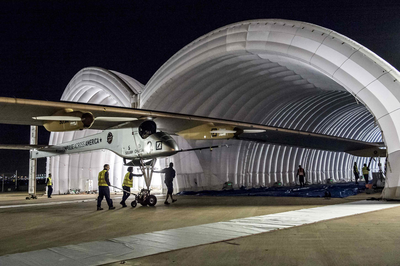] Figure 1: Example of an inflatable hangar - Adopted from 

In order to ensure functionality and safety, prior tests regarding the structure's behavior within the environmental fluid, i.e. an air flow, are essential. Here the investigation of the respective fluid-structure-interaction is of particular importance due to the lightweight concept being strongly affected by e.g. wind loads. Physical tests in this regards are, however, very costly since the lightweight concept does typically not allow for any scaling of the model to smaller sizes. This means, that a physical test always requires a very cost-intensive full-scale model. That is the reason why people are particularly interested in the powerful as well as resource- and cost-efficient virtual design analysis, which in this case means a computational analysis of the fluid-structure interaction (FSI).

Requirement for the corresponding coupled simulation, though, is the ability to deal with large deformations, wrinkling or folding, respectively. To this end a simulation technology based on the method of embedded domains1 is developed at the International Center of Numerical Methods in Engineering (CIMNE). The method of embedded domains is an alternative methodology for the computation of partial differential equations and as such offers the interesting advantage of efficiently dealing with complex boundaries and large deformations where a body-fitted technique like the Arbitrary Lagrangian-Eulerian method (ALE) for examples uses an often expensive moving domain discretization (“Moving Mesh”). Particularly in the scope of fluid-structure interaction analysis, the embedded methods allows to separately handle the different physical entities without having to account for a specific interface model. Instead different overlapping discretizations ("embedded meshes") are used. See figure 2 for an illustration of the different approaches.

In this context, the goal of the present monograph is 1) the further development of the at CIMNE developed method of embedded domains such that it may be used for large-scale CFD and FSI problems and 2) the evaluation of the method compared to the well-known ALE approach. In doing so the tasks were split into two key topics: first the interface tracking in the embedded case and second the setup and comparison of the FSI solution procedure in both cases. For the interface tracking in the embedded method, level set techniques were to be implemented and verified. In terms of the solution procedures the goal was to develop and implement different mesh-updating strategies in the ALE-case as well as mapping techniques for the embedded method. Hence different test scenarios of coupled fluid-structure problems were to be developed, set up and simulated in order to finally compare the methods. For the sake of software modularity focus was here set on partitioned solution techniques. Furthermore in order to keep the computational costs of the coupled analyses as low as possible, parallelization techniques ought to be applied throughout the entire implementation phase.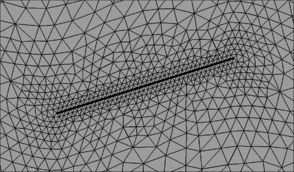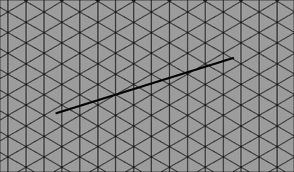(a) Body-fifted approach (Explicit interface model) (b) Embedded approach (Background mesh) Figure 2: Different approaches in a coupled fluid-structure interaction analysis - The figure shows an example structure within a surrounding fluid domain.

The software environment was generally given by the CIMNE in-house multiphysics finite element solver “Kratos”. For the partitioned analysis furthermore the simulation environment EMPIRE (“Enhanced Multi Physics Interface Research Engine”, Technical University Munich) was to be used. So an additional specification arising from this context required the set up of an interface between both software frameworks in order to be able to use the full functionality of EMPIRE together with all features in Kratos. Perspectively the goal is to use Kratos via EMPIRE together with the at the Technical University Munich (TUM) developed structural solver “Carat++” in a common partitioned FSI-environment. This shall allow to combine and consolidate capabilities of either software package and hence the knowledge of either of the related research groups.

Based on all the aforementioned goals, the present monograph is organized as follows: In the first section (chapter 2 to 4) the theoretical background regarding the analysis of coupled fluid-structure problems is given. Here it starts with a discussion of the single field problems which subsequently is extended and merged to the fundamentals of coupled fluid-structure analyses. In both cases the above stated and newly in Kratos implemented method of embedded domains is introduced in detail. Part of the theoretical framework is also a discussion of how in both approaches the computational efficiency may be improved. This includes the presentation of parallelization techniques as well as spatial search algorithms specifically applied in case of the embedded method.

In the second section then (chapter 5 to 6) the different applied software packages as well as the corresponding software interface are described. Here the contents are presented in a very application oriented way in order to provide a documentation for future users.

In the third section (chapter 7) the first key topic of the present monograph is elaborated, i.e. the interface tracking in the scope of the embedded method. Here we answer the questions regarding how to track the interface with two overlapping meshes and how does this affect the corresponding solution quality. Therefore different geometry examples, from a generic structure to a large scale Formula One car, are investigated. Furthermore in this context, different fluid problems are simulated with the embedded method and subsequently evaluated.

Having discussed how to track the interface in the embedded case and knowing about the situation in a body-fitted approach, the fourth section (chapter 8) is dedicated to the second key topic of this monograph, i.e. the actual solution procedure with fluid-structure simulations using either of the aforementioned methods. Here the different developed process steps are elaborated and evaluated in detail giving finally a complete overview of the entire solution process in both cases. With all the implementations then at hand, two solution examples of fully coupled problems are presented which eventually allows for a comparison of the two different approaches.

Finally all the results are briefly summarized and contrasted to the above stated goals.

In order to facilitate the understanding of the later presented developments, the two process flows corresponding to the two different solution approaches as they were established in Kratos, shall be outlined here in advance (3, 4)2. For now, they shall just give an idea about the necessary steps to establish a coupled fluid-structure simulation using one of the above mentioned approaches. In the later course of this monograph then, whenever a feature is discussed or developed, its integration into the overall process is illustrated by means of these two charts. This allows to see importance and impact of single developments in a more general context.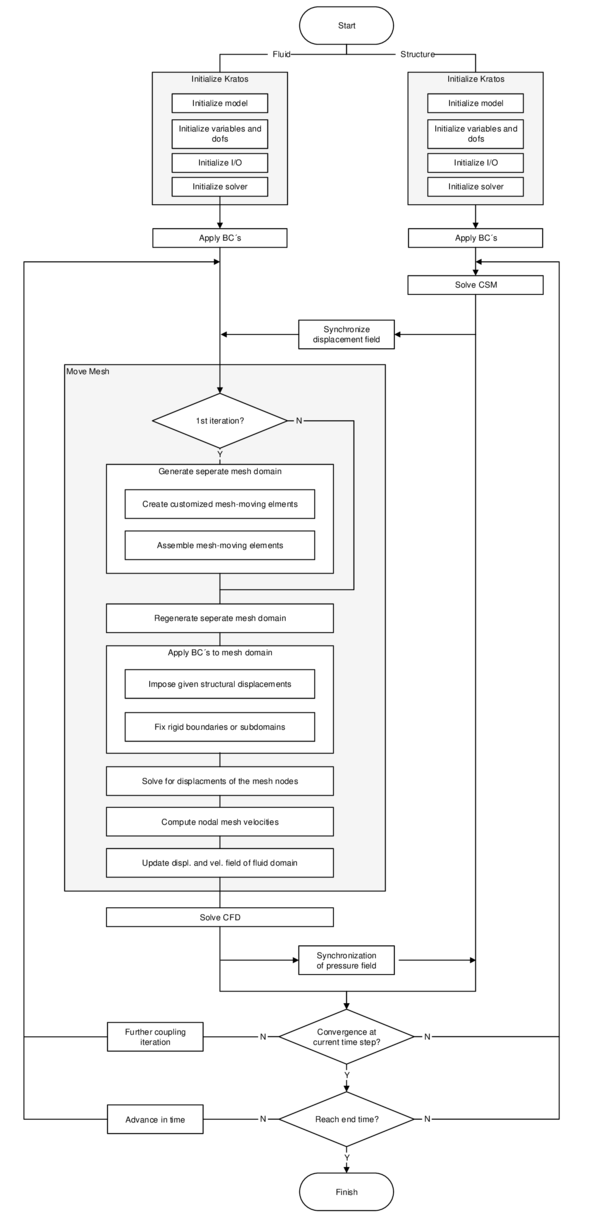Figure 3: Partitioned FSI simulation using the ALE approach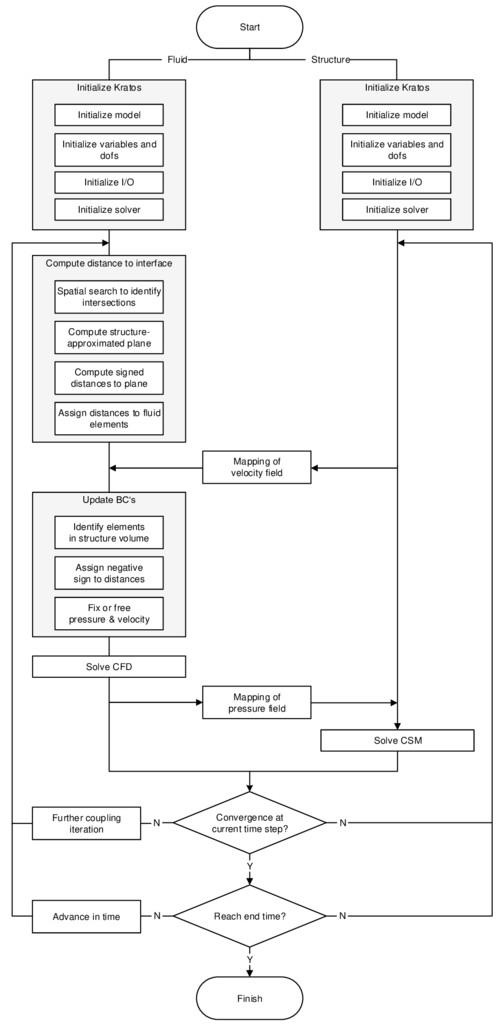Figure 4: Partitioned FSI simulation using the embedded approach

(1) In literature also called “immersed” or “fix-grid” methods

(2) Note that both of the depicted processes show a partitioned analysis

# 2 Fluid and structure as uncoupled fields

In the following the mechanical fundamentals of fluids and structures shall be discussed together with their numerical treatment, i.e. their spatial discretization via FEM, their time discretization using different time integration schemes and their solution by some selected procedures. Both fluid and structure will be regarded as a continuum. Consequently their formulation will be similar and based on classical continuum mechanics. Given this assumption, the chapter will start with a brief introduction into the general description of motion according to basic continuum mechanics. Actual differences between structures and fluids from the point of view of their mechanical description will be elaborated in the later course of the chapter. Afterwards the method of embedded domains will be introduced into the context of classical fluid mechanics. Particularly the relevant element formulation will be of interest here. A discussion of how to impose corresponding boundary conditions will finally close the chapter.

## 2.1 Lagrangian and Eulerian description of motion

In continuum mechanics there are different ways to describe motion. We will focus in this context on the most established ones for each the structure and the fluid. For detailed information in this context, the reader is referred to the classical textbooks of Malvern  or Donea  and Cengel .

In the referential description the motion is described with respect to a reference configuration in which a particle ${\textstyle X}$ of the continuum is located on the position ${\textstyle {\boldsymbol {X}}}$ at time ${\textstyle t}$. It is called Lagrangian description when the reference configuration coincides with the initial configuration at ${\textstyle t=0}$. In elasticity theory the initial configuration is typically chosen to be the unstressed state. Within the Lagrangian viewpoint, one keeps track of the motion of an individual material particle by recording the position ${\textstyle {\boldsymbol {x}}}$ of a particle ${\textstyle X}$ at a time ${\textstyle t}$ linking its material coordinates ${\textstyle {\boldsymbol {X}}}$ to the spatial coordinates ${\textstyle {\boldsymbol {x}}}$ via the function ${\textstyle \chi }$:

 ${\boldsymbol {x}}=\chi (\mathbf {X} ,t).$
(2.1)

Computationally this means that each individual node of a discretized domain is permanently attached to an associated material particle at any point of time as shown in figure 5. When the motion results in large deformations and therefore large distortions of the computational mesh, this method approaches a limit and might even fail due to excessively distorted finite elements which are linked to the material particles.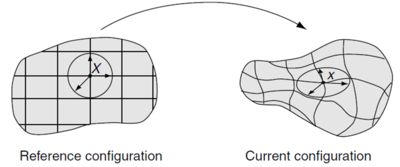Figure 5: Lagrangian viewpoint - The computational grid follows the material particles in the course of their motion (adapted from ).

On the contrary, the spatial description - also called the Eulerian description - avoids such difficulties by considering a control volume fixed in space. In that case the continuum is moving and deforming relatively to the discretized domain of the control volume. We do not keep track of the motion of an individual material particle, rather we observe how the flow field at the fixed computational mesh nodes is changing over time by introducing field variables within the control volume. For example, the spatial description of the velocity field can be defined as a field function ${\textstyle \mathbf {g} }$ of the spatial coordinates ${\textstyle {\boldsymbol {x}}}$ and the time instant ${\textstyle t}$:

 ${v}=\mathbf {g} ({\boldsymbol {x}},t).$
(2.2)

In this equation it becomes obvious that there is no link to the initial configuration or the material coordinates ${\textstyle {\boldsymbol {X}}}$. Moreover, the velocity ${\textstyle {\boldsymbol {v}}}$ of the material at a given node of the computational grid is associated to the velocity of the material particle coinciding with the node. It is also possible to conclude from the flow field at given nodes to the total rate of change of the flow field when following a particle that moves through the fluid domain. This is done via the material derivative which basically links the Eulerian and the Lagrangian description. Applied to a pressure field, the relation is as follows:

 ${Dp}{Dt}={\frac {\partial p}{\partial t}}+{\boldsymbol {v}}\cdot \nabla p,$
(2.3)

or correspondingly for the velocity field, it reads:

 ${\frac {D{\boldsymbol {v}}}{Dt}}={\frac {\partial {\boldsymbol {v}}}{\partial t}}+{\boldsymbol {v}}\cdot \nabla {\boldsymbol {v}}.$
(2.4)

The first part on the right hand side is called the local or unsteady term describing the local rate of change and the second part is the convective term constituting the rate of change of a particle when it moves to a region with different pressure or velocity.

Table 1 is supposed to contrast the most significant advantages and disadvantages of the two descriptions of motion. In order to combine the advantages of both Lagrangian and Eulerian description of motion the so-called Arbitrary Lagrangian-Eulerian method (ALE) has been developed. This method will be treated in chapter 3.2.2.

 Lagrangian description Eulerian description Allows to keep track of the history-dependent material behavior and to back-reference from the current configuration to the initial configuration of any material particle. Does not permit to conclude from the current configuration to the initial configuration and the material coordinates ${\textstyle {\boldsymbol {X}}}$. The coincidence of material particles and the computational grid affects that the convective term drops out in the material derivative 2.4 leading to a simple time derivative. The computational mesh is decoupled from the motion of material particles resulting in a convective term (see formula 2.4). The numerical handling of such a convective term leads to difficulties due to its nonsymmetric character. Following the motion of the particles might lead to excessive distortions of the finite elements when no remeshing is applied what in turn can cause numerical problems during simulation. Due to the fixed computational mesh there are no distortions of finite elements such that large motion and deformation in the continuum can be analyzed. Is mostly used in structure mechanics, free surface flow and simulations incorporating moving interfaces between different materials (e.g. FSI). Application especially in the field of fluid mechanics (e.g. simulation of vortices). Following free surfaces and interfaces between different materials comes along with larger numerical effort.

## 2.2 Computational fluid mechanics

Within this chapter the fundamental formulation of the fluid mechanical problem, that is applied throughout this monograph, shall be introduced. Thereby we will first discuss the governing equations, their numerical discretization in space and time as well as an established solution procedure. Unless stated elsewhere, we will restrict ourselves to a Eulerian description of motion. Furthermore in this context we will focus on finite element techniques as well as the fractional step solution method, which both accounts for the later application in problems related to fluid-structure interaction. In all explanations we will closely follow the theoretical basics given in , the implementation and solver related details from chapter 3 in  and some specific research results in terms of the finite element method for fluid analysis elaborated in [7,8].

In the second part then a newly developed embedded approach shall be introduced, with which simulations, comprising highly deforming structures in a CFD context, shall be eased significantly. In here we will explain, based on the finite element method, both the new modeling technique and its corresponding immanent assumptions. It shall be of particular importance here that the latter assumptions are introduced critically and if necessary linked to corresponding investigations contained in the later course of the present monograph. All of the explanations will furthermore be kept general in that sense that it can be easily transferred from a shear CFD to a fully coupled FSI analysis.

### 2.2.1 Governing equations

The first step in describing the mechanics of a material, including fluids, is the assumption about the underlying material model. We will in the following rely on the continuum assumption which models the material as a continuous mass rather than for instance as discrete particles1. Based on this assumption any material is governed by the conservation of linear momentum:

 ${\frac {\partial }{\partial t}}\rho {\boldsymbol {v}}+\nabla (\rho {\boldsymbol {v}}\otimes {\boldsymbol {v}})+\nabla \cdot {\boldsymbol {\sigma }}-\rho {\boldsymbol {b}}=0,$
(2.5)

the conservation of mass:

 ${\frac {\partial }{\partial t}}\rho +\nabla \cdot \rho {\boldsymbol {v}}=0,$
(2.6)

and the conservation of energy.

 ${\frac {\partial \rho E}{\partial t}}+\nabla \cdot ({\boldsymbol {v}}(\rho E+p^{*}))=\rho {\boldsymbol {g}}\cdot {\boldsymbol {v}}+Q+\Psi +\nabla \cdot {\boldsymbol {q}},$
(2.7)

where ${\textstyle {\boldsymbol {v}}}$ is the velocity vector, ${\textstyle \rho }$ is the density, ${\textstyle p^{*}}$ is the physical pressure, ${\textstyle {\boldsymbol {b}}}$ the body force vector, ${\textstyle E}$ the sum of internal and kinetic energies, ${\textstyle {\boldsymbol {q}}}$ the heat flux vector, and ${\textstyle Q}$ and ${\textstyle \Psi }$ are the internal heat generation and internal heat dissipation function, respectively. The equations here are written in conservative form, which means they are arranged such, that they actually show that the overall change of a quantity is zero, i.e. the quantity is conserved. Note that the system is completely coupled, i.e. a solution of one equation is not possible without taking into account all the others.

Now we will introduce the following assumptions: 1) All state variables are continuous in space so their derivatives exist, 2) the fluid is considered to be incompressible, which yields

 ${\frac {\partial \rho }{\partial t}}=0\quad ,\quad \nabla \cdot \rho {\boldsymbol {v}}=\rho \nabla \cdot {\boldsymbol {v}},$
(2.8)

and 3) the fluid is considered Newtonian, with constant viscosity, where

 ${\boldsymbol {\sigma }}=2\mu \nabla ^{S}{\boldsymbol {v}}-p^{*}{\boldsymbol {I}}=\mu \nabla ^{2}{\boldsymbol {v}}+\mu \nabla (\nabla \cdot {\boldsymbol {v}})-p^{*}{\boldsymbol {I}}.$
(2.9)

Here, ${\textstyle \sigma }$ describes the Cauchy stress, ${\textstyle \mu }$ the dynamic viscosity, ${\textstyle \nabla ^{S}}$ the symmetric part of the velocity gradient and ${\textstyle {\boldsymbol {I}}}$ is the identity matrix. In fact the latter assumption about the material constitution represents the only major difference between the description of a fluid, as it is done here, and the description of a structure whose constitutive relation is typically given in the form ${\textstyle {\boldsymbol {\sigma }}={\boldsymbol {C}}{\boldsymbol {\epsilon }}}$. Note that the latter is based on the actual strains ${\textstyle {\boldsymbol {\epsilon }}}$ rather than the strain rates ${\textstyle {\frac {\partial {\boldsymbol {\epsilon }}}{\partial t}}}$ as they are implied in 2.9.

A consequence of these assumptions is, that the energy conservation is not anymore necessary to sufficiently describe the mechanical system. Thus for an incompressible flow, instead of four governing equations (conservation of momentum, mass and energy as well as the constitutive equation) we only have two simplified partial differential equations with the two independent state variables ${\textstyle p^{*}}$ and ${\textstyle {\boldsymbol {v}}}$2. The remaining two equations with all the above assumptions included are known as the incompressible Navier-Stokes equations (NSE), which are typically given in the non-conservative form:

 ${\frac {\partial {\boldsymbol {v}}}{\partial t}}+({\boldsymbol {v}}\cdot \nabla ){\boldsymbol {v}}-\nu \nabla ^{2}{\boldsymbol {v}}+\nabla p={\boldsymbol {b}}$
(2.10.a)

 $\nabla \cdot {\boldsymbol {v}}=0$
(2.10.b)

Here ${\textstyle \nu =\mu /\rho }$ is the kinematic viscosity and ${\textstyle p=p^{*}/\rho }$ the kinematic pressure. Note that the NSE describe a non-linear, coupled dynamic system.

Eventually we have the choice to either express ${\textstyle {\boldsymbol {v}}}$ w.r.t. a stationary coordinate system, where ${\textstyle {\boldsymbol {v}}}$ corresponds to the physical velocity at a given fixed point in space (Eulerian approach) or a moving coordinate system (Lagrangian approach), where ${\textstyle {\boldsymbol {v}}}$ is determined from the point of view of the moving fluid particle. These approaches can also be combined in a generalized formulation in order to be able to resolve the dynamics of the different domains in an FSI context, as we will see later.

To ensure that the system has a unique solution and to make the problem well posed, it is finally necessary to prescribe exactly one boundary condition at each the Neumann and the Dirichlet boundary. The NSE hence pose a classical boundary value problem where a strong imposition of the latter boundary conditions reads:

 ${\boldsymbol {v}}={\boldsymbol {v}}_{D}\qquad \forall ~{\boldsymbol {v}}~on~\Gamma _{D}$
(2.11.a)

 $-p{\boldsymbol {n}}+2\nu {\boldsymbol {n}}\cdot \nabla ^{S}{\boldsymbol {v}}=t_{N}\qquad \forall ~t_{N}~on~\Gamma _{N}$
(2.11.b)

The assumption of initial conditions in the form

 ${\boldsymbol {v}}({\boldsymbol {x}},0)={\boldsymbol {v}}_{0}({\boldsymbol {x}})\quad \quad \forall ~{\boldsymbol {v}}~in~\Omega$
(2.12.a)

 ${\boldsymbol {p}}({\boldsymbol {x}},0)={\boldsymbol {p}}_{0}({\boldsymbol {x}})\quad \quad \forall ~{\boldsymbol {p}}~in~\Omega$
(2.12.b)

completes the problem formulation. Having now mathematically formalized the underlying physics. We have to discretize the NSE in order to solve it numerically.

(1) A famous particle-based model in computational fluid dynamics is e.g. the Lattice-Boltzmann-Method.

(2) Note that the velocity is a three-dimensional vector actually resulting in four independent state variables. The number of equations within the NSE increases accordingly.

### 2.2.2 Discretization

The NSE can be discretized in various ways both in time and space. All solutions throughout this monograph, though, were computed based on a finite element discretization in space and finite difference schemes in time which is why the latter techniques shall be introduced in the following. We will thereby follow an idea which in the literature is called the method of lines.

The method of lines is a usual practice in finite analysis of time-dependent problems. In here we are first discretizing with respect to the spatial variables from which we obtain a system of coupled first-order ordinary differential equations (with respect to time). The latter is called the semi-discrete system. Then to complete the discretization of the original PDE, we integrate the first-order differential system forward in time to trace the temporal evolution of the solution starting form the initial point ${\textstyle {\boldsymbol {v}}_{0}({\boldsymbol {x}})}$.

#### 2.2.2.1 Spatial discretization

Within this section it is assumed that the reader is familiar with the basic concepts of the finite element method using variational calculus.

Given the NSE, its weak form is obtained by multiplying 2.10.a and 2.10.b with the test functions ${\textstyle {\boldsymbol {\omega }}}$ and ${\textstyle q}$ respectively. The corresponding weighted residual formulation reads:

 $\int _{\Omega }\left({\frac {\partial {\boldsymbol {v}}}{\partial t}}+({\boldsymbol {v}}\cdot \nabla ){\boldsymbol {v}}-\nu \nabla ^{2}{\boldsymbol {v}}+\nabla p\right)\cdot {\boldsymbol {\omega }}d\Omega =\int _{\Omega }\left({\boldsymbol {b}}\right)\cdot {\boldsymbol {\omega }}~d\Omega$
(2.13.a)

 $\int _{\Omega }\left(\nabla \cdot {\boldsymbol {v}}\right)q~d\Omega =0$
(2.13.b)

By performing an integration by parts of the viscous term and the pressure gradient term in the momentum balance and each applying the divergence theorem the final weak form of the NSE is obtained as:

 $\int _{\Omega }{\frac {\partial {\boldsymbol {v}}}{\partial t}}\cdot {\boldsymbol {\omega }}~d\Omega -\int _{\Omega }\nu \nabla {\boldsymbol {u}}\cdot \nabla {\boldsymbol {\omega }}~d\Omega -$ $\int _{\Omega }p\cdot \nabla {\boldsymbol {\omega }}~d\Omega =\int _{\Omega }{\boldsymbol {b}}\cdot {\boldsymbol {\omega }}~d\Omega +\int _{\Gamma _{N}}{\boldsymbol {\omega }}\cdot {\boldsymbol {t}}_{N}~d\Gamma _{N}$
(2.14.a)

 $\int _{\Omega }\left(\nabla \cdot {\boldsymbol {v}}\right)q~d\Omega =0$
(2.14.b)

It can be seen from the equation, that by this approach we have naturally produced a Neumann boundary which is now given in a weak formulation as:

 $\int _{\Gamma _{N}}{\boldsymbol {\omega }}\cdot {\boldsymbol {t}}_{N}~d\Gamma _{N}=\int _{\Gamma _{N}}{\boldsymbol {\omega }}\cdot \left[-p{\boldsymbol {n}}+2\nu {\boldsymbol {n}}\cdot \nabla ^{S}{\boldsymbol {v}}\right]~d\Gamma _{N}$
(2.15)

where ${\textstyle {\boldsymbol {n}}}$ describes the boundary normal. In this weak formulation the Dirichlet boundary terms vanish since the test-functions ${\textstyle {\boldsymbol {\omega }}}$ and ${\textstyle q}$ are by definition zero on the Dirichlet boundary. This implies that, given that ${\textstyle {\boldsymbol {v}}_{D}}$ is part of the solution of the NSE, the Dirichlet boundary conditions are automatically fulfilled. One important practical example of a Dirichlet boundary condition is the application of no-slip-conditions on walls.

At this point it shall be mentioned that for the imposition of slip boundary conditions by contrast, we may partially integrate 2.13.b such that we get:

 $\int _{\Omega }\left(\nabla \cdot {\boldsymbol {v}}\right)q~d\Omega =-\int _{\Omega }{\boldsymbol {v}}\cdot \nabla q~d\Omega +\int _{\Gamma }q{\boldsymbol {v}}\cdot {\boldsymbol {n}}~d\Gamma$
(2.16)

Then we split the boundary term in parts where we want to enforce slip-conditions and parts where we do not. This may read:

 $\int _{\Gamma }q{\boldsymbol {v}}\cdot {\boldsymbol {n}}~d\Gamma =\int _{\Gamma _{slip}}q{\boldsymbol {v}}\cdot {\boldsymbol {n}}~d\Gamma _{slip}+\int _{\Gamma _{rest}}q{\boldsymbol {v}}\cdot {\boldsymbol {n}}~d\Gamma _{rest}$
(2.17)

By simply omitting the computation of the slip-boundary integral during the simulation, i.e.

 $\int _{\Gamma _{slip}}q{\boldsymbol {v}}\cdot {\boldsymbol {n}}~d\Gamma _{slip}=0,$
(2.18)

we imply that the velocities ${\textstyle {\boldsymbol {v}}}$ along this parts of the boundary are in a weak sense perpendicular to the local boundary normals ${\textstyle {\boldsymbol {n}}}$. This basically reflects a tangent sliding of the fluid along the respective walls and hence an imposition of a slip boundary condition.

To finish the spatial discretization we introduce linear shape functions ${\textstyle {\boldsymbol {N}}({\boldsymbol {x}})}$ in order to approximate ${\textstyle {\boldsymbol {v}}}$ and ${\textstyle p}$ as well as ${\textstyle {\boldsymbol {\omega }}}$ and ${\textstyle q}$. Here a Galerkin formulation is used where shape functions and test-functions are of the same kind. By assuming Einstein's summation convention, the approximation reads:

 $p({\boldsymbol {x}})=\sum _{i}N_{i}({\boldsymbol {x}})\cdot p_{i},$
(2.19)

 ${\boldsymbol {v}}({\boldsymbol {x}})=\sum _{i}N_{i}({\boldsymbol {x}})\cdot {\boldsymbol {v}}_{i},$
(2.20)

 $q({\boldsymbol {x}})=\sum _{i}N_{i}({\boldsymbol {x}})\cdot q_{i}$
(2.21)

 ${\boldsymbol {\omega }}({\boldsymbol {x}})=\sum _{i}N_{i}({\boldsymbol {x}})\cdot {\boldsymbol {\omega }}_{i},$
(2.22)

Plugging these interpolations into the weak form of the NSE given by equation 2.14.a and 2.14.b we obtain the following semi-discrete form of the incompressible NSE:

 ${\boldsymbol {M}}{\frac {\partial {\boldsymbol {v}}}{\partial t}}+{\boldsymbol {C}}({\boldsymbol {v}}){\boldsymbol {v}}-\nu {\boldsymbol {L}}{\boldsymbol {v}}+{\boldsymbol {G}}p={\boldsymbol {F}}$ ${\boldsymbol {D}}{\boldsymbol {v}}=0$
(2.23)

where on elemental level

 ${\boldsymbol {M}}=\rho \int _{\Omega _{e}}{\boldsymbol {N}}{\boldsymbol {N}}^{T}~d\Omega _{e}$
(2.24.a)

 ${\boldsymbol {L}}=\int _{\Omega _{e}}\nabla {\boldsymbol {N}}\nabla {\boldsymbol {N}}^{T}~d\Omega _{e}$
(2.24.b)

 ${\boldsymbol {G}}=-\int _{\Omega _{e}}\nabla {\boldsymbol {N}}{\boldsymbol {N}}~d\Omega _{e}$
(2.24.c)

 ${\boldsymbol {C}}({\boldsymbol {v}})=-\int _{\Omega _{e}}{\boldsymbol {N}}({\boldsymbol {v}}\cdot \nabla {\boldsymbol {N}})~d\Omega _{e}$
(2.24.d)

 ${\boldsymbol {F}}({\boldsymbol {v}})=\int _{\Omega _{e}}{\boldsymbol {N}}{\boldsymbol {b}}~d\Omega _{e}+\int _{\Gamma _{N,e}}{\boldsymbol {n}}(p-\nu \rho \nabla {\boldsymbol {v}})~d\Gamma _{N,e}$
(2.24.e)

 ${\boldsymbol {D}}=-{\boldsymbol {G}}^{T}$
(2.24.f)

Note that the problem is still time dependent. That is, we still need to introduce a time discretization scheme in order to assemble the local contributions to a global system that can be solved. Note also that ${\textstyle {\boldsymbol {v}}}$ and ${\textstyle p}$ here are the approximated quantities of the velocity and the pressure. Since this will be the case for the remainder of the monograph, we will refrain from an indexation for the sake of readability. For the actual computation in an FEM framework, numerical integration methods (such as Gauss-Integration) are necessary to compute the integrals in 2.24.

At the end of this section two basic problems shall be mentioned, that arise in this form of the discretization: The first problem occurs in cases where the convective term is dominant, for example in high-Reynolds or turbulent flows. In these cases the standard Galerkin approach gets unstable. Another numerical difficulty arises from the incompressibility constraint. In incompressible flows the pressure is acting as a Lagrange multiplier that enforces the velocity not to violate the mass conservation in a very strong form. The role of the pressure variable is thus to adjust itself instantaneously to the given velocity field such that the mass conservation holds.This leads to a coupling between the velocity and the pressure unknowns that causes the system in 2.23 to get ill-conditioned or even singular.

Both problems lead to the fact that in an FEM formulated flow problem, the compliance to certain numerical conditions or the application of stabilization techniques are inevitable.

#### 2.2.2.2 Time discretization

Given the first order time dependent, semi-discrete NSE in 2.23, we may use a variety of different methods to integrate in time and hence compute the numerical solution of the NSE. Depending on the application we may therefore use either single step or multistep methods in an explicit or implicit formulation. Famous classes of integration schemes which are based on a single time step are: the list of Runge-Kutta methods or all customized integrators from the Newmark-family. A vast source of detailed information, in particular also in terms of accuracy order or stability, can be found in . For a quick reference about the principal idea of the time integration chapter 3.4.1 in  is recommended.

By contrast, a famous class of integration schemes based on multi-step procedures is given by the different schemes of the Backward Differentiation Formula (BDF). In fact the BDF scheme of second order, i.e. BDF-2, is used by the fractional step solver, with which the solutions throughout this monograph were generated.

In the BDF-2 scheme, unlike in other multi-step integration variants, we, for a given function and time, approximate the time derivative of quantities rather than the quantities itself by incorporating information from previous, current and following time-steps. This typically renders this method sufficiently accurate and stable also for numerically stiff problems. The discretization of the time derivative in BDF-2 reads:

 $\left.{\frac {\partial {\boldsymbol {v}}}{\partial t}}\right|_{t_{n+1}}=C_{1}{\boldsymbol {v}}_{t_{n+1}}+C_{2}{\boldsymbol {v}}_{t_{n}}+C_{3}{\boldsymbol {v}}_{t_{n-1}}$
(2.25)

with the coefficients

 $C_{1}={\frac {3}{2\Delta t}},$
(2.26)

 $C_{2}=-{\frac {2}{\Delta t}},$
(2.27)

 $C_{3}={\frac {1}{2\Delta t}},$
(2.28)

If we now introduce the time discretization into the semi-discrete local systems, i.e. we plug 2.25 in 2.23, and furthermore replace in the latter equation the continuous quantities ${\textstyle {\boldsymbol {v}}}$ and ${\textstyle p}$ by their time discrete correspondents ${\textstyle {\boldsymbol {v}}_{n+1}}$ and ${\textstyle p_{n+1}}$, we obtain the fully discretized local system in block-matrix form as:

 $\left[{\begin{array}{cc}{\frac {3}{2\Delta t}}{\boldsymbol {M}}+{\boldsymbol {C}}({\boldsymbol {v}}_{n+1})-\nu {\boldsymbol {L}}&{\boldsymbol {G}}\\{\boldsymbol {D}}&{\boldsymbol {0}}\\\end{array}}\right]\left[{\begin{array}{cc}{\boldsymbol {v}}_{n+1}\\p_{n+1}\end{array}}\right]=\left[{\begin{array}{cc}{\boldsymbol {F}}+{\frac {2}{\Delta t}}{\boldsymbol {M}}{\boldsymbol {v}}_{n}-{\frac {1}{2\Delta t}}{\boldsymbol {M}}{\boldsymbol {v}}_{n-1}\\{\boldsymbol {0}}\end{array}}\right]$
(2.29)

Finally the elemental contributions can be assembled to a global discrete system that may be solved iteratively using the fractional step method. In fact the combination of the BDF-2 scheme with a fractional step solution procedure poses an established compromise between accuracy, stability and computational costs in an FEM environment.

### 2.2.3 Fractional step solution

Having the discrete system in equation 2.29, we want to solve for ${\textstyle {\boldsymbol {v}}_{n+1}}$ and ${\textstyle p_{n+1}}$. In practical examples this system is typically very large comprising up to several millions degrees of freedom, which renders the application of accurate and robust direct solution techniques very inefficient or even impossible. Iterative solvers by contrast are known to behave very efficient with large problems, which is why it might be preferable to use them for a solution. Iterative solvers, however, tend to severe robustness problems with badly conditioned systems. The critical conditioning that may arise from the convective term and the incompressibility constraint, as they were described above, hence require specialized iterative techniques, such as the fractional step method. In the following the idea of the latter shall be sketched briefly. For a detailed derivation, the reader is referred to chapter 3.8 in .

The idea of the fractional step method is to split the overall monolithic solution of 2.29 into several steps, such that each step contains a well-conditioned subsystem that can be solved more efficiently. In order to do so an estimate ${\textstyle {\boldsymbol {\tilde {v}}}_{n+1}}$ of the velocity field is introduced and hence the convective term is approximated as

 ${\boldsymbol {C}}({\boldsymbol {v}}_{n+1}){\boldsymbol {v}}_{n+1}={\boldsymbol {C}}({\boldsymbol {\tilde {v}}}_{n+1}){\boldsymbol {\tilde {v}}}_{n+1},$
(2.30)

which is a distinct assumption causing the fractional step method to only deliver an approximate solution of ${\textstyle {\boldsymbol {v}}_{n+1}}$. The approximation, though, converges to the exact solution as ${\textstyle \Delta t}$ tends to zero and is hence a valid assumption for small time steps.

Using the previous assumptions and introducing a time integration scheme as described in the previous section, the following solution steps can be derived:

Step 1:

 ${\boldsymbol {M}}{\frac {{\tilde {\boldsymbol {v}}}_{n+1}-{\boldsymbol {v}}_{n}}{\Delta t}}+{\boldsymbol {C}}({\tilde {\boldsymbol {v}}}_{n+1}){\tilde {\boldsymbol {v}}}_{n+1}-\nu {\boldsymbol {L}}{\tilde {\boldsymbol {v}}}_{n+1}+\gamma {\boldsymbol {G}}p_{n}={\boldsymbol {F}}_{n+1}$
(2.31.a)

Step 2:

 $\Delta t{\boldsymbol {D}}{\boldsymbol {M}}^{-1}{\boldsymbol {G}}(p_{n+1}-\gamma p_{n})={\boldsymbol {D}}{\tilde {\boldsymbol {v}}}_{n+1}$
(2.31.b)

Step 3:

 ${\boldsymbol {M}}{\frac {{\boldsymbol {v}}_{n+1}-{\tilde {\boldsymbol {v}}}_{n+1}}{\Delta t}}+{\boldsymbol {G}}(p_{n+1}-\gamma p_{n})=0$
(2.31.c)

where ${\textstyle \gamma }$ is a numerical parameter, whose values of interest are ${\textstyle 0}$ and ${\textstyle 1}$. In order to keep the equations simple, instead of the above discussed BDF-2 scheme we have chosen a BDF-1 time discretization1 where

 $\left.{\frac {\partial {\boldsymbol {v}}}{\partial t}}\right|_{t_{n+1}}={\frac {1}{\Delta t}}{\boldsymbol {v}}_{t_{n+1}}-{\frac {1}{\Delta t}}{\boldsymbol {v}}_{t_{n}}.$
(2.32)

Given the steps above the fractional step iteration rule reads:

1. Given ${\textstyle p_{n}}$ and ${\textstyle {\boldsymbol {v}}_{n}}$ we can solve for the velocity estimate ${\textstyle {\boldsymbol {\tilde {v}}}_{n+1}}$ by means of 2.31.a.
2. Having ${\textstyle p_{n}}$ and ${\textstyle {\boldsymbol {\tilde {v}}}_{n+1}}$ we use them in 2.31.b to solve for ${\textstyle p_{n+1}}$
3. Having ${\textstyle p_{n}}$, ${\textstyle {\boldsymbol {\tilde {v}}}_{n+1}}$ and ${\textstyle p_{n+1}}$ we compute in 2.31.c the approximated solution ${\textstyle {\boldsymbol {v}}_{n+1}}$. If convergence not achieved, start again at 1.

The fractional step method as described here has different properties making it very interesting for the application in a FEM-based framework for the solution of incompressible flows. An important property for example is the improved stabilization in case of a badly-conditioned system. This property is a consequence of computing several well-conditioned steps instead of solving a monolithic ill-conditioned system at once. It is worthwhile to note that this holds even without any explicit implementation of stabilization terms. Another important property is the reduced computational effort due to the fact that the single steps are typically converging much faster compared to the time that is needed for convergence of the overall monolithic system. Finally it shall be mentioned, that the fractional step method allows a very natural introduction of the structural contribution during the solution of an FSI problem. This will also hold for the case of the embedded approach, as it will be shown later.

(1) Note that the BDF-1 discretization exactly represents a first order implicit Euler-scheme.

### 2.2.4 Embedded formulation

Within this chapter the fundamentals of a new embedded formulation shall be discussed. Thereby all the explanations will be kept general in the sense that an extension of this method to an FSI-scenario can be easily understood. By that we want to clearly emphasize the underlying model capabilities with regards to both a pure CFD analysis and a fully coupled simulation of fluid-structure interactions.

The organization of this chapter is oriented at the successive tasks necessary to set up an embedded environment. That is, in the first part it is discussed how different types of structures may be in general embedded and hence approximated within a background fluid mesh. In this context it will be of particular interest how a voluminous body differs from other types of structures. Having approximated the embedded model, a new element formulation will be introduced that is capable of dealing with the embedded boundaries. This in particular refers to the discontinuity that arises at respective borders. Finally it shall be shown how boundary conditions, that appear as a consequence of the present structure, are imposed on the fluid.

An important aspect throughout all the given sections will be the elaboration and distinct presentation of all the fundamental approximations based on which the embedded formulation was designed. An investigation of their impact will then follow in chapter 7 and 8.

#### 2.2.4.1 Embedding open and closed structures

Embedding a structure into a fluid domain requires a mathematical description of the former relative to the latter. This is typically done by using, what in literature is often referred to as, level set methods. The embedded approach we are following here is a level set method in that sense, that we are tracking the motion of an arbitrary interface within a surrounding domain by embedding the interface as the zero level set of a given distance function. The surrounding domain in our case is described by a fluid model whereas the interface corresponds to the physical connection of the given fluid to an embedded structure.

All embedded approaches of that kind have in common, that they are approximating the embedded domain by means of some distance function. Thereby the embedded domain is typically given in a discrete form. For example when we are embedding a structure into a fluid mesh, we are classically using the discrete description of the structure, i.e. its FE-mesh. That means for the embedded method, however, that not the actual structure will be approximated by the distance function but its discretization, which in all cases results in a further level of approximation, that is not present in a body-fitted formulation. It is thus important to note at first, that this additional approximation level leads to an immanent error which only can be reduced but not avoided. A detailed evaluation of the latter will follow in later the course of this monograph.

As already indicated, embedding the structure into a background fluid mesh implies classically a distance function which describes the spatial distance between points in both domains such that we are able to identify the common interface. The development of a respective distance function is one focus of this monograph and will be elaborated in detail in chapter 7. In this section we will be rather more interested in the more general question of how to embed into a given domain both open structures like membranes or shells, where we only have an exterior flow, and closed or voluminous structures like bluff bodies or the inflatable Hangar from the beginning, where the interior and exterior part of the fluid needs to be treated differently.

In order to be able to embed both types of structures, first the idea of the level set method needs to be generalized. To this end two distance functions are used, instead of just relying on one. So conceptually there is

1. a discontinuous distance function in order to identify and keep track of the position of an embedded interface within a background fluid mesh and
2. a continuous distance function which allows to classify fluid nodes as either “inside” or “outside” the embedded structure.

Note that the latter distinction is only necessary given that the embedded structure is voluminous.

In the first case, so for the identification of the embedded interface, the idea is to use a signed distance function which associates to each node in a given cut fluid element a signed distance to the given embedded structure. The sign is thereby chosen according to the normal orientation of the structure. By that we are able to numerically distinguish between the structure's positive and negative side. Figure 6 illustrates the concept at a 2D example. Having the signed distance values on all nodes of a cut fluid element we can then reproduce the intersection points and approximate the actual embedded interface by means of techniques that are going to be introduced later.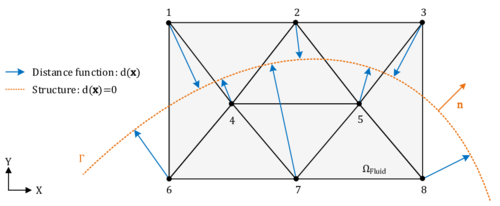Figure 6: Concept of a distance function within a level set approach

This procedure of computing signed distances and tagging the surrounding fluid nodes with the corresponding values is performed for each cut fluid element independently as indicated in figure 7. That is, the signed distances of the first distance function are not considered to be nodal quantities such as the velocities or displacements, but rather more elemental ones. Due to this customization of the original level set method, the approximation of the embedded interface becomes a purely local operation. Besides of some very nice computationally advantageous aspects, this localization leads to an important characteristic of this first distance function: Since the distances are elemental quantities, different distances may be given at one physical node, which in turn means that there will be not necessarily a continuous representation of the embedded structure as can be guessed from figure 7.

What at a first glance might look very rough has in fact a lot of advantages from which the most important advantage is the possibility to deal with several discontinuous structures within one fluid mesh as may be easily understood from figure 8.

In here a point ${\textstyle A}$ is shown, that, depending on which structure it is referred to, should have a positive distance according to the red triangle but a negative one when seen as a part of the blue one. If the distance function was continuous and the distances were nodal quantities, node ${\textstyle A}$ would clearly not be able to describe both structure parts at once which is, however, necessary obviously to correctly represent it. With the distance function being discontinuous by contrast, the embedded structure in each of the highlighted triangles can be reconstructed exactly since the distances are not stored on the nodes but are rather more members of the single elements. In fact this makes the discontinuous approach very powerful, since this implies that we are principally not restricted in terms of possible intersection patterns that may arise across several elements. This is what forms a real embedded approach. In this context it is moreover worthwhile to mention, that the above indicated computational advantages arise just because of this purely local operation, since in such a framework a parallelization of the computation is straight forward and very effective as we will see later.

So in a nutshell, the discontinuous distance function is needed in order to be able to identify the embedded interface without any restriction to certain intersection patterns. Given a voluminous structure, however, it might not be enough to “only” know about the embedded interface. Typically it is also of interest which nodes of the fluid mesh are lying inside the structure and which ones are outside. This in particular is the case when we have inflatable structures for which we might want to treat the closed fluid part in the interior of the structure differently from the environmental fluid. Therefore another indicator to identify “inside” and “outside” is needed.Figure 8: Need for discontinuity in an embedded approach

An obvious indicator for the distinction is again the sign of the distances that are computed. Different from before, however, the nodal distance to the structure is needed, since we want to classify each node as either outside or inside. That is basically why we need a continuous distance function instead of a discontinuous here. Details to their implementation will follow in chapter 7. For now it is only important that this distance function computes for each node a distance to the embedded structure and assigns its sign automatically according to a technique which is based on what in computer graphics is called “ray tracing”. Following this terminology the process of assigning the indicator to the single nodes is typically referred to as “coloring”

The underlying concept with this type of coloring is straightforward: Depending on an sequence of choice, different rays are “shot” through the fluid domain such that they start and end at a node which by definition lies outside. Along their way they assign every trespassed node the indication for “outside”, which we chose to be a positive sign for the respective nodal distance. Whenever a structure boundary is now crossed the rays switch their status and henceforth assign the opposite indication. So if just started the nodal distances will be tagged with a negative sign after the ray crosses a structure boundary, indicating that they belong to the interior. This is done until every fluid node was touched by a ray at least once. As a result we obtain fluid nodes that either have a negative distance to the structure, iff a fluid node is part of the structure's interior domain, or a positive distance else. Figure 9 illustrates the concept.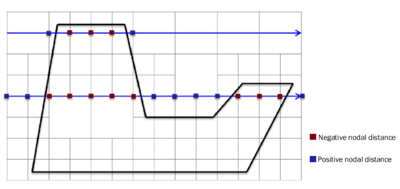Figure 9: Coloring by means of ray tracing

Unfortunately the algorithm in its basic form only works for simple cases, excluding any model defects or similar challenges as shown in figure 10. In order to obtain a robust automatic coloring, additional implementations were necessary which will, however, not be further detailed here.

Assuming a robust coloring technique, we are finally able to apply any model assumptions to the different domains of the fluid, which are point-wise identified with positive or negative distances. Since in a lot of applications the flow in the interior is not of interest, we simply deactivate the corresponding degrees of freedom by setting all the velocities and pressures at nodes with negative distances to zero. By that the respective degrees of freedom are effectively excluded from the overall solution of the fluid.

At the end of this chapter it can be concluded: Using two different distance functions in combination with a powerful coloring technique allows to take into account both voluminous and membrane or shell structures in an embedded environment. It is worthwhile to mention that it is of no importance whether the embedded approach is applied in a CFD or an FSI context.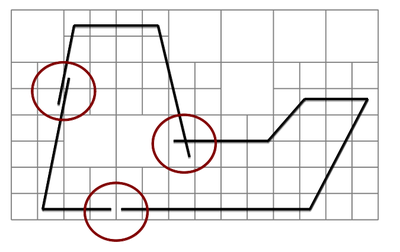Figure 10: Challenges in coloring by means of ray tracing

#### 2.2.4.2 Element technology

Given that the embedded structure is properly represented within the fluid domain by the techniques that were introduced in the previous section, we have to take care about the discontinuities at the embedded interface. To this end we are customizing the element formulation of the one elements that intersect with the structure. Conceptually the idea is here, to add nodes at the interface and to perform a local subdivision. Figure 11 illustrates the concept.

To introduce finally a discontinuity an obvious and easy approach might be to introduce Dirichlet boundary conditions on the newly added nodes. Unfortunately such an approach is by far not robust since the mesh obtained may become arbitrarily bad, i.e. very small and deformed elements might appear, which finally can lead to a severe ill-conditioning of the system.Figure 11: Virtual subdivision of split element

Therefore a different approach was chosen, instead. In there we first duplicate the nodes at the interface and divide the domain into two virtual blocks with each two virtual nodes as shown in the figure 12. Taking into account the later application we distinguish the two new virtual blocks by referring to them as each the positive or negative side of the split fluid element. As a result we may independently describe quantities on the positive side (such as a positive face pressure) or the negative side (negative face pressure), respectively.

The key idea now to solve the conditioning problem is, that instead of giving full freedom to the virtual nodes, we will express the degrees of freedom associated to those nodes as a function of the degrees of freedom of the actual fluid nodes on the respective side of the virtual domain. In our case for instance, we are imposing that the variables on the interface must respect the following constraints:

 $\Phi (a_{+})=\Phi (3)$ $\Phi (b_{+})=\Phi (3)$
(2.33)

and

 $\Phi (a_{-})=\Phi (1)$ $\Phi (b_{-})=\Phi (2)$
(2.34)

where ${\textstyle \Phi }$ represents any degree of freedom and the arguments correspond to the node numbers. This can be graphically understood as illustrated in figure 13.Figure 12: Separation of domain by duplication of nodesFigure 13: Constraints on virtual nodes in a discontinuous element formulation

As might be guessed up to now, an approach as described above would require a further introduction of constraints after the fluid domain was modeled with finite elements. So a better way would be to incorporate such constraints already by construction. This can now be achieved by introducing modified shape functions which indeed span exactly the same space as the one described by the standard finite element space, however, take into account the new nodes and constraints.

Functions with these characteristics were developed for other purposes in the work of Ausas et al. in . The basic idea is thereby as simple as effective: The shape functions of the split fluid element are constructed in the same way as for standard finite elements, however, with the two major differences of 1) each being just defined on one of two separate virtual domains and 2) each containing a vanishing gradient along cut edges in 2D or intersecting faces in 3D. An illustration is given in figure 14.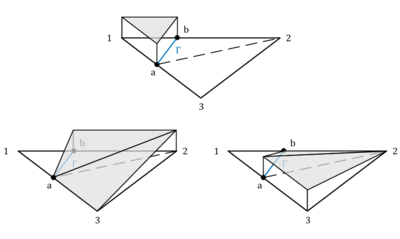Figure 14: Discontinuous shape functions

The numerical integration over the split fluid element domain is eventually done by using the well-known Gauss-Integration method where we simply introduce separate Gauss points on each sub-triangle and integrate for each sub-triangle independently. Figure 15 highlights the idea.

Note that this is a purely local approach where the introduced auxiliary nodes on the interface are not needed, and actually not even seen in the computation of the overall system. In fact all the operations in the embedded approach are purely local with such a formulation, which is very advantageous in terms of high performance computing.

Note also that by using these modified shape functions, the finite element solution along the edges or faces that are not cut, is not changing. This is an important characteristic in that sense, that these elements can be used together with standard finite elements in a common model. As a matter of fact, the only thing that changes in the elements cut by the interface is the kinematic description used in reconstructing the different fields of interest.

Finally it is worthwhile to highlight, that by applying this modified shape function approach within an element, we introduced by construction an element that does not allow any flux over an embedded interface. That is exactly the discontinuity we wanted to implement.

Apart from this discontinuity the modified shape functions are generally ${\textstyle C^{0}}$ continuous between elements. As will be elaborated in the later course of this monograph, however, there are “intersection patterns” in which this does not hold anymore. Anyways the hence introduced error might be still a valid approximation.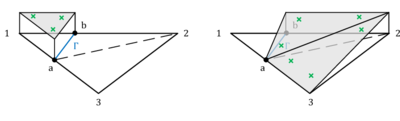Figure 15: Gauss integration in an embedded approach - The figure shows a separate integration for each sub-triangle as well as each side of the split fluid element

#### 2.2.4.3 Velocity boundary conditions

Because of the constraints imposed on the virtual nodes, the shape functions described above have a zero gradient in direction of each edge or face intersected by the structure. While this solves the ill-conditioning problem it implies that the gradient of the shape function normal to the embedded interface is zero. In turn this implies that if we use this modified space to describe the velocities, the only suitable boundary condition for the beginning is “slip”. How the latter is imposed in an embedded scenario is going to be explained in this section. At the end then, we will briefly sketch possibilities how a stick behavior might be introduced at embedded walls.

Given the NSE on elemental level, velocities at the embedded interface are imposed weakly by making use of a partial integration of the weighted mass conservation equation, 2.14.b. Since we have to take into account the positive and the negative side of the cut fluid element independently, we first split the integral domain into the positive and negative virtual subdomain and then perform the integration by parts. Furthermore we subdivide the generated boundary terms into the “standard” element boundaries ${\textstyle \Gamma _{s}}$ and the embedded boundary ${\textstyle \Gamma }$ which all together form the complete boundary of the cut fluid element:

 $\int _{\Omega }\nabla \cdot {\boldsymbol {v}}\cdot q~d\Omega =+\int _{\Omega _{+}}\nabla \cdot {\boldsymbol {v}}\cdot q~d\Omega _{+}+\int _{\Omega _{-}}\nabla \cdot {\boldsymbol {v}}\cdot q~d\Omega _{-}$ $=-\int _{\Omega _{+}}{\boldsymbol {v}}\cdot \nabla \cdot q~d\Omega _{+}-\int _{\Omega _{-}}{\boldsymbol {v}}\cdot \nabla \cdot q~d\Omega _{-}$ $+\int _{\Gamma _{+}}q{\boldsymbol {v}}\cdot {\boldsymbol {n}}~d\Gamma _{+}+\int _{\Gamma _{-}}q{\boldsymbol {v}}\cdot {\boldsymbol {n}}~d\Gamma _{-}$ $+\int _{\Gamma _{s+}}q{\boldsymbol {v}}\cdot {\boldsymbol {n}}~d\Gamma _{s+}+\int _{\Gamma _{s-}}q{\boldsymbol {v}}\cdot {\boldsymbol {n}}~d\Gamma _{s-}$
(2.35)

The volume integrals in here are solved using the Gauss integration for discontinuous fluid elements as described in section 2.2.4.2. The embedded boundary by contrast represents the contact to the embedded structure and is consequently constrained by the structure´s velocity. As we will see in chapter 8.3.3, it is from a practical point of view, however, difficult to introduce a corresponding constraint to the fluid formulation since in general the velocity of the structure is not constant along the embedded boundary. Therefore, in order to significantly ease the computation of the boundary integrals in 2.35, we assume that the velocity is constant along the interface within a split fluid element. This constant velocity is in the following referred to as the “embedded velocity” ${\textstyle {\boldsymbol {v}}_{embedded}}$ and it is obtained by averaging or interpolating the given velocities of the structure inside the respective fluid element. See chapter 8.3.3 for further details in this regard. Furthermore, since we regarded values along intersected edges of the fluid element as constant, as explained in the previous chapter, the embedded velocity is constant throughout the entire cut fluid element and will hence act at each fluid node equally. See figure 16 for an illustration.

Introducing this embedded velocity and having constant values along cut edges obviously makes a proper representation of the boundary layer very difficult. Apart from that, however, it has important advantages when it comes for example to the mapping of the physical quantities between the different domains, as we will see later. Since the discussed embedded method was at first not designed for an application in highly turbulent flows driven by corresponding boundary layers, and since in all other cases the boundary layer is often anyways not resolved but rather more incorporated using wall-functions, we considered this as a valid assumptions.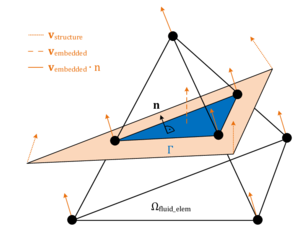Figure 16: Assumption of embedded velocity - Orange depicts the structure that is intersecting the fluid element and leading to the embedded boundary $\Gamma$ in blue. Note that the embedded velocity is a function of the nodal velocities of the structure.

Introducing now the aforementioned assumptions into 2.35, we obtain for the embedded boundary in the fluid:

 $\int _{\Gamma _{+/-}}q{\boldsymbol {v}}\cdot {\boldsymbol {n}}~d\Gamma _{+/-}=\int _{\Gamma _{+/-}}q{\boldsymbol {v}}_{embedded}\cdot {\boldsymbol {n}}~d\Gamma _{+/-},$
(2.36)

which means that inside the cut fluid element the fluid is free to slip along the embedded boundary ${\textstyle \Gamma }$, but the velocity of the fluid in normal direction to the embedded boundary is prescribed by the velocity of the structure along the same direction1. This exactly corresponds to the weak form of a slip-boundary condition in a CFD simulation. It is important to note that by this approach only the part of the embedded velocity in normal direction is taken into account in the CFD solution.

We can utilize now the assumptions in terms of the constant embedded velocity to simplify the final computation of the integral from 2.36. The idea is thereby similar to the Gauss integration: We assign to each intersection node between structure and fluid (see figure 16) a part of the area of the embedded interface. Then, having assumed the velocity of the structure to be constant along the embedded interface, the computation of the integral reduces to a simple multiplication in the form:

 $\int _{\Gamma }q{\boldsymbol {v}}_{embedded}\cdot {\boldsymbol {n}}~d\Gamma =q_{i}{\boldsymbol {v}}_{embedded}\cdot {\boldsymbol {n}}A_{i}$
(2.37)

where ${\textstyle A_{i}}$ describes a fraction of the overall area of the embedded boundary at the intersection node ${\textstyle i}$. ${\textstyle A_{i}}$ is here computed by means of weighting the overall interface area which in turn relies on simple geometric considerations. As already pointed out, this way of computing the integral is of course just valid under the assumption of a constant embedded velocity.

At this point the two major approximations that were introduced and discussed in the course of this section shall be recapped briefly:

1. So far in the embedded approach we assumed by construction a slip boundary condition along the interface of the embedded structure
2. We considered the velocity of the structure to be constant within a single cut fluid element.

These are clearly assumptions that will lead to approximation errors. Their actual influence has to be tested, though. First investigations to this end will follow in subsequent chapters. Here it shall only be emphasized that, while the errors introduced due to the second assumption are becoming negligibly small as refining the background fluid mesh, errors due to a restriction to slip-conditions do not. An implementation of stick-conditions in the given embedded approach is, however, still part of ongoing developments, which is why at the end of this section only the principal idea of the main approach in this context shall be sketched.

A stick boundary condition may be introduced in the form:

 $\int _{\Gamma }{\boldsymbol {\omega }}\cdot {\boldsymbol {v}}_{wall}(y)~d\Gamma =0$
(2.38)

where ${\textstyle y}$ is the orthogonal distance to the wall. The latter may be computed from geometric considerations that can be done once the embedded structure is mathematically captured by the distance function. Given that ${\textstyle {\boldsymbol {v}}_{wall}(y=0)=0}$, a simple wall law might read:

 ${\boldsymbol {v}}_{wall}(y)={\boldsymbol {v}}(y)-{\boldsymbol {v}}_{prescribed}(y)$
(2.39)

where we introduce a pseudo viscosity in that sense that we apply a prescribed velocity ${\textstyle {\boldsymbol {v}}_{prescribed}(y)}$ in opposite direction to the given velocity field at the embedded boundary. The concept is illustrated in figure 17. This procedure of introducing wall laws within cut elements is in fact very promising and might be further exploited in future developments. The objective should be to enrich the embedded approach with powerful wall-laws in order to be able to sufficiently represent the boundary layer.Figure 17: Introduction of a wall law to allow for stick boundary conditions on an embedded interface

#### 2.2.4.4 Pressure boundary conditions

Having applied a velocity boundary condition of Dirichlet type at the embedded interface within a cut fluid element in order to incorporate the movement of the structure, the fluid at the interface immediately reacts to this by adjusting the pressure on the interface such that there is no flux through it, i.e. the discontinuity requirement is maintained. This pressure change in turn has to be applied as Neumann BC on the remaining fluid domain. Classically this can be done either in a strong or in a weak from, respectively. Therefore we recap the pressure term from the weighted NSE given in equation 2.13.a:

 $\int _{\Omega }\nabla p\cdot {\boldsymbol {\omega }}~d\Omega$
(2.40)

Partial integration of this term yields:

 $\int _{\Omega }\nabla p\cdot {\boldsymbol {\omega }}~d\Omega =-\int _{\Omega }p\cdot \nabla {\boldsymbol {\omega }}~d\Omega +\int _{\Gamma }{\boldsymbol {\omega }}\cdot p{\boldsymbol {n}}~d\Gamma$
(2.41)

In principal this equation offers now two possibilities: Either we prescribe the pressure in a strong form by inserting it on the left hand side of the equation and use the latter in the computation of the NSE, or we use the partial integrated formulation on the right hand side and hence impose the pressure in a weak form by introducing a traction ${\textstyle t_{N}=p\cdot {\boldsymbol {n}}}$ in the respective boundary term such that we get a total of

 $-\int _{\Omega }p\cdot \nabla {\boldsymbol {\omega }}~d\Omega +\int _{\Gamma _{N}}{\boldsymbol {\omega }}\cdot t_{N}~d\Gamma _{N}$
(2.42)

where ${\textstyle +\int _{\Gamma _{N}}}$ describes the Neumann boundary. Note that we are changing by this the continuity requirements with regards to the pressure. I.e. while the pressure needs to be continuous in 2.40 it can be discontinuous in 2.42, which clearly relaxes requirements in terms of the solution space. So with the computation of the NSE, we have to choose between either using the strong formulation given in 2.40 or the weak formulation with relaxed solution requirements given in 2.42.

Knowing that the fractional step method we are using is generally based on a strong imposition of pressure boundary conditions, we chose to apply pressure boundary conditions at the embedded interface in a strong form. By that we are increasing the accuracy2 whereas at the same time lowering the computational effort 3. It shall nevertheless be emphasized that this win-win kind of situation is only given with the fractional step method and may look different in other cases in which a weak imposition of the pressures boundary conditions might be inevitable. In the framework of the given embedded method, though, this means: Pressures at embedded interfaces are imposed strongly in the form of 2.40.

(1) Note in this context the scalar multiplication of ${\boldsymbol {v}}_{embedded}$ with the boundary or structure normal ${\boldsymbol {n}}$

(2) Since we are fulfilling the pressure boundary condition point-wise

(3) Since we are not forced to compute additional terms as they occur in a weak formulation

## 2.3 Computational structure mechanics

In the framework of this chapter a very basic but general overview is given about the differential equation of an elastic solid and its discretization in space and time. An extensive introduction into the topic is given in Malvern , Holzapfel  and Belytschko . A further very recommendable work in this context is the classical textbook on FEM by Zienkiewicz .

### 2.3.1 Governing equations

As already mentioned in table 1 the structure is mainly described by a Lagrangian description of motion. Based on this approach we will discuss the main equations in the following. This will finally lead us to the initial boundary-value problem of elasticity theory.

Kinematics

Considering a deformed body in the current configuration - expressed by the coordinates ${\textstyle {\boldsymbol {x}}}$ - it can be related to the reference configuration ${\textstyle {\boldsymbol {X}}}$ by means of the displacement field ${\textstyle {\boldsymbol {u}}}$ at any point of time:

 ${\boldsymbol {u}}=\chi (\mathbf {X} ,t)-{\boldsymbol {X}}={\boldsymbol {x}}-{\boldsymbol {X}},$
(2.43)

Accordingly, the velocity field of the material particles can be derived

 ${\boldsymbol {v}}={\frac {\partial {\boldsymbol {u}}}{\partial t}}$
(2.44)

as well as the acceleration field

 ${\boldsymbol {a}}={\frac {\partial {\boldsymbol {v}}}{\partial t}}={\frac {\partial ^{2}{\boldsymbol {u}}}{\partial t^{2}}}.$
(2.45)

In order to describe the relation between both configurations, the deformation gradient ${\textstyle {\boldsymbol {F}}}$ as a fundamental measure in continuum mechanics is introduced

 $d{\boldsymbol {x}}={\boldsymbol {F}}\cdot d{\boldsymbol {X}}$
(2.46)

and therefore represents a mapping function of a line element in the reference configuration ${\textstyle d{\boldsymbol {X}}}$ to the current configuration. As the deformation gradient is not suitable as strain measure, the non-linear Green-Lagrange strain tensor ${\textstyle {\boldsymbol {E}}}$ is introduced which is applicable for large deformations and equals to zero in an undeformed state

 ${\boldsymbol {E}}={\frac {1}{2}}({\boldsymbol {F}}^{T}{\boldsymbol {F}}-{\boldsymbol {I}}),$
(2.47)

whereas ${\textstyle {\boldsymbol {I}}}$ denotes the unit tensor. From the equation it appears that the Green-Lagrange strain tensor refers to the undeformed configuration. There is also other strain measures such as the Euler-Almansi strain tensor which refers to the deformed configuration and contains the inverse of ${\textstyle {\boldsymbol {F}}}$.

Balance equations

The inertia forces, internal forces as well as the external forces reacting on a body in the current configuration are in equilibrium according to Cauchy's first equation of motion (balance of linear momentum):

 $\rho {\frac {\partial ^{2}{\boldsymbol {u}}}{\partial t^{2}}}=\nabla \cdot {\boldsymbol {\sigma }}+\rho {\boldsymbol {b}}.$
(2.48)

Here, ${\textstyle \rho }$ denotes the material density in the current configuration, ${\textstyle {\boldsymbol {\sigma }}}$ the Cauchy stress tensor and ${\textstyle {\boldsymbol {b}}}$ an acceleration field characterizing the external force. This field equation is stated in strong or local form indicating that it is fulfilled at any point throughout the current domain ${\textstyle \Omega }$. As we want to refer the set of equations to the reference configuration in the manner of the Total-Lagrangian formulation, the equilibrium equation can be transformed to reference configuration. To this end, the Cauchy stress tensor has to be rewritten with regard to the reference configuration resulting in the second Piola-Kirchhoff stress tensor ${\textstyle {\boldsymbol {S}}}$:

 ${\boldsymbol {S}}=\det {\boldsymbol {F}}{\boldsymbol {F}}^{-1}{\boldsymbol {\sigma }}{\boldsymbol {F}}^{-T}.$
(2.49)

Then, the balance of linear momentum w.r.t. the reference configuration can be formulated as

 $\rho _{0}{\frac {\partial ^{2}{\boldsymbol {u}}}{\partial t^{2}}}=\nabla \cdot ({\boldsymbol {F}}\cdot {\boldsymbol {S}})+\rho _{0}{\boldsymbol {b}},$
(2.50)

in which we use the material density ${\textstyle \rho _{0}}$ in the reference configuration. The external volume body force ${\textstyle {\boldsymbol {b}}}$ is now considered to be a function of the reference configuration

 ${\boldsymbol {b}}={\boldsymbol {b}}({\boldsymbol {X}},t).$
(2.51)

The aforementioned symmetry of the second Piola-Kirchhoff stress tensor is particularly expressed by Cauchy's second equation of motion (balance of angular momentum)

 ${\boldsymbol {S}}={\boldsymbol {S}}^{T}$
(2.52)

which is also valid for the Cauchy stress tensor

 ${\boldsymbol {\sigma }}={\boldsymbol {\sigma }}^{T}.$
(2.53)

For the sake of completeness, we also want to mention the mass balance equation

 $\rho _{0}=\rho \det {\boldsymbol {F}}$
(2.54)

at which ${\textstyle \det {\boldsymbol {F}}}$ characterizes a measure for the volume ratio of infinitesimal small volume elements in an undeformed and a deformed configuration.

Constitutive equations

The constitutive equations manifest a relation between the stress and the strain measure and thereby linking the reaction of the material to the applied loads. In the course of this work we will use materials which allow large displacements but small strains, what advises to use the St. Venant-Kirchhoff material model resulting in a linear relationship between the Green-Lagrange strain tensor and the second Piola-Kirchhoff stress tensor

 ${\boldsymbol {S}}={\boldsymbol {C}}:{\boldsymbol {E}},$
(2.55)

whereas ${\textstyle {\boldsymbol {C}}}$ describes the elasticity tensor of fourth order. Further assuming an isotropic elastic material, the stress-strain-relationship can be resolved to the following equation

 ${\boldsymbol {S}}=\lambda ~{\hbox{tr}}({\boldsymbol {E}}){\boldsymbol {I}}+2\mu {\boldsymbol {E}}$
(2.56)

at which ${\textstyle \lambda }$ and ${\textstyle \mu }$ are the Lamé constants which depend on the material specific Young's modulus ${\textstyle E}$ and Poisson coefficient ${\textstyle \nu }$. In problems witch small deformations, the difference between the deformed and undeformed configuration can be neglected such that the constitutive equation reduces to

 ${\boldsymbol {\sigma }}={\boldsymbol {C}}:{\boldsymbol {\epsilon }}$
(2.57)

what describes a linear elastic material behavior. Therein, ${\textstyle \epsilon }$ denotes the linear elastic strain tensor.

Initial boundary value problem

The kinematic relation 2.47, the balance of momentum 2.50 and the constitutive equation 2.55 hold throughout the entire domain ${\textstyle \Omega _{0}}$ which is initially defined by a prescribed displacement field and velocity field

 ${\boldsymbol {u}}({\boldsymbol {X}},t=t_{0})={\boldsymbol {u}}_{0}$ ${\boldsymbol {v}}({\boldsymbol {X}},t=t_{0})={\boldsymbol {v}}_{0}$
(2.58)

The domain is limited by the boundary ${\textstyle \Gamma _{0}}$ along which the boundary conditions have to be defined for any point of time. At every location of the boundary either the state variables itself have to be prescribed (Dirichlet boundary conditions) or their derivatives (Neumann boundary conditions)

 ${\boldsymbol {u}}={\hat {\boldsymbol {u}}}{\hbox{ on }}\Gamma _{0,D}$
(2.59)

 ${\boldsymbol {T}}={\hat {\boldsymbol {T}}}={\boldsymbol {N}}\cdot {\boldsymbol {S}}{\hbox{ on }}\Gamma _{0,N}.$
(2.60)

The normal vector ${\textstyle {\boldsymbol {N}}}$ denotes the vector normal to the Neumann boundary. ${\textstyle \Gamma _{0,D}}$ and ${\textstyle \Gamma _{0,N}}$ are non-overlapping and jointly cover the complete boundary ${\textstyle \Gamma _{0}}$.

### 2.3.2 Discretization

Generally the strong form of the momentum balance can not be solved analytically which requires to use discretization techniques in order to find an approximate solution. This section discusses the applied methods for the discretization in space and time.

#### 2.3.2.1 Spatial discretization

The method of Finite Elements is used for spatial discretization. The idea is to introduce a finite number of nodes throughout the domain at which the displacement field is approximated. The field in between the nodes is described by an interpolation by means of shape functions, e.g. Lagrange polynomials. The Finite Element method does not solve the strong form but the weak formulation of the differential equation which can be derived by integral principles, more precisely the principle of virtual work. This principle states that if a domain is subjected to an admissible, infinitesimally small virtual displacement ${\textstyle \delta {\boldsymbol {u}}}$, the generated virtual work ${\textstyle \delta W}$ has to vanish (i.e. ${\textstyle \delta W=0}$).

The application of the principle of virtual work to the strong form 2.50 leads to the following equation in Total-Lagrangian formulation in which the structure is considered w.r.t. the reference configuration (characterized by the undeformed domain ${\textstyle \Omega _{0}}$ and the undeformed boundary ${\textstyle \Gamma _{0}}$)

 $\delta W=\delta W_{dyn}+\delta W_{int}-\delta W_{ext}=0$
(2.61)

with

 $\delta W_{dyn}=\int \limits _{\Omega _{0}}\delta {\boldsymbol {u}}\cdot \rho _{0}{\frac {\partial ^{2}{\boldsymbol {u}}}{\partial t^{2}}}~d\Omega _{0}$
(2.62)

 $\delta W_{int}=\int \limits _{\Omega _{0}}\delta {\boldsymbol {E}}:{\boldsymbol {S}}~d\Omega _{0}$
(2.63)

 $\delta W_{ext}=\int \limits _{\Omega _{0}}\delta {\boldsymbol {u}}\cdot \rho _{0}{\boldsymbol {b}}~d\Omega _{0}+\int \limits _{\Gamma _{0}}\delta {\boldsymbol {u}}\cdot {\hat {\boldsymbol {T}}}~d\Gamma _{0}.$
(2.64)

This set of equations expresses that the sum of virtual work of inertia forces ${\textstyle \delta W_{dyn}}$, internal forces ${\textstyle \delta W_{int}}$ and external forces ${\textstyle \delta W_{ext}}$ vanishes.

Introducing the concept of Finite Elements, the displacement as well as the material coordinate at any location ${\textstyle {\boldsymbol {x}}}$ within an element can be described with the matrix of shape functions ${\textstyle {\boldsymbol {N}}}$ based on the nodal displacements ${\textstyle {\tilde {\boldsymbol {u}}}(t)}$:

 ${\boldsymbol {u}}({\boldsymbol {X}},t)={\boldsymbol {N}}({\boldsymbol {X}}){\tilde {\boldsymbol {u}}}(t)\quad {\hbox{and}}\quad \delta {\boldsymbol {u}}({\boldsymbol {X}},t)={\boldsymbol {N}}({\boldsymbol {X}})\delta {\tilde {\boldsymbol {u}}}(t).$
(2.65)

Based on this approximation, the strain-displacement relation can be written, assuming small deformations and rotations (derived from equation 2.47)

 $\epsilon ={\boldsymbol {D}}{\boldsymbol {u}}={\boldsymbol {D}}{\boldsymbol {N}}{\tilde {\boldsymbol {u}}}={\boldsymbol {B}}{\tilde {\boldsymbol {u}}},$
(2.66)

whereas ${\textstyle {\boldsymbol {D}}}$ is the strain-to-displacement differentiation operator, which can be reviewed in the recommended literature, and ${\textstyle {\boldsymbol {B}}}$ denotes the strain-displacement matrix. Substituting these equations into the weak form we finally obtain for an arbitrary finite element ${\textstyle e}$ in the Total-Lagrangian formulation

 $\delta {\tilde {W}}^{e}=\delta {\tilde {\boldsymbol {u}}}\cdot {\Bigg [}~\underbrace {\int \limits _{\Omega _{0}^{e}}{\boldsymbol {N}}^{T}\rho _{0}{\boldsymbol {N}}{\frac {\partial ^{2}{\tilde {\boldsymbol {u}}}}{\partial t^{2}}}~d\Omega _{0}^{e}} _{{\boldsymbol {M}}^{e}}+\underbrace {\int \limits _{\Omega _{0}^{e}}{\boldsymbol {B}}^{T}{\boldsymbol {S}}~d\Omega _{0}^{e}} _{{\boldsymbol {P}}^{e}({\boldsymbol {S}})}$
(2.67)

 $-\underbrace {\int \limits _{\Omega _{0}^{e}}{\boldsymbol {N}}^{T}\rho _{0}{\boldsymbol {b}}~d\Omega _{0}^{e}-\int \limits _{\Gamma _{0}^{e}}{\boldsymbol {N}}^{T}{\hat {\boldsymbol {T}}}~d\Gamma _{0}^{e}} _{{\boldsymbol {f}}^{e}}~{\Bigg ]}.$
(2.68)

In an Updated-Lagrangian formulation, which considers the system in the deformed state, the shape functions are a function of the current configuration ${\textstyle {\boldsymbol {\mathcal {N}}}={\boldsymbol {\mathcal {N}}}({\boldsymbol {x}},t)}$. Further, the integration has to be performed over the current domain ${\textstyle \Omega }$ and the current boundary ${\textstyle \Gamma }$:

 $\delta {\tilde {\mathcal {W}}}^{e}=\delta {\tilde {\boldsymbol {u}}}\cdot {\Bigg [}~\underbrace {\int \limits _{\Omega ^{e}}{\boldsymbol {\mathcal {N}}}^{T}\rho {\boldsymbol {\mathcal {N}}}{\frac {\partial ^{2}{\tilde {\boldsymbol {u}}}}{\partial t^{2}}}~d\Omega ^{e}} _{{\boldsymbol {\mathcal {M}}}^{e}}+\underbrace {\int \limits _{\Omega ^{e}}{\boldsymbol {\mathcal {B}}}^{T}{\boldsymbol {\sigma }}~d\Omega ^{e}} _{{\boldsymbol {\mathcal {P}}}^{e}({\boldsymbol {\sigma }})}$
(2.69)

 $-\underbrace {\int \limits _{\Omega ^{e}}{\boldsymbol {\mathcal {N}}}^{T}\rho {\boldsymbol {b}}~d\Omega ^{e}-\int \limits _{\Gamma ^{e}}{\boldsymbol {\mathcal {N}}}^{T}{\hat {\boldsymbol {T}}}~d\Gamma ^{e}} _{{\boldsymbol {\mathcal {F}}}^{e}}~{\Bigg ]}.$
(2.70)

The integration on element level is usually approximated with the Gaussian quadrature (see e.g. ). Taking the sum over all elements we will end up in the semi-discrete problem 

 ${\boldsymbol {M}}{\frac {\partial ^{2}{\tilde {\boldsymbol {u}}}}{\partial t^{2}}}+{\boldsymbol {P}}({\boldsymbol {S}})={\boldsymbol {f}}$
(2.71)

with

 ${\boldsymbol {M}}=\sum \limits _{e}{\boldsymbol {M}}^{e}~{\hbox{;}}~{\boldsymbol {P}}=\sum \limits _{e}{\boldsymbol {P}}^{e}~{\hbox{and}}~{\boldsymbol {f}}=\sum \limits _{e}{\boldsymbol {f}}^{e},$
(2.72)

where ${\textstyle {\boldsymbol {M}}}$ is the quadratic, symmetric and sparse mass matrix, ${\textstyle {\boldsymbol {P}}}$ the internal force vector and ${\textstyle {\boldsymbol {f}}}$ the external force vector.

#### 2.3.2.2 Time discretization

The time discretization is performed with a second order Newmark-Bossak scheme, , which shall be shortly introduced here. We want to concentrate on the Updated-Lagrangian formulation in which the reference configuration is updated at each time step. Therefore we can compute the current configuration at time step ${\textstyle n+1}$ based on the reference configuration at time step ${\textstyle n}$.

In the Newmark scheme  the set of unknown variables in equation 2.71 is reduced to the displacements ${\textstyle {\boldsymbol {u}}_{n+1}}$ which implies that the velocity ${\textstyle {\boldsymbol {v}}_{n+1}}$ and the acceleration ${\textstyle {\boldsymbol {a}}_{n+1}}$ have to be expressed as functions of the displacements in time step ${\textstyle n+1}$:

 ${\boldsymbol {v}}_{n+1}={\frac {\gamma }{\beta \Delta t}}({\boldsymbol {u}}_{n+1}-{\boldsymbol {u}}_{n})-{\frac {\gamma {-\beta }}{\beta }}{\boldsymbol {v}}_{n}-{\frac {\gamma {-2}\beta }{2\beta }}\Delta t{\boldsymbol {a}}_{n}$
(2.73)

 ${\boldsymbol {a}}_{n+1}={\frac {1}{\beta \Delta t^{2}}}({\boldsymbol {u}}_{n+1}-{\boldsymbol {u}}_{n})-{\frac {1}{\beta \Delta t}}{\boldsymbol {v}}_{n}-{\frac {1-2\beta }{2\beta }}{\boldsymbol {a}}_{n},$ (2.74)

Here, ${\textstyle \Delta t=t_{n+1}-t_{n}}$ and ${\textstyle \beta }$ and ${\textstyle \gamma }$ are constants which control the order of accuracy and numerical stability and can be chosen e.g. according to . Inserting equation (2.74) into the semi-discrete differential equation (2.71) yields

 ${\boldsymbol {M}}{\boldsymbol {a}}_{n+1}+{\boldsymbol {P}}({\boldsymbol {u}}_{n+1})-{\boldsymbol {f}}_{n+1}=0,$
(2.75)

in which the internal force vector ${\textstyle {\boldsymbol {P}}({\boldsymbol {u}}_{n+1})}$ is typically given in the form

 ${\boldsymbol {P}}({\boldsymbol {u}}_{n+1})={\boldsymbol {K}}({\boldsymbol {u}}_{n+1}){\boldsymbol {u}}_{n+1}$

where ${\textstyle {\boldsymbol {K}}}$ is the global stiffness matrix. Equation (2.75) is a non-linear equation system for the unknown displacements ${\textstyle {\boldsymbol {u}}_{n+1}}$ which can be solved in an iterative solution procedure using e.g. the Newton-Raphson method.

# 3 Fluid-structure interaction

Up to now we considered structure and fluid to be independent and restricted ourselves to their single field solution. In engineering practice, though, both mechanical systems are often tightly coupled and hence need to be combined in a global model whose interaction may be simulated by means of dedicated solution procedures. Nowadays, as a result of intense research and development during the last decades, powerful and efficient technologies are available therefore making it more and more attractive to incorporate different interaction phenomena in the classical single field analysis. The expectation here is to be able to get a more profound understanding of complex fluid-structure systems in which the coupling plays an important role, such as for instance light-weight structures in a CFD context. This chapter now shall provide the relevant theoretical background for such a coupled fluid-structure analysis.

The chapter is organized in three different parts. In the first part the coupling conditions are introduced, so it will be briefly discussed what it formally means to couple a fluid- with structure-mechanical problem. In the second part then different possibilities for the mechanical formulation of the global FSI-problem shall be presented. At this point indeed a brief overview of possible approaches will be given, but general focus will be the embedded and the Arbitrary Lagrangian-Eulerian approach. Finally relevant solution procedures will be discussed in more detail. To this end both the monolithic and the partitioned approach shall be discussed together with the question of how to get from the system in the first approach to the one in the latter as well as what possibilities and drawbacks either way has got. Focus here will be the partitioned approach. In this context the numerical problems related to the artificial added-mass effect will be introduced for which at the end of the chapter a stabilization method will be presented.

## 3.1 Coupling conditions of the FSI problem

In the previous chapter the fluid as well as the structure have been considered as separate fields which do not interact with each other. In order to take a strong coupling between the fluid domain ${\textstyle \Omega _{F}}$ and the structure domain ${\textstyle \Omega _{S}}$ into account, the coupling conditions at the coupling interface, which is defined as the shared boundary ${\textstyle \Gamma _{FSI}=\Gamma _{F}\cap \Gamma _{S}}$, have to be fulfilled. The notations can be taken from the visualization in figure 18.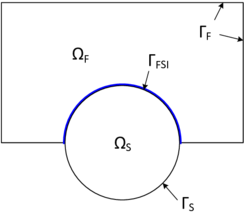Figure 18: FSI coupling interface - The fluid domain $\Omega _{F}$ with the boundary $\Gamma _{F}$ and the structure domain $\Omega _{S}$ with the boundary $\Gamma _{S}$ share the FSI interface $\Gamma _{FSI}$.

On the one hand, the particles are not allowed to cross the shared interface ${\textstyle \Gamma _{FSI}}$ which enforces a kinematic condition depending on the applied fluid model . In case the viscosity of the fluid cannot be neglected (viscous fluid), a "no-slip"-condition at the interface can be defined as follows

 ${\boldsymbol {u_{F}}}={\boldsymbol {u_{s}}}$
(3.1)

 ${\boldsymbol {v_{F}}}={\frac {\partial {\boldsymbol {u_{S}}}}{\partial t}}.$
(3.2)

These equations express the continuity of displacements and velocities across the interface. Physically it means that the fluid particles close to the interface conduct the same movement as the particles on the structure domain. Depending on which formulation and therefore which coupling variable is chosen (displacement or velocity), only one of the two equations is applied as they are equivalent in their physical meaning. If viscous effects of the fluid can be neglected, a "slip" condition needs to be defined instead. This results in the following relations

 ${\boldsymbol {n_{F}}}\cdot {\boldsymbol {u_{F}}}={\boldsymbol {n_{S}}}\cdot {\boldsymbol {u_{s}}}$
(3.3)

 ${\boldsymbol {n_{F}}}\cdot {\boldsymbol {v_{F}}}={\boldsymbol {n_{S}}}\cdot {\frac {\partial {\boldsymbol {u_{S}}}}{\partial t}}.$ (3.4)

which describes the continuity of displacements and velocities perpendicular to the interface.

On the other hand there are dynamic conditions which the fluid and structure have to comply to at the interface:

 ${\boldsymbol {n_{F}}}\cdot {\boldsymbol {\sigma _{F}}}={\boldsymbol {n_{S}}}\cdot {\boldsymbol {\sigma _{S}}}$
(3.5)

These conditions guarantee that the force equilibrium of the surface traction vectors along the interface is fulfilled.

## 3.2 Formulation of the FSI problem

A key question in approaching FSI problems is the question about how to formulate the material motion in the fluid and the structure field. In the last decades many different formulation methods have been proposed of which each has advantages and drawbacks when applied to certain physical problems. In the framework of this monograph we will focus on the fundamentally different ALE method and embedded method. However, in the first part of this chapter we want to relate these methods to a very general context of FSI formulation methods. Afterwards, the ALE approach will be further discussed in detail, whereas the embedded method was already treated intensively in chapter 2.2.4.

### 3.2.1 Two principal formulation methods

A very detailed and widespread overview about formulation methods in general may be found e.g. in ,  and  as well as in the included literature references.

To explain two principal formulation methods, let us first of all assume a rigid body motion ${\textstyle {\boldsymbol {u}}}$ of a structure within a discretized fluid domain (See figure 19).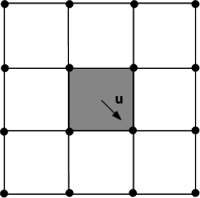Figure 19: Rigid body motion of a structure (grey) in a fluid domain

A classical way to handle the coupled motion is by using a body-fitted solution approach in which the fluid nodes at the FSI interface are forced to follow the movement of the structure at the same interface (See figure 20).

This is done in the so-called Arbitrary Lagrangian-Eulerian (ALE) method [5,19]. The ALE method has many advantages making it the method of choice for many applications. It allows for example an easy tracking of the FSI interface and therefore provides high accuracy of the flow along the interface. This may result in a high overall accuracy of the solution. Even cases in which the grids of the fluid and the structure along the interface do not exactly match can be handled. Therefore mapping techniques are used which in general allow to map quantities between arbitrary different grids. Throughout the present work we therefore use the Mortar Element Method described in .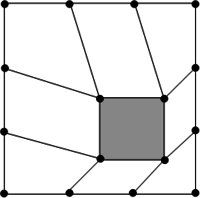Figure 20: Body-fitted solution approach

On the other side, however, it is possible in an ALE approach, that large deformations and rotations distort the mesh such that even a costly remeshing may become necessary. In fact this typically happens when simulating the previously introduced inflatable hangar. Here the problem becomes even more critical since the structure starts to wrinkle or fold as indicated in figure 21.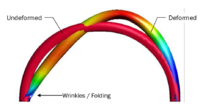Figure 21: Folded tube of an inflatable hangar structure - If the hangar is subjected to severe wind loads, the tubes may be massively deformed resulting in such a wrinkling and folding.

In order to treat large deformations or such complex movements, one can apply non-body-fitted or fixed-grid methods in which the fluid mesh remains unchanged. The fluid domain is then described by an Eulerian formulation and the structure moves independently from the fluid nodes locations. The concept is visually explained in figure 22.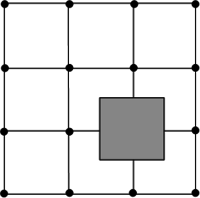Figure 22: Embedded approach - The fluid and the structure mesh are completely decoupled from each other.

A widely used method based on the fixed-grid approach is the embedded or immersed boundary method which was first proposed by Peskin [21,22] in order to simulate the blood flow through a beating heart. Initially used with Finite Differences, it was later extended to Finite Elements as the Immersed Finite Element Method, e.g. by using the discrete Dirac delta functions . Another derived method is the Fictitious Domain Method discussed e.g. by Glowinski et al.  which describes the interface between fluid and structure by means of a distributed Lagrange multiplier. An extension of the fixed-grid approach to the application of compressible solids and fluids is provided by the Immersed Continuum Method (see also ). A general overview on the derivations of Immersed Boundary Methods is e.g. given in . It is important to realize that with all immersed methods the solution accuracy or stability depends on the given background mesh which typically is seen as a big disadvantage.

Finally, the advantages of the ALE- and the embedded method can be combined in Chimera method which divides the fluid into a moving domain around the FSI interface and a non-moving domain further away from the interface. An example where the Chimera method was successfully applied with flexible structures is given in .

In the following the ALE method is discussed on a more theoretical basis. The theoretical background of the embedded method is given in chapter 2.2.4.

### 3.2.2 Arbitrary Lagrangian-Eulerian Method

The purely Lagrangian description of the fluid domain has the disadvantage to result in locally strong mesh distortions with increased motion of the FSI interface. Having this in mind, the goal of the ALE method is to let the fluid nodes move "arbitrarily" in a Eulerian manner such that the distortions of the elements in the fluid domain are minimized and larger structural deformations are possible. Therefore, the advantages of Lagrangian and Eulerian methods are combined (consider also the overview in table 1).

The ALE method was first applied to FEM by Donea, [3,5], whose papers are a recommendable guideline to understand the algorithm in detail. As opposed to the Lagrangian and Eulerian description of motion, the idea of the ALE method is to introduce a third domain, i.e. the mesh domain. The latter is the so-called referential configuration with the corresponding referential coordinates ${\textstyle \chi }$ describing the motion of the mesh points. The velocity of the grid points ${\textstyle {\boldsymbol {v_{m}}}}$ can be hence be computed as

 ${\boldsymbol {v_{m}}}={\boldsymbol {v_{m}}}({\boldsymbol {\chi }},t)=\left.{\frac {\partial {\boldsymbol {x}}}{\partial t}}\right|_{\boldsymbol {\chi }}.$
(3.6)

Based on the mesh velocity we can introduce a new measure which characterizes the relative velocity between the mesh and the material points, i.e. the convective velocity ${\textstyle {\boldsymbol {c}}}$:

 ${\boldsymbol {c}}={\boldsymbol {v}}-{\boldsymbol {v_{m}}}.$
(3.7)

Using the convective velocity we unify the Lagrangian and Eulerian description in an ALE formulation by reformulating the material derivative (see equation 2.4) such that it refers to the additionally introduced referential (mesh) configuration:

 $\left.{\frac {\partial f}{\partial t}}\right|_{\boldsymbol {X}}={\frac {Df}{Dt}}=\left.{\frac {\partial f}{\partial t}}\right|_{\boldsymbol {\chi }}+{\boldsymbol {c}}\cdot \nabla f,$
(3.8)

whereas ${\textstyle f}$ can be e.g. the fluid particle velocity ${\textstyle {\boldsymbol {v}}}$. Based on the adjusted material derivative, the momentum equation of the Navier-Stokes Equations (2.10.a) can be rewritten as

 $\left.{\frac {\partial {\boldsymbol {v}}}{\partial t}}\right|_{\boldsymbol {\chi }}+({\boldsymbol {c}}\cdot \nabla ){\boldsymbol {v}}-\nu \nabla ^{2}{\boldsymbol {v}}+\nabla p={\boldsymbol {b}},$
(3.9)

in which we replaced in the convective term the material velocity by the convective velocity. Equation 3.9 represents the ALE-formulation of the Navier-Stokes Equations.

Finally, the aforementioned "arbitrary" movement of the mesh points at each time step requires certain so-called mesh-updating strategies which distribute the interface deformation over the fluid domain. Many approaches have been proposed to this end, whereas two main strategies can be identified: mesh-regularization and mesh-adaption . Herein, we will concentrate on strategies based on mesh regularization. A very straight-forward approach in this context is to handle the movement of the FSI interface by solving a second-order partial differential equation. Prominent examples are the springs algorithm (see e.g. Farhat ), the elastic deformation method (e.g. Baker ) or solving a Laplacian equation based on the interface movement, which goes back to the work of Winslow . The specific algorithms we use throughout this work are described in chapter 8.2.1.

## 3.3 Solution of the FSI problem

In the previous chapter the formulation of the FSI problem was discussed. With that in mind this chapter now aims to provide the theoretical background in terms of the corresponding solution procedure. To this end we will in the first section discuss the two main solution approaches, i.e. the monolithic and the partitioned or staggered solution. Since we are in the course of this monograph only applying the partitioned approach, we will here mainly focus on the latter solution technique. A detailed description of how to actually reformulate the system to obtain a partitioned solution process is therefore presented. In the second part of this chapter then we will concentrate on one of the most important numerical problems related to the partitioned FSI analyses, i.e. the artificial added-mass effect. This will be important for the interpretation of the results later. Finally a stabilization technique shall be presented which allows to effectively use the partitioned analysis for strongly coupled problems.

### 3.3.1 From the monolithic to a partitioned solution

This section will introduce the theoretical concepts in terms of the numerical treatment of FSI-problems. In doing so we will mainly rely on the very detailed elaborations in , , , and , to which the interested reader shall be referred to for further information. Distinct focus will be set on techniques to establish a fully partitioned solution scheme based on a Dirichlet-Neumann coupling. Even though the formulation of the underlying mechanics as well as the corresponding concepts are introduced in a very general manner, no attempt will be made to cover the full spectrum of possible solution procedures. A very nice and profound classification of the latter, though, can be found in chapter ${\textstyle 6.1}$ of .

From a mathematical point of view an FSI problem can be expressed through an ODE system in the form1

 $\left[{\begin{array}{cc}{\boldsymbol {M}}_{SS}&{\boldsymbol {M}}_{SF}\\{\boldsymbol {M}}_{FS}&{\boldsymbol {M}}_{FF}\\\end{array}}\right]\left[{\begin{array}{cc}{\boldsymbol {a}}_{S}\\{\boldsymbol {a}}_{F}\end{array}}\right]+\left[{\begin{array}{cc}{\boldsymbol {C}}_{SS}&{\boldsymbol {C}}_{SF}\\{\boldsymbol {C}}_{FS}&{\boldsymbol {C}}_{FF}\\\end{array}}\right]\left[{\begin{array}{cc}{\boldsymbol {v}}_{S}\\{\boldsymbol {v}}_{F}\end{array}}\right]$ $+\left[{\begin{array}{cc}{\boldsymbol {K}}_{SS}&{\boldsymbol {K}}_{SF}\\{\boldsymbol {K}}_{FS}&{\boldsymbol {K}}_{FF}\\\end{array}}\right]\left[{\begin{array}{cc}{\boldsymbol {u}}_{S}\\{\boldsymbol {u}}_{F}\end{array}}\right]=\left[{\begin{array}{cc}{\boldsymbol {b}}_{S}\\{\boldsymbol {b}}_{F}\end{array}}\right]$
(3.10)

where ${\textstyle {\boldsymbol {u}}}$, ${\textstyle {\boldsymbol {v}}}$ and ${\textstyle {\boldsymbol {a}}}$ characterize the motion of either the fluid ${\textstyle F}$ or the structure ${\textstyle S}$, the coefficient matrix ${\textstyle {\boldsymbol {M}}}$ describes the mass, ${\textstyle {\boldsymbol {C}}}$ the damping and ${\textstyle {\boldsymbol {K}}}$ the stiffness of the system. Furthermore ${\textstyle {\boldsymbol {b}}}$ denotes the right-hand side that contains the sum of all imposed forces. The actual coupling between both domains is herein given by the off-diagonal or mixed terms indexed with ${\textstyle FS}$ or ${\textstyle SF}$.

If all the matrices are now fully occupied one speaks of a fully coupled or two-way coupled FSI-problem. Here we may distinguish between two different situations: strongly coupled systems in which the density ratio between fluid and structure is ${\textstyle {\frac {\rho _{F}}{\rho _{S}}}\approx 1}$, and weakly coupled systems where the respective density ratio is ${\textstyle {\frac {\rho _{F}}{\rho _{S}}}<<1}$. This distinction is important with regard to the choice of the solution procedure.

If in either of the two lines of the above system the mixed terms are missing a one-way coupled system is obtained and finally if no mixed-terms are present the two systems are fully decoupled and could be solved independently.

Given a fully coupled FSI problem and assuming that all the corresponding coefficients in 3.10 are known, the ODE system can be directly discretized in time leading to the so called “monolithic” formulation of the coupled problem. In that configuration the complete problem can be solved for the state variables conveniently in a single solution step by means of an arbitrary integrator for ODE-systems, which is very advantageous from the point of view of accuracy. This solution procedure is in the following referred to as the monolithic solution.

The disadvantage of a monolithic solution procedure is, however, that the corresponding system of equations is generally very large (all the variables of the problem need to be solved at the same time) and often badly conditioned due to the coexistence of terms coming from the description of physically different problems . To overcome these disadvantages it is possible to split the monolithic system into the two single field problems such that they indeed take into account contributions from each the other field at the common boundary or interface, respectively, but apart from that can be solved independently. As we will see later, the solution then follows a defined strategy which is based on a mutual exchange and imposition of boundary conditions at the common interface.

The underlying formulation of such a partitioned solution procedure is obtained by bringing all the coupling quantities in equation 3.10 to the right hand side. The system then reads:

 $\left[{\begin{array}{cc}{\boldsymbol {M}}_{SS}&0\\0&{\boldsymbol {M}}_{FF}\\\end{array}}\right]\left[{\begin{array}{cc}{\boldsymbol {a}}_{S}\\{\boldsymbol {a}}_{F}\end{array}}\right]+\left[{\begin{array}{cc}{\boldsymbol {C}}_{SS}&0\\0&{\boldsymbol {C}}_{FF}\\\end{array}}\right]\left[{\begin{array}{cc}{\boldsymbol {v}}_{S}\\{\boldsymbol {v}}_{F}\end{array}}\right]$ $+\left[{\begin{array}{cc}{\boldsymbol {K}}_{SS}&0\\0&{\boldsymbol {K}}_{FF}\\\end{array}}\right]\left[{\begin{array}{cc}{\boldsymbol {u}}_{S}\\{\boldsymbol {u}}_{F}\end{array}}\right]=\left[{\begin{array}{cc}{\boldsymbol {RS}}_{S}\\{\boldsymbol {RS}}_{F}\end{array}}\right]$
(3.11)

in which

 ${\begin{array}{cc}{\boldsymbol {RS}}_{S}={\boldsymbol {b}}_{S}-{\boldsymbol {M}}_{SF}{\boldsymbol {a}}_{F}-{\boldsymbol {C}}_{SF}{\boldsymbol {v}}_{F}-{\boldsymbol {K}}_{SF}{\boldsymbol {u}}_{F}\\{\boldsymbol {RS}}_{F}={\boldsymbol {b}}_{F}-{\boldsymbol {M}}_{FS}{\boldsymbol {a}}_{S}-{\boldsymbol {C}}_{FS}{\boldsymbol {v}}_{S}-{\boldsymbol {K}}_{FS}{\boldsymbol {u}}_{S}\end{array}}$
(3.12)

This system of equations may be eventually solved in a partitioned manner.

Besides the fact that we are avoiding the complications related to a monolithic solution, this partitioned solution procedure offers a few more very nice advantages. The most important one is the fact that from a solution perspective the two different mechanical problems are decoupled. That is optimal from the point of view of software modularity since for either problem we can use dedicated and well-established high-performance solvers. The disadvantage, though, is obviously the additional computational effort. Since we are solving each system independently, we need make sure that in each time step the coupling conditions are fulfilled to a high degree of accuracy in order to keep the accumulated error minimal. This requires an additional solution iteration in each time step and hence causes a significant increase in the number of solver calls. Another typical disadvantage arises from possible convergence problems during the additional solution iteration. Also it may happen that the problem is not well defined on one of the domains since boundary conditions are only defined in the other domain2. In a lot of applications, though, the advantages of this approach outweigh the disadvantages making it a frequently applied solution approach in the context of FSI simulations.

Nevertheless, the feasibility of such an approach relies on the following three assumptions :

1. the system (equation 3.10) is linear
2. the corresponding matrix coefficients are all known
3. it is possible to define physically stable test case

For a detailed discussion of the latter assumptions the interested reader is referred to chapter ${\textstyle 4.2}$ page ${\textstyle 81}$ in . At this point it is only important to note, that in case of the interaction between flexible structures and incompressible fluids, which is the system of interest within this monograph, none of the previous assumptions are valid. This leads particularly in situations where ${\textstyle {\frac {\rho _{F}}{\rho _{S}}}\approx 1}$, i.e. in strongly coupled problems, to numerical difficulties. One such difficulty is the artificial added-mass effect described in the follow-up section.

To overcome these problems two principal solution or coupling strategies may be applied in partitioned analyses3:

1. an explicit computation with prediction-correction techniques
2. an iterative or implicit solution procedure

In the first case the single field problems are solved subsequently just once in each time step. Therefore typically information from the previous time step is used as a prediction. In order to reduce the hence occurring spurious energy, additional correction techniques may be applied. Given a very weak coupling, i.e. ${\textstyle {\frac {\rho _{F}}{\rho _{S}}}<<1}$ , the solution of the system formulated in equation 3.10 can be simplified even more since then it can be solved in a purely explicit manner without correction. A purely explicit computation is the fastest way to obtain a coupled solution in the context of a partitioned FSI analysis. In practical applications, however, it is typically either not sufficient or not applicable. Since in the scope of this monograph we are exclusively relying on the second of the above principal coupling strategies, details are omitted here. Instead the simple but complete example from chapter 2.1.1 in  shall be recommended. It describes the algorithmic idea of the partitioned analysis4 by means of an explicit computation with prediction-correction techniques which may serve as a basis for further research in this context.

In case of the second solution strategy, iterative schemes are applied to control numerical problems in a coupled analysis. The basic idea in this context is an implicit treatment of the coupling variables where in each time step the latter are iteratively improved until the residuum, defined by the the coupling conditions, is below a certain tolerance threshold. Then one proceeds in time starting with the iteration all over again. An illustration of the implicit solution procedure is given in figure 23.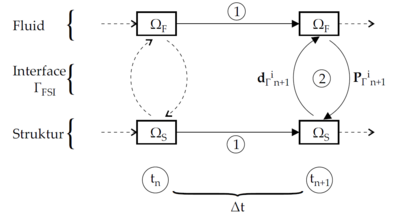] Figure 23: Iterative solution procedure - Adopted from 

Important to note is that by using such a solution approach, the coupling conditions are fulfilled (up to the degree of accuracy defined by the tolerance threshold) and the energy conservation at the common interface is ensured. An implicit solution of equation 3.11 following the procedure above hence principally convergences to the monolithic solution of equation 3.10 (Given that it converges at all) , which has to be an essential characteristic of a partitioned approach in general. In order to actually allow for convergence, though, we have to follow one of several possible iteration schemes. Throughout the present monograph the so called “Gauss-Seidel” fixed-point iteration scheme was chosen to this end. The reason for this choice was the existing profound experience basis in this regards.

The Gauss-Seidel fixed-point iteration aims to iteratively solve the constrained nonlinear FSI problem from equation 3.11 assuming that the sought equilibrium condition appears as a so called fixed-point5 in the solution space. The corresponding iteration rule then requires to reformulate 3.11 such that we obtain its corresponding fixed-point form:

 $0={\boldsymbol {M}}_{S}{\boldsymbol {a}}_{S}^{k+1}+{\boldsymbol {C}}_{S}{\boldsymbol {v}}_{S}^{k+1}+{\boldsymbol {K}}_{S}{\boldsymbol {u}}_{S}^{k+1}-f({\boldsymbol {a}}_{F}^{k},{\boldsymbol {v}}_{F}^{k},{\boldsymbol {u}}_{F}^{k})$
(3.13.a)

 $0={\boldsymbol {M}}_{F}{\boldsymbol {a}}_{F}^{k+1}+{\boldsymbol {C}}_{F}{\boldsymbol {v}}_{F}^{k+1}+{\boldsymbol {K}}_{F}{\boldsymbol {u}}_{F}^{k+1}-f({\boldsymbol {a}}_{S}^{k+1},{\boldsymbol {v}}_{S}^{k+1},{\boldsymbol {u}}_{S}^{k+1})$
(3.13.b)

where ${\textstyle k}$ is the iteration index. Now we recap that at the interface it holds:

 ${\begin{array}{cc}{\boldsymbol {u}}_{S,\Gamma }={\boldsymbol {u}}_{F,\Gamma }\\{\boldsymbol {v}}_{S,\Gamma }={\boldsymbol {v}}_{F,\Gamma }\\{\boldsymbol {a}}_{S,\Gamma }={\boldsymbol {a}}_{F,\Gamma }\end{array}}\quad \forall t$
(3.14)

Using 3.13 and 3.14 the iteration rule of the Gauss-Seidel fixed-point iteration finally reads

1. Given ${\textstyle {\boldsymbol {u}}_{F}^{k},{\boldsymbol {v}}_{F}^{k},{\boldsymbol {a}}_{F}^{k}}$ from the previous time step or iteration, solve the structure problem in 3.13.a for ${\textstyle {\boldsymbol {u}}_{S}^{k+1},{\boldsymbol {v}}_{S}^{k+1},{\boldsymbol {a}}_{S}^{k+1}}$
2. Use the coupling conditions in 3.14 to prescribe the solution quantities at the interface on fluid side.
3. Solve the fluid problem in equation 3.13.b for the remaining solution quantities ${\textstyle {\boldsymbol {u}}_{F}^{k+1},{\boldsymbol {v}}_{F}^{k+1},{\boldsymbol {a}}_{F}^{k+1}}$ on fluid side. Then restart iteration.

According to Banach's fixed-point theorem this iteration now converges under the condition that each single iteration step mathematically corresponds to a contraction. The latter condition, though, is not always fulfilled and typically violated in strongly coupled problems, which we actually wanted to solve with the introduced iterative scheme. To make sure that the contraction condition remains valid along the iteration, however, we can use a relaxation method which relaxes either of the coupling variables at the interface during step 2) in the above iteration rule. The corresponding relaxation generally reads:

 ${\tilde {\boldsymbol {V}}}_{\Gamma }^{k+1}={\boldsymbol {V}}_{\Gamma }^{k}+\omega \left({\boldsymbol {V}}_{\Gamma }^{k+1}-{\boldsymbol {V}}_{\Gamma }^{k}\right)$
(3.15)

 ${\tilde {\boldsymbol {V}}}_{\Gamma }^{k+1}=\omega {\boldsymbol {V}}_{\Gamma }^{k+1}+\left(1-\omega \right){\boldsymbol {V}}_{\Gamma }^{k}$
(3.16)

where ${\textstyle {\tilde {\boldsymbol {V}}}_{\Gamma }}$ represents the relaxed coupling variable, ${\textstyle {\boldsymbol {V}}_{\Gamma }}$ the unrelaxed variable, ${\textstyle k}$ denotes the iteration index and ${\textstyle \omega }$ the relaxation factor. In the specific case where the structural velocity is relaxed, as we will do it later, step 2) in the above iteration reads:

 ${\tilde {\boldsymbol {v}}}_{S,\Gamma }^{k+1}=\omega {\boldsymbol {v}}_{S,\Gamma }^{k+1}+\left(1-\omega \right){\boldsymbol {v}}_{S,\Gamma }^{k}$
(3.17)

This means, that we are in each iteration step not imposing the actual simulated value for the structural velocity on the fluid boundary at the common interface, but only an, according to ${\textstyle \omega }$, relaxed value, which is typically smaller than the original one. That is the relaxation factor is typically chosen ${\textstyle \omega <1}$ which corresponds to an underrelaxation. Practically interpreted this means that we are relaxing the load with which the structure excites the fluid in each iteration during one time step. Conceptually we are by that ensuring a contraction in the fixed-point iteration. As a result convergence of the fixed-point iteration may be achieved even with strongly coupled problems. The fixed-point iteration scheme combined with the above described relaxation method hence poses a powerful solution procedure that also allows a partitioned analysis of strongly coupled problems.

Even though this is a very powerful approach, the question remains, how to choose ${\textstyle \omega }$. Generally it holds that the corresponding value needs to be as low as possible to actually achieve numerical stability but high enough to reduce the hence increasing number of necessary iteration steps as much as possible. In practice this trade-off often cannot be effectively balanced out by the program user himself. Instead automatized strategies to accelerate convergence can be applied. A very effective and important strategy in this context is the adaptive relaxation using the Aitken-method which during the iteration continuously computes new and improved relaxation parameters. A detailed elaboration of this method including its computation as well as background information can be found in , chapter ${\textstyle 6.6.2.2}$ and the herein given references. Throughout this monograph the above discussed Gauss-Seidel coupling strategy is combined with the aforementioned Aitken-method in order to ensure convergence.

With the Aitken-method now we discussed all the principal theoretical concepts regarding the solution of a coupled fluid-structure problem that will be of importance in the later course of this monograph. As already stated in the beginning we in particular focused here on a partitioned solution procedure. What in this regard was already mentioned but not elaborated further so far is the fact that a partitioned approach typically faces well-known numerical problems, which has to be taken into account in a proper simulation. One of the most important problems shall be discussed in the following section.

(1) Here we already discretized in space via the finite element method

(2) Pressure boundary conditions on the structure are actually defined in the fluid domain

(3) A profound overview of different coupling strategies, in particular for the second case, is given in , chapter $6.6$

(4) In  denoted as the “staggered approach”

(5) See respective mathematical textbooks for a definition

Whenever acceleration is imposed on a fluid flow, either by accelerating an embedded body or by an external acceleration of the fluid, additional inertia forces will act on surfaces of embedded bodies that are in contact with the fluid . This effect is typically referred to as the “artificial added-mass effect”. For a simple voluminous, spherical particle embedded in an incompressible fluid domain for instance the additional inertia force, also referred to as the virtual mass force, is given as :

 ${\boldsymbol {F}}_{VM}={\frac {\rho _{F}V_{S}}{2}}\left({\frac {D{\boldsymbol {v}}_{F}}{Dt}}-{\frac {d{\boldsymbol {v}}_{S}}{dt}}\right)$
(3.18)

where ${\textstyle {\boldsymbol {v}}_{F}}$ is the fluid flow velocity, ${\textstyle {\boldsymbol {v}}_{S}}$ is the spherical particle velocity, ${\textstyle \rho _{F}}$ is the mass density of the fluid, ${\textstyle V_{S}}$ is the volume of the particle, and ${\textstyle {\frac {D}{Dt}}}$ denotes the material time derivative. Taking into account the aforementioned virtual mass force, the momentum equation of the particle for example reads:

 $m_{S}{\frac {d{\boldsymbol {v}}_{S}}{dt}}=\Sigma {\boldsymbol {F}}+{\boldsymbol {F}}_{VM}=\Sigma {\boldsymbol {F}}+{\frac {\rho _{F}V_{S}}{2}}\left({\frac {D{\boldsymbol {v}}_{F}}{Dt}}-{\frac {d{\boldsymbol {v}}_{S}}{dt}}\right)$
(3.19)

in which ${\textstyle m_{S}}$ represents the mass of the particle and ${\textstyle \Sigma {\boldsymbol {F}}}$ contains all the imposed forces like the gravitational force, drag, lift, Basset force, etc.. By rearranging the equation we get:

 $\left(m_{S}+{\frac {\rho _{F}V_{S}}{2}}\right){\frac {d{\boldsymbol {v}}_{S}}{dt}}=\Sigma {\boldsymbol {F}}+{\frac {\rho _{F}V_{S}}{2}}{\frac {D{\boldsymbol {v}}_{F}}{Dt}}$
(3.20)

In front of the first order time derivative of the particle velocity now an extra mass term occurs that arises due to the interaction of the fluid with the particle or the structure in general. That is, the particle accelerates as if it had an extra mass of half the fluid it displaces. This extra term is typically referred to as “artificial added-mass” or simply “added-mass” giving this effect its notion. The particle is now just an example, but the effect is conceptually the same in the general case. A mathematically more rigorous derivation for the general case is given in .

As already stated in the beginning, the artificial added-mass effect affects the surface of the structure that is in contact with the fluid. Transferred to the case of a partitioned FSI analysis the effect hence exclusively occurs at the coupling interface. Furthermore it is important to realize that, since we are in an FSI analysis generally dealing with transient movements, the artificial added-mass effect is always present. Its impact depends, however, on the density of fluid. If the fluid density is small compared to the density of the structure it can typically be neglected. In cases where the density of the fluid is about the density of the structure or higher, the added-mass may be even greater than the mass of the structure itself so the corresponding effect has to be taken into account. Severe numerical errors might be the consequence else. A typical error that is seen in this context, is for instance a pressure distribution that is significantly oscillating in time.

The effect is particularly a problem in fluid-structure scenarios with incompressible fluids at a density ratio of ${\textstyle {\frac {\rho _{F}}{\rho _{S}}}\approx 1}$. Here the resulting inertia terms may dominate the solution of the interaction problem. On the other hand side the effect generally diminishes in an analysis with compressible fluids. This can be understood with a rather practical interpretation of the artificial added-mass effect: Since fluid and structure cannot occupy the same space at the same time the structure displaces the fluid as it accelerates through it. The fluid reacts to it with an additional inertia response, that in turn is all the more distinct the higher the effective mass of the fluid, i.e. the one partition of the entire fluid mass that immediately reacts to this state change. In compressible fluids obviously the effective mass is significantly lower than in incompressible fluids where, due to the incompressibility constraint, the entire mass of the fluid reacts to this state change at once.

In partitioned FSI analyses the artificial added-mass effect and the possible numerical errors are typically handled very effectively by means of implicit coupling strategies. As we will see later, however, this might be still not enough in terms of the herein discussed embedded method. In this case additional techniques are necessary in order to prevent numerical instabilities. A corresponding technique that was applied throughout this monograph will be introduced in the following section.

### 3.3.3 Dealing with the artificial added-mass effect in incompressible flows

In the previous chapter we described a particular problematic phenomena when computing an FSI scenario with incompressible fluids in a partitioned approach, i.e. the artificial added-mass effect. One typically very efficient way to avoid this phenomena is to use an implicit, iterative solution procedure with corresponding relaxation technique as described in the first part of this chapter. Despite the application of the latter, however, it could be observed with the herein given examples, that in particular the embedded method still is prone for instabilities due to this artificial added-mass effect, given that we have a density ratio close to one. So in order to prepare the embedded method also for problems of the latter kind it was necessary to improve the stability of the partitioned embedded solution procedure.

In order to understand where there is actually potential for an improvement we recap the origin of the problem with the artificial added-mass effect from a more pragmatic perspective: The problem is, that we do not incorporate any sensitivity information so far into the analysis of the fluid-structure interaction since the computation of the corresponding derivatives is very expensive. That is whenever we compute the solution of the fluid as a reaction to the movement of the structure we do actually not take into account that the hence resulting pressure variation has an influence on the movement again. In turn, to be more precise we would have to incorporate the sensitivity of the structural movement w.r.t. to a pressure variation into the analysis of the actual pressure variation on CFD side. By neglecting this, it is essentially assumed that the structure and the fluid may partially overlap during the iteration from one time step to the other. This is of course contrary to the original assumption of incompressibility which eventually leads to pressure-oscillations or this artificial added-mass, respectively. So the general idea is now to incorporate into the analysis of the fluid this missing information about the structural response if there is a pressure variation at the interface (This approach partially follows the ideas presented in ). To incorporate the missing information, we have to perform two basic tasks:

1. Find a sufficient prediction of the structural response and
2. incorporate this prediction into the fluid analysis.

Let us first concentrate on the linearized prediction of the structural movement. In order to keep it simple here we will in the following derive the necessary equations on a discrete level which is based on a standard Galerkin weak form analysis. With that in mind, consider the case of a general structural problem in the form

 ${\boldsymbol {M}}{\frac {\partial {\boldsymbol {v}}}{\partial t}}+{\boldsymbol {K}}{\boldsymbol {u}}={\boldsymbol {f}}$
(3.21)

where we used the same notation as in the previous chapters and comprised all the external forces in one vector ${\textstyle {\boldsymbol {f}}}$. Introducing a first order backward-Euler time-integration this can be further discretized in time as

 ${\boldsymbol {M}}{\frac {{\boldsymbol {v}}_{n+1}-{\boldsymbol {v}}_{n}}{\Delta t}}+{\boldsymbol {K}}{\boldsymbol {u}}={\boldsymbol {f}}$
(3.22)

where ${\textstyle n}$ and ${\textstyle n+1}$ denote the current and following time step, respectively. Having introduced the time discretization we may reformulate the problem such that we have an expression which approximates the structural movement as

 ${\boldsymbol {v}}_{n+1}={\boldsymbol {v}}_{n}+\Delta t{\boldsymbol {M}}^{-1}{\boldsymbol {f}}-\Delta t{\boldsymbol {M}}^{-1}{\boldsymbol {K}}{\boldsymbol {u}}$
(3.23)

Now we note that the displacement ${\textstyle {\boldsymbol {u}}}$ is proportional to the time step ${\textstyle \Delta t}$, i.e. ${\textstyle {\boldsymbol {u}}\propto \Delta t}$. That is as the time step reduces the second term with the tangential stiffness in the equation above decreases second order while the first term depending on ${\textstyle f}$ reduces linearly. Taking now into account that this stabilization is to be applied in strongly coupled problems where typically very small time steps are required, we may neglect the influence by the tangential stiffness as since the structural behavior is mainly dominated by the inertia term. Equation 3.23 reduces to

 ${\boldsymbol {v}}_{n+1}={\boldsymbol {v}}_{n}+\Delta t{\boldsymbol {M}}^{-1}{\boldsymbol {f}}$
(3.24)

Note that this is a clear simplification but as we will see later it is sufficient for a proper stabilization. Introducing now an iterative solution technique with iteration index ${\textstyle k}$ the solution for this equation at two subsequent iteration steps reads1

 ${\boldsymbol {v}}_{n+1}^{k}={\boldsymbol {v}}_{n}+\Delta t{\boldsymbol {M}}^{-1}{\boldsymbol {f}}^{k}$
(3.25.a)

 ${\boldsymbol {v}}_{n+1}^{k+1}={\boldsymbol {v}}_{n}+\Delta t{\boldsymbol {M}}^{-1}{\boldsymbol {f}}^{k+1}$
(3.25.b)

Subtracting now equation 3.25.a from 3.25.b and rearranging the resulting term we get a linearized Newton-Raphson solution scheme to solve for the structural velocities depending on the external forces:

 ${\boldsymbol {v}}_{n+1}^{k+1}={\boldsymbol {v}}_{n+1}^{k}+\Delta t{\boldsymbol {M}}^{-1}\left({\boldsymbol {f}}^{k+1}-{\boldsymbol {f}}^{k}\right)$
(3.26)

Now we note that, by choosing a weak formulation and the Galerkin discretization, the external force vector is decomposed into body forces and surface tractions along the Neumann boundary:

 ${\boldsymbol {f}}=\int _{\Omega }{\boldsymbol {N}}^{T}\rho {\boldsymbol {b}}~d\Omega +\int _{\Gamma }{\boldsymbol {N}}^{T}{\hat {T}}~d\Gamma$
(3.27)

where ${\textstyle {\boldsymbol {b}}}$ is the body force and ${\textstyle {\hat {T}}}$ the surface traction that may be given as a surrounding pressure field in the form

 ${\hat {T}}=p{\boldsymbol {n}}$
(3.28)

with ${\textstyle {\boldsymbol {n}}}$ being the surface normal at the coupling boundary. Introducing 3.28 in 3.27 and the latter into 3.26, the final structural movement may be written as

 ${\boldsymbol {v}}_{n+1}^{k+1}={\boldsymbol {v}}_{n+1}^{k}+\Delta t{\boldsymbol {M}}^{-1}\left[\int _{\Omega }{\boldsymbol {N}}^{T}\rho \left({\boldsymbol {b}}^{k+1}-{\boldsymbol {b}}^{k}\right)~d\Omega +\int _{\Gamma }{\boldsymbol {N}}^{T}\left(p^{k+1}-p^{k}\right){\boldsymbol {n}}~d\Gamma \right]$
(3.29)

Note that by these operations we obtained an expression that not just describes the reaction of the structure to any body load, but also an expression that takes into account a pressure variation ${\textstyle p^{k+1}-p^{k}}$ along the Neumann boundary. Practically interpreted this means that, given a pressure variation from any environmental phenomena, such as a surrounding fluid, its influence on the structural movement may be described by the boundary term

 $\Delta t{\boldsymbol {M}}^{-1}\int _{\Gamma }{\boldsymbol {N}}^{T}\left(p^{k+1}-p^{k}\right){\boldsymbol {n}}~d\Gamma$
(3.30)

The information from the above boundary terms can now be used to improve the fractional step solution procedure when computing the fluid in a partitioned FSI problem. Before entering the term, however, in the fractional step process, we want to prepare it some more.

First we note, that the pressures in an FSI context are nodal quantities which is why we also need to discretize them by means of finite elements. Taking the latter into account we can reformulate equation 3.30 to

 $\Delta t{\boldsymbol {M}}^{-1}\int _{\Gamma }{\boldsymbol {N}}{\boldsymbol {N}}^{T}{\boldsymbol {n}}~d\Gamma \left(p^{k+1}-p^{k}\right)$
(3.31)

where the pressure values ${\textstyle p}$ are now nodal quantities interpolated via the additional shape function ${\textstyle N}$. The integral can now be computed by means of Gauss-integration techniques. Here all the constant terms were put outside the integral.

Second we note that in the FSI context the boundary along which the pressure variation needs to be taken into account is the common interface or “wet” interface ${\textstyle \Gamma _{\hbox{FSI}}}$, respectively. We may thus want to make this clear by denoting the boundary term as

 $\Delta t{\boldsymbol {M}}^{-1}\int _{\Gamma _{\hbox{FSI}}}{\boldsymbol {N}}{\boldsymbol {N}}^{T}{\boldsymbol {n}}~d\Gamma _{\hbox{FSI}}\left(p^{k+1}-p^{k}\right)$
(3.32)

Also we want to emphasize for the later application in the FSI that the above mass matrix ${\textstyle {\boldsymbol {M}}}$ describes the nodal masses at the boundary of the structure by replacing ${\textstyle {\boldsymbol {M}}}$ with ${\textstyle vec{M}_{S}}$:

 $\Delta t{\boldsymbol {M}}_{S}^{-1}\int _{\Gamma _{\hbox{FSI}}}{\boldsymbol {N}}{\boldsymbol {N}}^{T}{\boldsymbol {n}}~d\Gamma _{\hbox{FSI}}\left(p^{k+1}-p^{k}\right)$
(3.33)

Third we note that the mass matrix ${\textstyle {\boldsymbol {M}}_{S}}$ is composed of the structural density ${\textstyle \rho _{S}}$ and the nodal volumes ${\textstyle V_{i}}$ assigned to each node on the boundary. By contrast the integral ${\textstyle \int _{\Gamma _{\hbox{FSI}}}{\boldsymbol {N}}{\boldsymbol {N}}^{T}{\boldsymbol {n}}~d\Gamma _{\hbox{FSI}}}$ poses a nodal area ${\textstyle A_{i}}$ which represents the area of influence of each node along the boundary. Assuming a nodal integration rule, both are hence of the form

 ${\boldsymbol {M}}_{S}^{-1}={\begin{pmatrix}{\frac {1}{\rho _{S}V_{i}}}&0&\cdots &0\\0&{\frac {1}{\rho _{S}V_{i}}}&\cdots &0\\\vdots &\vdots &\ddots &\vdots \\0&0&\cdots &{\frac {1}{\rho _{S}V_{i}}}\end{pmatrix}}$
(3.34)

 $\int _{\Gamma _{\hbox{FSI}}}{\boldsymbol {N}}{\boldsymbol {N}}^{T}{\boldsymbol {n}}~d\Gamma _{\hbox{FSI}}={\begin{pmatrix}A_{i}{\boldsymbol {n}}_{i}&0&\cdots &0\\0&A_{i}{\boldsymbol {n}}_{i}&\cdots &0\\\vdots &\vdots &\ddots &\vdots \\0&0&\cdots &A_{i}{\boldsymbol {n}}_{i}\end{pmatrix}}$ (3.35)

Considering now that the nodal volume of an interface node divided by the corresponding nodal area results in some nodal thickness description, i.e. ${\textstyle V_{i}/A_{i}=h_{i}}$, what remains from the multiplication of both matrices in equation 3.33 is a coefficient matrix ${\textstyle {\boldsymbol {C}}_{S}}$ in the form

 ${\boldsymbol {C}}_{S}(\rho _{S}\cdot h_{i})={\begin{pmatrix}{\frac {{\boldsymbol {n}}_{i}}{\rho _{S}h_{i}}}&0&\cdots &0\\0&{\frac {{\boldsymbol {n}}_{i}}{\rho _{S}h_{i}}}&\cdots &0\\\vdots &\vdots &\ddots &\vdots \\0&0&\cdots &{\frac {{\boldsymbol {n}}_{i}}{\rho _{S}h_{i}}}\end{pmatrix}}$
(3.36)

We can hence rewrite 3.33 such that we have

 $\Delta t{\boldsymbol {C}}_{S}(\rho _{S}\cdot h_{i})\left(p^{k+1}-p^{k}\right)$
(3.37)

A few important observations can now be made here. For example as ${\textstyle \rho _{S}}$ increases, this term tends more and more to zero, which when applied in the FSI context is what we expect since for heavy structures its movement will be less influenced by a surrounding fluid. That is, this term has a negligible influence. In turn, however, this means that given a lightweight structure, such as a membrane, neglecting equation 3.37 in the coupled analysis clearly introduces an error that may even cause the simulation to fail.

Another important observation relates to the role of the term ${\textstyle \rho _{S}\cdot h_{i}}$. The problem here is that, we indeed want to use this expression for a prediction of the structural movement in the CFD analysis, we, however, typically do not know this nodal thickness. In fact we only now it if the entire structure is a membrane since then the thickness is a prescribed parameter. That means given a voluminous structure we have to guess ${\textstyle h_{i}}$ at each node.

By being forced to guess ${\textstyle h_{i}}$ we introduced basically parameters that may be arbitrarily chosen later on in the CFD analysis. But since we do not want to guess a parameter for each node separately we may want to introduce a common parameter ${\textstyle h}$ for this “interface thickness or height, respectively”. Equation 3.37 hence reads

 $\Delta t{\boldsymbol {C}}_{S}(\rho _{S}\cdot h)\left(p^{k+1}-p^{k}\right)$
(3.38)

This parametrization is possible since when the variation of the pressure is very small and eventually zero the entire boundary term vanishes. Transferred to the FSI application this means that at convergence of the coupling conditions, where there is no iterative change of the pressure anymore, the above expression will disappear independent of the guess of ${\textstyle h}$. As a matter of fact this is the most important property of this approach since it guarantees that if the parameter is well chosen, we obtain a very nice convergence behavior because we took into account the movement of the structure, and if not, we are at least not distorting the overall solution.

So finally what we have is, given a pressure variation ${\textstyle p^{k+1}-p^{k}}$, the corresponding response of the structure may be estimated by means of guessing the parameter ${\textstyle h}$ and hence evaluate equation 3.38 which as such can be integrated into the fractional step solution process.

Having now found a good formulation for the prediction of the structural movement we may proceed to actually incorporate this term into the solution procedure of the fluid. To this end we recap that, assuming the fractional step technique that was applied throughout this monograph, we can express the unknown velocities in time step ${\textstyle n+1}$ by means of the estimated velocity ${\textstyle {\tilde {\boldsymbol {v}}}}$ as well as the unknown pressure values ${\textstyle p_{n+1}}$ and ${\textstyle p_{n}}$ in the form2

 ${\boldsymbol {v}}_{n+1}={\tilde {\boldsymbol {v}}}_{n+1}+\Delta t{\boldsymbol {M}}^{-1}{\boldsymbol {G}}(p_{n+1}-p_{n})$
(3.39)

As obvious there is not yet any structural information contained in this formulation. So the velocity in the next time step with a standard fractional step procedure applied to an FSI problem will be only computed by means of the given pressure delta without taking into account that the later also influences the movement of the structure and hence again ${\textstyle {\boldsymbol {v}}_{n+1}}$. To face this problem and to improve the coupling conditions the idea is now, whenever the fluid computes nodal quantities in the interior of the fluid domain, the standard fractional step procedure is applied, i.e. equation 3.39 is computed, but as soon as the nodal quantity is part of the common interface ${\textstyle \Gamma _{\hbox{FSI}}}$ we extend the given formulation by the above prediction from equation 3.38. The complete formulation hence reads:

 ${\begin{array}{ll}\quad {\hbox{in}}~\Omega {\hbox{:}}&{\boldsymbol {v}}_{n+1}={\tilde {\boldsymbol {v}}}_{n+1}+\Delta t{\boldsymbol {M}}^{-1}{\boldsymbol {G}}(p_{n+1}-p_{n})\\\\\quad {\hbox{ on}}~\Gamma _{\hbox{FSI}}{\hbox{ :}}&{\boldsymbol {v}}_{S,n+1}={\tilde {\boldsymbol {v}}}_{S,n+1}+\Delta t{\boldsymbol {M}}^{-1}{\boldsymbol {G}}(p_{n+1}-p_{n})+\Delta t{\boldsymbol {C}}_{S}(\rho _{S}\cdot h)\left(p_{n+1}-p_{n}\right)\end{array}}$
(3.40)

In order to avoid clutter we will drop here any indices from the underlying Newton-Raphson scheme and instead just use the time indices to indicate which quantity is given at which time instance. Furthermore we will abbreviate the given pressure deltas by ${\textstyle p_{n+1}-p_{n}=\Delta p}$ Keeping that in mind, we can take the divergence of the entire expression such that we get

 ${\begin{array}{ll}\quad {\hbox{in}}~\Omega {\hbox{:}}&0={\boldsymbol {D}}{\tilde {\boldsymbol {v}}}_{n+1}+\Delta t{\boldsymbol {D}}{\boldsymbol {M}}^{-1}{\boldsymbol {G}}\Delta p\\\\\quad {\hbox{ on}}~\Gamma _{\hbox{FSI}}{\hbox{ :}}&0={\boldsymbol {D}}{\tilde {\boldsymbol {v}}}_{S,n+1}+\Delta t{\boldsymbol {D}}{\boldsymbol {M}}^{-1}{\boldsymbol {G}}\Delta p+\Delta t{\boldsymbol {D}}{\boldsymbol {C}}_{S}(\rho _{S}\cdot h)\Delta p\end{array}}$
(3.41)

Note that we enforce incompressibility in each time step which is why ${\textstyle {\boldsymbol {D}}{\boldsymbol {v}}_{n+1}}$ has to be zero. A reformulation such that we have the auxilliary velocity on the left-hand side and factorizing out the pressure delta yields

 ${\begin{array}{ll}\quad {\hbox{in}}~\Omega {\hbox{:}}&{\boldsymbol {D}}{\tilde {\boldsymbol {v}}}_{n+1}=\Delta t{\boldsymbol {D}}{\boldsymbol {M}}^{-1}{\boldsymbol {G}}\Delta p\\\\\quad {\hbox{ on}}~\Gamma _{\hbox{FSI}}{\hbox{ :}}&{\boldsymbol {D}}{\tilde {\boldsymbol {v}}}_{S,n+1}=\Delta t\left[{\boldsymbol {D}}{\boldsymbol {M}}^{-1}{\boldsymbol {G}}+{\boldsymbol {D}}{\boldsymbol {C}}_{S}(\rho _{S}\cdot h)\right]\Delta p\end{array}}$
(3.42)

This now exactly represents the second step in the fractional step algorithm in which we are computing for the unknown pressure value in the following time step by means of a Newton-Raphson scheme. Therefore compare the above equations to equation 2.31.b. So essentially this means that in order to incorporate the given prediction of the structural movement into the solution procedure, we, given that the state variable is located on ${\textstyle \Gamma _{\hbox{FSI}}}$, simply add the prediction term to the second step of the fractional step procedure. In all other cases we use the known formulation. This also makes sense from a practical point of view since in the second step we are computing for the pressure variation during one time step. As already indicated this pressure variation again causes additional contributions from the structure along the common interface, which have to be taken into account as precise as possible in order to avoid a violation of the incompressibility. By doing so the solution of the coupled problem is stabilized and the convergence in a partitioned FSI analysis is significantly improved as we will see in a benchmark example later.

A very obvious question now is, why does this actually work?

The answer therefore may be manifold which is why we want to chose a rather conceptual explanation: The smaller we choose ${\textstyle h}$ the more dominant is the additional boundary term with the computation of the pressure in the second step of the fractional step solution according equation 3.42. At the same time we are by that reducing the intertial influence from the incompressible fluid. Altogether this may be regarded as adding some sort of compressibility to the coupled interface which gets more and more distinct as we lower ${\textstyle h}$. This compressibility basically lowers the effective mass3 of the fluid and hence improves the convergence condition for the fixed-point iteration scheme as it was stated in equation 3.3.1. As a consequence the solution is stabilized.

This stabilization is, however, only possible on cost of the computational effort since at the same time the number of iteration until convergence of the coupling quantities increases. So we may conclude that we need to choose ${\textstyle h}$ as high as possible in order to keep the computational effort limited but at the same time as low as possible for a stable solution. As a first start we simply may use ${\textstyle h=1}$ which corresponds to not altering the structural density. In all the examples computed throughout this monograph a lowering of the latter value to about 1% of its original magnitude was enough to stabilize the the solution. Of course this relies on the experience of the user. So in future research one might think about strategies to automatize this step.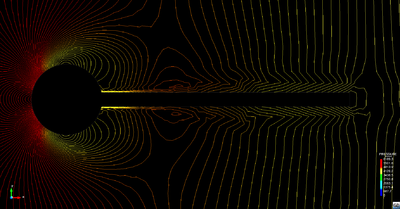Figure 24: Influence of stabilized fractional step iteration on pressure field - Note that the isobars are not perpendicular to the moving structure anymore

The actual influence of this modification can be seen when having a look on the resulting pressure along a coupled interface where now a significant shift of the isobars should be observed indicating the differences between the boundary and the interior. For an illustration the improvement was applied to the well-known strongly coupled benchmark-problem in . Figure 24 shows the isobars during the solution iteration in a time instance at the very beginning of the oscillation of the elastic beam. It clearly can be seen that the additional structure term was taken into account at the interface since the isobars are not orthogonal to the latter. In here we chose a parameter value of ${\textstyle h=0.01}$ which corresponds to reduce the original proposed structural density at the boundary down to ${\textstyle 1\%}$ of its original value. Again it shall be emphasized that this modification only has an influence during the iteration in one time step. At convergence of the pressure state4 at the interface, 3.38 and hence the additional term in equation 3.42 vanishes which restores the orthogonality of the isobars again.

Finally it is worthwhile to mention that this additional structure term gets better and better in its accuracy the smaller the actual time step is. In the limit it even shows the correct structural behavior. That is the smaller ${\textstyle \Delta t}$ the better this stabilization effect. Luckily the artificial added-mass effect behaves just reverse. So the smaller ${\textstyle \Delta t}$ the worse the artificial added-mass effect. Effectively this means the method is all the more effective and accurate the worse the artificial mass effect is which is a very nice characteristic.

(1) Note that ${\boldsymbol {f}}$ is a function of ${\boldsymbol {v}}$ and generally nonlinear.

(2) See , page 41 for a detailed elaboration of the equations in a fractional step process.

(3) Effective mass means the the mass which responds with interia forces to a given movement of the fluid boundary

(4) Note that convergence at the interface might be checked for the displacements, the pressures or both together. In cases where only a convergence of the displacements is checked a negative influence of the stabilization technique can not be excluded since a convergence of displacements does not guarantee a convergent pressure state.In these cases hence the additional stabilization term may introduce accuracy errors or may even cause the simulation to fail.

# 4 Improving the computational efficiency

In FSI scenarios it is necessary to solve the whole coupled physical system of fluid field and structural field under suitable coupling conditions at the interface within one, typically iterative, simulation. Having the anyways computationally demanding single-field simulations, this generally increases the computational costs critically - depending on the chosen solution approach in some situations more and in others less. So strategies to improve the computational efficiency on any level play a crucial role. To this end, we want to present within this chapter several strategies that can be applied in different kind of situations .

In the first section we will thereby concentrate on parallelization techniques. In here different computer architectures will be analyzed briefly from a general point of view and in particular in terms of how they offer possibilities for improvements in the context of an FE-analysis. Furthermore different measures to allow for an evaluation of the quantitative benefit of a parallel environment are to be introduced. They are going to be used for efficiency analysis in subsequent chapters.

In the second section we will focus on a particular efficiency problem given for the embedded approach, i.e. the necessary spatial searches. In this context two different strategies will be introduced that were adopted and customized to face this problem as effective as possible. Since these strategies were adopted from a vast and living field of science, we will stick here only to the main ideas in the particular context of the embedded FSI analysis. All of the discussed strategies eventually were applied in the course of this monograph.

## 4.1 Parallelization techniques

Parallel computing is a technology that enables to divide larger tasks into multiple discrete subtasks according to the divide-and-conquer principle. These subtasks are executed simultaneously and therefore allow an efficient improvement of computational performance. As there is such a large demand for powerful resources and parallelization techniques in the field of simulations literature provides a vast offer of papers and books dealing with parallel computing. In the framework of this chapter we mainly refer to ,  and . Particularly, the book of Michael Quinn, , is highly recommended as it provides a basis for programming with OpenMP and MPI in C.

### 4.1.1 Potential and challenges of parallel computing

The technology of high performance computing provides an enormous potential which can be exploited in the field of Finite Element and FSI simulations. The most attractive arguments for dealing with parallel computing in the course of this monograph are summarized in the following overview:

• Reduction of computation time: The simulation can be computed in a fraction of the time needed by a sequential computation. By dividing the problem in N processes, the simulation can be computed up to 1/N times faster.
• Solve larger simulations: Larger simulations of a specific FSI problem e.g. caused by a much finer fluid mesh or a smaller time step can be computed in the same order of time. A computation which is N-times more expensive can be run within the same time.
• Concurrency: Several simulations can be executed simultaneously.
• Parallelization of FEM: The idea of the spatial discretization of a fluid or a structure into small elements allows to decompose the domain into pieces which are assigned to multiple processors. Due to the fact that the element-wise contributions are separately assembled into the global stiffness matrix, the finite element computations can be executed on each processor separately.
• Making use of the powerful Spanish Supercomputing Network (Red Española de Supercomputación)

Distributing the tremendous computational effort for a complex FSI simulation to multiple processors such that the computational efficiency improves, however, also brings some tricky challenges. The following list shows just the most significant challenges which we will face within this monograph:

• Larger implementation effort compared to sequential programs: In general, parallel code is much more complex than serial code. Multiple instructions are executed at the same time, but there might also be a data transfer between these instructions. Special functions are required to control the data transfer in order to avoid e.g. memory access conflicts. Also debugging is much more complicated.
• Load balancing: How to distribute the work between the processors? A perfect load balancing is reached, when all instructions executed by the processors are finished at the same time. The code is imbalanced if instructions need different times to finish their work and the processors are waiting for other processors to finish. This results in unused computing potential which decreases the efficiency of the parallel code.
• Dead locks: Several instructions are waiting for the other to finish but none of them is ever able to finish.
• Data transfer: When a process generates data which are processed by another one, they need to exchange data. This requires that the processes are executed in a certain order. Setting up the communication, synchronization and the order of transferring data is a difficult part of parallel computing.
• Race conditions: Processors are accessing the same memory at the same time resulting in a reading or writing conflict.
• Scalability of the problem: Describes the capability of the parallel code to handle larger amount of data by an increased number of processors. This is especially important to achieve in the framework of this work because large simulations for benchmarking will be used. The scalability is limited by the memory bandwidth in desktops equipped with multi-core processors .
• Overhead: The effort for managing the division of tasks into smaller packages, load balance between the processors, the communication, synchronization or also inclusion of external libraries requires a certain amount of time which is called overhead. The overhead always has to be compared to the time saving gained by the parallelization. When the overhead is larger than the time saving, the parallel simulation even gets slower than the sequential simulation.
• Efficiency of parallelization: Before parallelizing a code, one needs to think about the question: Is it worth to use parallelization? If the solution of a 3x3 matrix is supposed to be parallelized with many processors, the parallel computations will be even slower than the sequential computation.

### 4.1.2 Shared vs. Distributed Memory Machines

A very fundamental question in parallel computing is how to organize the memory which every processor is accessing in order to solve the physical problem. The most basic architecture is the uniprocessor (figure 25: a single processor accesses the main memory via a bus which is also connected to the input-output system (I/O). In order to reduce the access time to the main memory, a cache is interposed between the processor and the memory. Frequently used data is copied to the cache from which the processor can read much faster than from the main memory.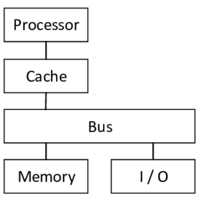Figure 25: Architecture of uniprocessors - A single processor accesses the main memory and the I/O systems via a bus.

Thinking in terms of parallel computing, a natural way to realize a parallel computer architecture would be to add multiple of these processors to the same bus and give them access to the same memory. Systems which are based on this principle are called shared memory machines (SMM) (figure 26). Whenever any processor is invoking a change of data in the memory, these changes are also visible to the other processors.

A main drawback of SMMs is that the shared memory and the bus feature a limited bandwidth, which is divided among the multiple processors. An alternative architecture is provided by the so-called distributed memory machines (DMM) (figure 27). In these architectures each processor addresses its own memory which is not accessible by the others. Therefore each processors can exploit the full bandwidth to its own memory. Nevertheless, DMMs require an interconnection network (e.g. internet or LAN) which is managing the communication between the local memory of each processor. Supercomputers and clusters - which are accessed in the framework of this monograph - are based on the technology of grid computing which is in turn a form of distributed memory architecture.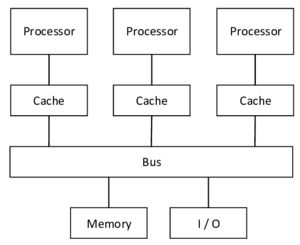Figure 26: Architecture of shared memory machines - All processors share the same memory.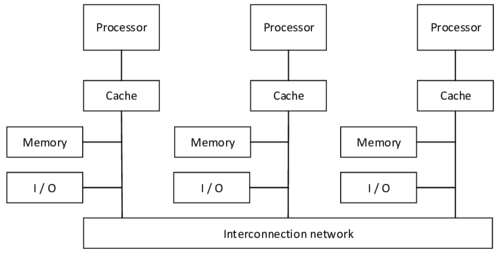Figure 27: Architecture of distributed memory machines - Every processor has its own local memory.

SMMs and DMMs are conceptually different architectures and therefore entail intrinsic advantages and disadvantages. Both architectures will be used in the framework of this monograph. Therefore table 2 presents the main advantages and disadvantages of SMMs and DMMs. The main reason for dealing with SMMs in the framework of this monograph was the easy implementation and the good scalability on a desktop PC. The main reason for using DMM concepts was the potential of exploiting large scalability on a supercomputer.

 Shared Memory Machines Distributed Memory Machines All memory is stored in a global address space which can be accessed by any processor resulting in equal access time to the main memory. If a processor needs to access data from the memory of another processor, messages have to be exchanged between the processors. This in turn causes additional overhead required for constructing, sending and receiving the message. Apart from this, the access time to the local memory is quite fast due to avoidance of interference with other processors. Easy to implement compared to DMMs. This is also because the programmer can avoid partitioning of data. Much more implementation effort because of managing load balancing or data transfer between the processors' local memory. Also debugging is very complex. Memory bandwidth limits the number of processors to be used and therefore limits the scalability. Memory bandwidth is much higher because each processor is only writing to and reading from its own local memory and can therefore use the full bandwidth of the memory. As a result, more processors can be used effectively, manifesting one of the main reasons for using DMMs. The scalability is much larger compared to SMMs and only limited by the interconnecting network. Cache coherence: If a processor is modifying shared data which are stored in the cache memory of each processor, the changes need to be propagated to the cache of the other processors. No problem of cache coherence because there are no processors which may overwrite data in the local memory of other processors.

### 4.1.3 Quantitative performance evaluation

In order to be able to evaluate the improvements achieved by parallel computing and see how the performance of the parallel code is improved with an increasing number of processors, some basic quantitative measuring tools are needed. They are presented in the following. These are tools which will be applied in the later course of this monograph.

Execution time

The execution time of a program is the time between the start of the execution until the end of all computations. Supposed that not every part of the code is parallelized, there is the execution time for the serial code ${\textstyle t_{Serial}}$ and the parallel code ${\textstyle t_{Parallel}}$:

 $t_{Exec}=t_{Serial}+t_{Parallel},$
(4.1)

whereas

 $t_{Parallel}=t_{Calc}+t_{Comm},$
(4.2)

with ${\textstyle t_{Calc}}$ the time required for the calculations and ${\textstyle t_{Comm}}$ the time spent for all communication processes (waiting for messages, sending, receiving messages, ...).

Speedup

The speedup indicates the improvement of the parallel computation with n processors compared to the sequential computation using just one processor. The ratio of execution time with one processor ${\textstyle t_{1}}$ and the execution time with n processors ${\textstyle t_{n}}$ forms the speedup:

 $s_{n}={\frac {t_{1}}{t_{n}}}.$
(4.3)

Optimally, one can reach a speedup of n when using n processors, but typically in practice, the speedup is smaller than n due to e.g. overhead or not ideal load balancing.

Efficiency and scalability

The efficiency describes the relative improvement achieved by parallel computing and is therefore a convenient measure for the processor utilization. The efficiency is computed by normalizing the speedup with the number of processors as follows:

 $e_{n}={\frac {s_{n}}{n}}.$
(4.4)

The maximal achievable efficiency is 1. The larger the achieved efficiency, the larger the scalability, which is defined as the ability of a parallelized code to increase the number of processors efficiently when increasing the problem size of the simulation. But there is no generally accepted measure for scalability. Proposals are e.g. given in  and .

Amdahl's law

The impact of the sequential part on the achievable speedup as the number of processors increases is described by Amdahl's law. Let ${\textstyle s}$ be the sequential part of a program code defined as ${\textstyle s\in [0,1]}$. Then, the ideally achievable speedup can be calculated with the following formula:

 $s_{n}={\frac {1}{s+{\frac {1-s}{n}}}}.$
(4.5)

By increasing the number of processors, the speedup is converging as the next formula is demonstrating:

 $\lim \limits _{n\rightarrow \infty }{s_{n}}={\frac {1}{s}},$
(4.6)

As seen in the equation, the speedup is in fact bounded by the amount of sequential code. This implies that to achieve the largest possible speedup the sequential part of the code has to be kept as small as possible . In essence this law conveys the significant message for this monograph to parallelize as much as possible to get the best benefit from parallel computing.

### 4.1.4 Parallel computing with MPI

The commonly used standard application programming interface for managing the communication between processors in distributed memory systems is called MPI (message-passing interface). By sending messages containing a part of the local data of a processor via the interconnection network to another processor, this processor will get indirect access to these data - as already explained in section 4.1.2. The message-passing model is ideal for being used on supercomputers and clusters.

Using MPI to parallelize a program code can be very complex and time-consuming as it effects many parts of the program structure. In order to get a comprehensive understanding of how MPI is effectively used in Kratos and which implementation work has to be done for this project, let us consider the following flow chart in figure 28. It shows a general simulation process in Kratos, e.g. a CFD analysis.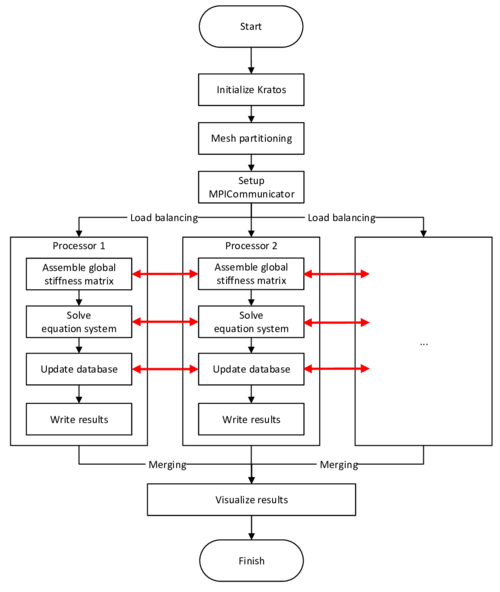Figure 28: Flow chart for a simulation in Kratos parallelized with MPI - The mesh is partitioned into subdomains which are assigned to multiple processors. These solve the simulation locally within the subdomain. The red arrows indicate the communication between the processes.

The first significant step after initializing Kratos is the partitioning of the domain into smaller subdomains which will be distributed later to the processors and stored on the local memory of each processor. This partitioning should be done such that the communication between the subdomains at the interface is minimal and the number of simultaneous data transfers is maximal as additional time for communication increases the overhead. Geometrically this implies that the surfaces of the interfaces should be as small as possible. In Kratos, the external library METIS was chosen to do this partitioning as optimal as possible. In figure 29 such a partitioning of a meshed cube can be seen, on the left with 4 processors and on the right with 16 processors.

Each subdomain contains an interface mesh to each other subdomain with which it shares nodes. These information about interface nodes are required for the solution process as any movement of an interface node effects both subdomains it belongs to. In Kratos, these data are stored in the MPICommunicator class which sets up a communication plan based on these data. This in turn requires the creation of an additional data structure for the unstructured graphs. Furthermore, the class cares about the avoidance of dead locks.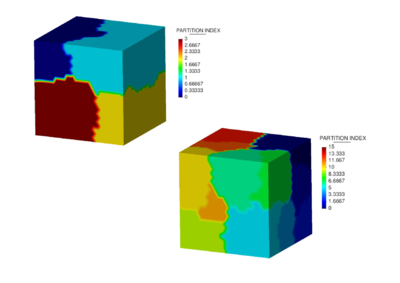Figure 29: Domain partitioning of a cube tetrahedra mesh - On the left hand side the cube is decomposed into 4 subdomains and on the right hand side into 16 subdomains.

Subsequently, the subdomains are equally distributed to the processors. In each subdomain, the contribution of the local elements to the global stiffness matrix is computed. This setup of the global stiffness matrix in parallel is done by the external library Trilinos. Based on the stiffness matrix, the local equation systems are solved by each processor. The solution of each interface node needs to be updated afterwards as the solution variables are superposed at the interfaces. Finally, the results are written and visualized in one file.

The set of functions required for the parallelization of code in MPI is already available in Kratos, such that the Python script could be easily customized to work in parallel. Finally, the script can be called via the terminal with the following command:

 mpirun -np 4 python script_name.py.

### 4.1.5 Parallel computing with OpenMP

The application programming interface which is commonly used for shared memory programming is OpenMP (open multi-processing). The main reasons for using OpenMP are that it is easy and flexible to use for implementation and it supports the portability between different platforms, which is why it was also used in the framework of Kratos. OpenMP is based on parallelizing program code on the level of loops by dividing the loop into independent iterations which are executed in so-called threads - sequential executable code blocks of a process.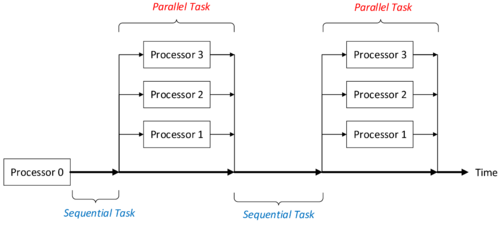Figure 30: Function principle of multithreading - One master-thread is responsible for executing the sequential tasks and for creating the threads in parallel sections.

Multithreading is the key characteristic for OpenMP and basically the main difference to MPI. Whereas in OpenMP one thread is active from the beginning till the end of the program and additional threads are dynamically activated throughout the course of the program, in MPI all the created processes remain active from the beginning till the end of the executed program. This also enables incremental parallelization, which is a significant advantage of OpenMP compared to MPI. In essence, this means that the sequential code can be incrementally transformed into a parallel code giving the programmer the possibility to concentrate on parallelizing the most time-consuming blocks of the code without changing the code structure significantly. This is not possible in MPI .

As already indicated, OpenMP is mainly used for the speedup of loops. In the framework of this monograph we will concentrate on the parallelization of for-loops as it is quite easy to implement. Particularly, it will be applied to loop over all finite elements of a discretized domain. In Kratos, this is done by simply partitioning the model into N-1 elements, wheras N is the number of used threads and one thread has the functions as master thread. Within the for-loop the compiler distributes these partitions to the threads which are executed by the processors. The syntax of the corresponding key command is as follows:

 #pragma omp parallel for.

This command indicates that the following loop is executed in parallel. It is important to realize that all the threads share the same database stored in the same memory, Therefore one needs to take into account race conditions during the implementation. This is especially the case when changing data stored on nodes, which belong to elements of different threads.

## 4.2 Spatial search

The techniques of parallel computing, as they were discussed above, offer a way to improve the computational efficiency in fluid-structure interaction analyses. In fact they are already widely spread in this context and generally not restricted to any specific solution procedure. In terms of the embedded approach, however, there is another important factor, that is influencing the computational efficiency and hence requires a dedicated solution beyond a pure parallelization.

In the embedded method we are letting meshes overlap without specifically define a common boundary. The actual coupling, however, requires an exchange of quantitative information along an interface, which therefore first has to be identified. As a consequence of the missing technical links between the meshes both the information exchange and the identification of the interface are somewhat depended on spatial search techniques, that are commonly not necessary in a body-fitted approach. Spatial searches, though, are computationally very demanding, which is why the choice of proper methods becomes particularly important. In the following we will briefly discuss the corresponding methods that were applied to this purpose throughout this work.

By construction the embedded approach contains two different steps for which a spatial search strategy has to be applied. That is:

1. Given a structure node we have to find the fluid element in which it is contained (necessary for the mapping of quantities at the interface) and
2. given a fluid element we have to find the embedded structure nodes (necessary when we want to check for intersections and hence identify the interface).

Both steps have in common, that basically either every structure node has to be checked against each fluid element or vice versa, which without any improvements ends up in a search algorithm of complexity ${\textstyle O(N^{2})}$. For highly refined meshes this quickly leads to an explosion of the computational costs, knowing that for instance the mapping of pressures or velocities has to be performed at every time step in each iteration. Luckily, however, a spatial search of this type poses a classical problem in computer science for which various techniques are available. Two powerful methods that were incorporated into the given embedded approach shall be introduced conceptually in the following. The aim is to provide a basic understanding plus the necessary key words for a possible literature research. Neither technical details will be elaborated nor any exhaustive comparison will be provided. Instead we are focusing in later chapters on their actual performance in the present practical application.

Besides of the fact that both of the above searching steps principally require to check every fluid element against each structure node, there is an important difference that is crucial for the choice of a proper spatial search method: In case where we want to identify intersections, we are searching for an entity in the domain of the structure, whereas in the other case we are searching for an entity in the domain of the fluid. Both domains are typically differing significantly in their shape. The fluid domain for example often is modeled as a convex hull over the structure, such as a block or a channel. The structure by contrast takes in general an arbitrary shape.

One method that is very well suited for regular distributions and superior with convex shapes is the spatial search based on bins. A bin is a data structure which basically divides the space into a number of regular cells as indicated in figure 31. Each cell of a bin knows about the entities it contains or it is intersecting with. So given a position in space we can ask for the corresponding cell and hence for the entities contained in this cell.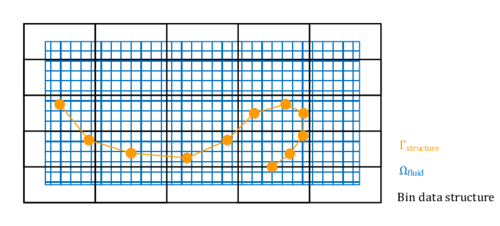Figure 31: Bin data structure - The picture shows the concept of a bin data structure applied to the situation of an embedded FSI scenario. Note that the situation equally holds for 3D.

Generally it holds, the more regular the shape on which the bin is based, the more efficient the search. In fact if the shape is regular or convex, this method is superior compared to other techniques. Since the fluid domain often is regular in an embedded approach the method performs in particular efficient when applied to the fluid domain. Because of that the bin-search is the method of choice for case 1 in the enumeration above. Here the bin data structure is based on the overall fluid domain whereas each cell knows about its containing fluid elements, e.g. tetrahedra. So to search for the fluid element in which a given structure node lies we create the bin data structure once and then with each new search ask for the cell in which the given structure point lies. The cell in turn holds a list of possible candidates. All we have to do finally is to check whether the structure node lies within one element of this small subset of all fluid elements. By that the complexity of the search drops from ${\textstyle O(N^{2})}$ to ${\textstyle O\left(log(N)\right)}$.

This is, however, only true for convex, regular shapes of the search space. In fact the computational effort quickly rises with more complex or non-convex shapes. In all the latter cases other alternatives, such as tree-based search methods, may perform better since they are taking into account the spatial distribution of the underlying data. This is why for case 2 of the enumeration above, an octree search was implemented.

In case 2 we had to find the embedded structure nodes of a given fluid element in order to be able to identify intersections and hence the common interface. To efficiently search for respective nodes, the structure is represented by an octree. More precisely, a given domain is divided into eight equal cubes, which are again partitioned into eight equal cells be it that a cell contains elements of the structure. This process is performed several times up to the desired level of refinement1. As a results the structural domain is decomposed by a tree-like data structure, i.e. an octree in 3D or a quadtree in 2D, respectively. The idea is conceptually illustrated for the 2D case in figure 32. The method applies accordingly in 3D.

The idea now is that the domain in which the octree was created not just contains the entire structure but also comprises the domain of the fluid. By that the single cells do not only know about embedded structure nodes, but it is moreover also possible to assign to each fluid element the set of cells of the octree which are intersecting with the corresponding fluid element. The respective cells are here obtained by applying the algorithm from Akenine-Möller . Based on this linking, the effort for the identification of the intersections between the fluid and the structure elements can then be eased significantly for basically the same reason as already in the bin-search: Instead of checking every fluid element against each structure element we are for a given fluid element just checking a small subset of all the structure elements for intersections. This reduces again the complexity to an order of ${\textstyle O\left(log(N)\right)}$.Figure 32: Principal setup of a quadtree - The picture shows how a quadtree is constructed from a given point data set (structure points). Its is conceptually the same for 3D where we subdivide into 8 different cells on each level (octree).

Different to the bin search, however, this method particularly performs well for arbitrary irregular shapes. Figure 33 shows in this context a large scale example from another application field. The advantage is obvious when noting that the octree leaves (cells at the lowest level of the octree) are closely concentrated along the boundary of the structure. Again transferred to our case case, this reduces the number of fluid elements that have to be checked for an intersection to only a few possible candidates.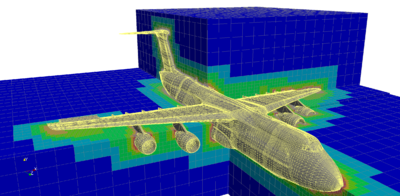] Figure 33: Octree mesh around a complex airplane model - The picture was taken from Zudrop in 

This reduction of complexity for highly irregular shapes could also be achieved by tree-based structures other than the octree, for example by using a k-d tree instead, which in the latter case might even be more robust. But typically the octree is faster compared to comparable techniques. Moreover practical experiences throughout the developments in this monograph showed that it is sufficiently robust in case of the embedded method which is why it is justified here to use this more efficient method instead of a k-d tree for example.

At the end of this chapter it can be summarized: For the two essential spatial searches in an embedded approach, a bin-based search and an octree-based based search are applied. Both are designed to reduce the computational costs connected to the spatial search significantly. Nevertheless, the spatial search in an embedded approach can be considered as disadvantageous compared to a body-fitted approach where these searches are not needed for a coupling. Since on the other hand, however, the embedded method is not dependent on any mesh-regularization, the actual advantage or disadvantage in terms of the computational effort remains to be investigated.

(1) A detailed description of the specific algorithm that is applied here, also with regards to its computational aspects, can be found in 

# 5 Development & simulation environment

In the course of this chapter Kratos as an FEM-based multiphysics solver will be introduced in conjunction with EMPIRE (Technical University Munich, TUM), a coupling software that is able to carry out co-simulations with an arbitrary number of solvers. Both software packages together form the environment of the entire developments and simulations in the remainder of the present monograph. Thus in order to facilitate the understanding of the later discussed implementations plus also to provide a short reference for people from either development teams, the essential concepts of both software packages shall be introduced in the following.

To this end, the first part of this chapter focuses on Kratos and in particular on its main class structure as well as the principal work flow which arises from the FEM-specific implementations. Based on that it will be explained how the problem is formulated within the so called Model Parts and how Kratos approaches the solution of the latter by a distinct segmentation of the single solution steps. This is important since it affects the later implementations significantly. The explanations mainly follow the descriptions in [6,49]. A more user-oriented format of the principal workflow can be found in .

As a goal of this work, Kratos is to be applied together with other solvers in a co-simulation environment, in particular with Carat, the structural solver of the Chair of Structural Analysis at the Technical University Munich. This is where EMPIRE comes into play. EMPIRE is used as control and communication instance in this regards.

The second part of this chapter deals with an introduction to the basic concepts of EMPIRE. It starts with an outline of the principal layout of a respective co-simulation and afterwards concentrates on how different solvers can be connected and linked together to a co-simulation network. Here focus is set on the interface between EMPIRE and the connected solvers, i.e. the EMPIRE_API. The chapter then closes with a short description of different intern features which are necessary for a proper co-simulation, such as e.g. filter routines. Explanations in terms of EMPIRE mainly follow the descriptions in . In there also two benchmark examples can be found.

## 5.1 Kratos

Due to the increasing demand for more and more realistic simulations of engineering problems the separate consideration of occurring physical phenomena (e.g. fluid mechanics, structural mechanics) is not sufficient any more. Rather more, it is necessary to account for the interaction between such fields leading to the coupling of physical quantities.

Those coupled fields result in partial differential equations which can be solved by means of the finite element method. Many conventional FEM codes are catered and optimized to the solution of physical problems including only one single field. The interaction of two or more fields therefore requires an external program managing the interface between the FEM codes, which at the same time lowers the flexibility towards other problems. This is, where Kratos as a unified software environment comes into play.

Kratos is an open-source software for solving multiphysical finite element problems. It is developed at CIMNE with the aim to provide a high level of data structure flexibility, modularity and reusability of the code. These advantages can be assured by a code written in the object-oriented language C++. C++ supports a generic programming which can provide a package of geometrical elements and algorithms that are widely decoupled from the specific physical problem. Therefore, new FE-codes can be easily added to the basic structure of Kratos. The object-oriented approach is particularly suitable for the implementation of finite element concepts. All these aforementioned design principles form the requirements for the efficient solution of multidisciplinary problems.

In Kratos, the objects in the framework of its object-oriented structure are constructed based on the general finite element approach. A very fundamental class structure can be found in figure 34 which at the same time represents the general work flow of a finite element analysis in Kratos. In the course of this chapter these concepts and the relation between these objects will be introduced and linked to the idea of a multidisciplinary framework based on FEM.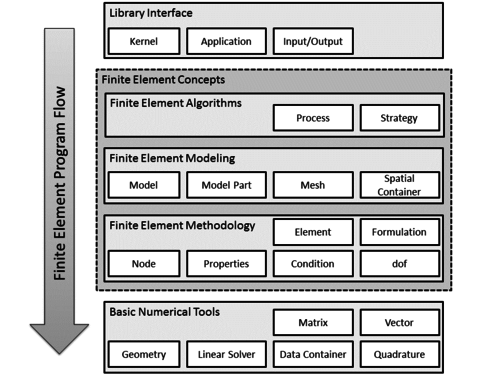Figure 34: Main class structure in Kratos - The figure gives an overview about the essential classes and their main objects in Kratos (adapted from ).

In view of the modularity and flexibility of including new physical phenomena Kratos distinguishes between the kernel responsible for numerical computations and programming and the actual physics of the problem which is implemented in separate applications. This distinction is very important to mention because it allows to define new applications characterized by an own set of dofs, variables and elements whereas the underlying finite element methodology and solution algorithms are managed by the Kratos kernel. Applications such as fluid-structure interaction can also depend on other applications such as in this case e.g. the fluid dynamics application. The interface between these applications manages the communication and is also controlled by the Kratos kernel. These relations are illustrated in figure 35.

In order to ensure a time-effective development process and to provide a high level of flexibility in executing physical examples which access the application libraries, Python is used as scripting language. During this so-called end-user development the compiled Kratos libraries are imported as required and thus enables the direct access to the functions within these libraries without recompilation.

Referring again to figure 34, the kernel and the applications manage the library interface. An additional element within this main class - the input-output module - gives the user the opportunity to include his own concepts without touching the application itself.

All the applications have the finite element core in common which handles the solution process, discretization and the numerical description on element level. In order to understand the object-oriented implementation of the FE approach in Kratos, it is necessary to explain the most basic entities in Kratos in a bottom-up approach. A graphical summary is given in figure 36. Nodes, elements and conditions represent the basis for the finite element formulation as it is the core idea to divide the geometrical domain into simple geometries (e.g. triangles or tetrahedra) which can be treated by a set of fundamental algorithms. The very basic entities of such geometries are Nodes which are characterized by a unique ID, the spatial position and a list of dofs (e.g. fixed displacements in x-direction). The dof class is basically described by its variable, the state of freedom in terms of any Dirichlet boundary condition (fixed or free) and its actual value. The dofs together with all variables are collected in a database in two different containers: nodal data (non-historical data only storing the current value as e.g. the list of adjacent nodes) and solution step nodal data (historical data storing both the current value and values from previous time steps - mainly variables related to the time iteration).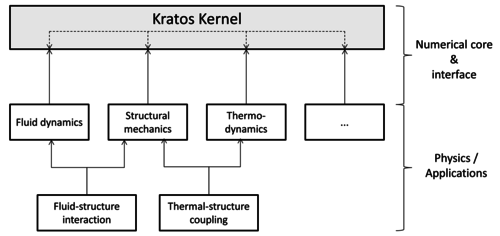Figure 35: Relationship between kernel and applications - Kratos separates the numerical core and FE description from the physical applications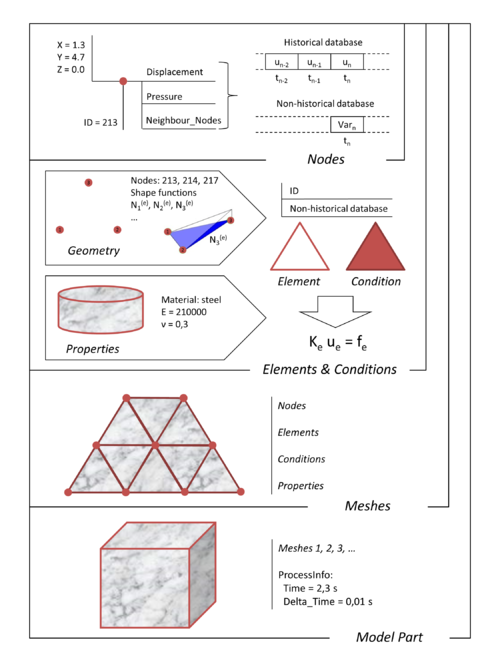Figure 36: Geometrical components describing a model in Kratos - This figure gives a graphical overview over the most important geometrical objects, their properties with reasonable example values and their mutual relationships

A collection of nodes leads to the geometrical definition of an element which itself is implemented in a separate class Geometry and also contains FEM-specific data as the shape functions or the Jacobian at the corresponding node. The Geometry class therefore constitutes the foundation for the definition of an Element which contains a large part of the physics of the FEM problem. Within an element, all the available information are collected to compute the elemental matrix on the LHS of the fundamental local linear system of equations as well as the RHS. These matrices and vectors are later assembled to the global system of equations. Contrary to this, conditions are faces of a finite element directly at the model boundary, which implies that they are used to impose boundary conditions to the system. Any intrinsic information of the elements as material properties of a structure are stored in the Properties class.

All these data are practically bundled within the Mesh class as a container storing the nodes, elements, conditions and properties constituting the region of interest in the domain to be simulated. Several meshes can again be collected to a Model Part which eventually represents the most important class in Kratos as it comprises all necessary information for carrying out a simulation. Therefore additional data as the process info specifying e.g. the current time step of the simulation are also stored as part of the model part. During a preprocessing the complete model part has to be created which then forms the basis for all relevant simulations.

After having defined the main components which completely describe a model in Kratos, the system of equations needs to be solved with a solver which can be chosen according to the requirements of the individual problem. The complexity of solving such huge systems of equations results in a variety of solvers which are at the same time executing a bunch of algorithms. In Kratos this is in general implemented by means of the Process class. The latter handles different tasks ranging from computing the normal of a triangular element to the search for adjacent elements of a node. An important derived class of Process is the Strategy class which is managing the solution steps of the FEM problem. For every problem the finite element algorithms can be uniquely separated into the following basic steps:

1. Time iteration: requests the local contributions from each element (more precisely the stiffness matrix, damping matrix and mass matrix) to the global system matrix and the residual vector as well. These matrices and vectors are combined to form an effective matrix and effective residual vector by using a certain time iteration scheme.
2. Build equation system: assembles the effective terms of each element to one global system matrix and a global residual vector provided by the time scheme at each iteration step.
3. Solve equation system: having the linear system of equations, this step calls a linear solver to solve the system.
4. Update database
5. Check convergence (if required)
6. Calculate output data (if required)

In Kratos, the time iteration (step 1) as well as the updating of the database (step 4) are combined to the Scheme object. Moreover, steps 2 and 3 are combined to one common object called BuilderAndSolver. These two objects form the main ingredients for every strategy which is implemented in Kratos. It is important to note that Kratos generally uses a monolithic solution approach when solving multi-field problems. That is that the BuilderAndSolver in this case sets up and solves a global system of equations which comprises the problem specific equations as well as respective coupling terms. A partitioned approach is not explicitly implemented.

Completing the discussion about the most important classes in Kratos according to figure 34, it also has to be mentioned the group of numerical tools. It contains some basic auxiliary means provided for the implementation of the FEM. Besides of Matrices and Vectors, there is an object to provide various Quadrature Methods, an object defining essential Linear Solvers e.g. exploiting the sparse global matrix characteristics typical for FEM. Data Containers and the aforementioned Geometry object are further advanced techniques in order to improve and extend the performance of the preexisting C++ libraries.

Having a bunch of classes in Kratos at hand, it is crucial to shortly go into the setup of a simulation. As mentioned before, the model part contains information about the geometry, the mesh and also the imposed initial and boundary conditions. At CIMNE, the preprocessing tool GiD is commonly used to define the full model part graphically, which is automatically transferred to a text file, the so-called .mdpa-file. Additionally, a Python file ProblemParameters.py containing the problem settings - such as the solver setup, the process information or the postprocessor configuration - is generated by the software. That file is read from the main Python script which coordinates the complete simulation process by loading all necessary libraries and physical data, defining the solver and additional process functions as well as managing the solution process. The main script is named either KratosOpenMP.py or KratosMPI.py depending on whether the program is parallelized with OpenMP or MPI. After finishing the simulation process, the results can be visualized with the postprocessing function of GiD.

## 5.2 EMPIRE

Solving coupled multi-field systems using a partitioned approach has the particular advantage that different existing and possibly well-tested solvers for the respective single-field correspondents can be reused as black boxes in a multiphysical analysis. This is not only advantageous from a user point of view but is also attractive since it allows to combine technical knowledge in cooperations across single institutions. A partitioned approach, however, requires information exchange among the single codes which has to be managed in a specific co-simulation environment.

At the Chair of Structural Analysis at the TUM such a modular partitioned solution approach is pursued in order to facilitate cooperations with acknowledged experts in the field of computational mechanics. One specific goal in this context is to be able to perform FSI simulations using Kratos as a fluid solver in combination with the in-house software Carat as a solver tailored to structural problems. To this end the Chair of Structural Analysis continuously develops its own open-source coupling software called EMPIRE (Enhanced Multiphysics Interface Research Engine).

Throughout its development EMPIRE is kept generic and thus allows the simulation of not just two but also several coupled fields. It is for example possible to investigate fluid-structure interactions while monitoring the physical quantities in a control circuit which in turn actively influences the interaction as it is often the case in practical applications. In fact EMPIRE is virtually unlimited in the number of couplings and thus allows n-code co-simulations.

Regarding its structure, EMPIRE principally consists of two components, the coupling code Emperor and the application programming interface (API) which is referred to as the EMPIRE_API in the following. The latter is a necessary interface for a proper communication with the different simulation codes whereas the first manages the entire communication. The general communication pattern in a co-simulation using EMPIRE is depicted in figure 37. Following the terminology in , each link represents a communication instance or a connection between solver and the Emperor. Each connection is used to exchange specific information such as the displacement or pressure field in a fluid-structure simulation. For the sake of consistency output and input are distinguished as it is done in the figure. The Emperor acts as a hub in this network hence the latter as a whole poses a common server-client model in which both bilateral and unilateral communication is possible as indicated in figure 37. It is important to note that the single clients, i.e. the simulation codes, indeed communicate with the Emperor but are running independently and do not have to know anything about each other. The advantages regarding modularity and flexibility are obvious.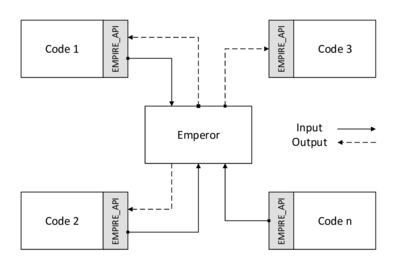Figure 37: Co-simulation with EMPIRE - The figure shows the principal communication within a co-simulation controlled by EMPIRE. (Adapted from )

Since the simulation codes are generally independent and Emperor only manages the respective inputs, each client has to have an interface to EMPIRE, i.e. functions which for example allow to send field data in the expected format to EMPIRE. This interface has to be implemented in the single client codes, like Kratos, according to the given specifications in the EMPIRE_API. The latter is written in C++ but contains a linkage to C which enables it to be used by different compilers in these languages and furthermore by some dynamic libraries like Python. This facilitates the integration of communication routines in Kratos, since its user is intended to operate exclusively on Python level. Regarding the communication between Kratos and EMPIRE in particular the functions of the API which are listed in table 3 have to be imported in Kratos to establish an interface to the Emperor.

 Function Description connect Establish connection to the server (Emperor). getUserDefinedText Get information from XML File. sendMesh Send mesh of the client at the coupling interface to the server. Receiving a respective mesh is not possible yet. Note that EMPIRE can work with multiple meshes but not multiple partitions, so possible pieces should be assembled into one mesh before being sent. sendDataField Send field data to the server (e.g. displacement field on structural solver side). Field data in this context is defined on a mesh, this function thus implies data mapping. recvDataField Receive field data from the server (e.g. displacement field on fluid solver side). Field data in this context is defined on a mesh, this function thus implies data mapping. sendSignal_double Send a simple data array which is not necessarily linked to a mesh. recvConvergenceSignal Request convergence signal from server. disconnect Close connection to the server.

Multiphysical problems usually involve a high number of degrees of freedom. In order to account for the respective computational effort, EMPIRE can also run in parallel. In that regards it is important to note, that EMPIRE is partially parallelized using OpenMP. Furthermore it uses MPI for the connection of the single solvers as well as for the send- and receive-routines. It, however, doesn't do any domain decomposition or partitioning. This significantly restricts parallelization possibilities when using it together with Kratos which allows to partition the physical domain by making use of MPI.] Figure 38: Filters in EMPIRE - Various filters can be applied and linked to modify data along a connection. 

Apart from a parallelized run and the pure information exchange alongside a connection it might also be necessary to modify sent or received data. This is for example the case when connected clients are using non-matching grids for their simulations. Here a proper mapping has to be applied which is transforming the field information at interfaces from one grid to the other. Various kinds of modifications can be done by using respective operators which in the following, according to Wang et al. , are called filters. Filters can be applied both as single operators and as part of a filter network. Figure 38 illustrates this concept. A list with the implemented filters, that also were used in the scope of this work, is given in table 4.

 Filter Description Mapping filter In a partitioned multiphysical simulation each single-field solver usually has a different discretization at the respective interfaces. This requires data mapping between two non-matching grids. Different mapping methods are implemented in EMPIRE to this end. We will use the Mortar Method in the following. Extrapolation filter An extrapolation filter is necessary when data at coupled interfaces has to be predicted in each new time step. Relaxation filter Relaxation can be used in iterative schemes to stabilize the simulation.

All connections and filters have to be defined in a single XML-file which Emperor reads as input. Furthermore the XML-file bundles information about the single clients and contains settings with regard to the coupling algorithm and the iteration sequences. By that it is possible to realize different coupling strategies, both explicit and implicit. EMPIRE finally performs the simulation according to the settings in the XML-file. After having developed a communication interface, also Kratos can be invoked in this file making it possible to carry out partitioned FSI simulations using Kratos and Carat together in a co-simulation environment.

# 6 Kratos' interface to EMPIRE

In order to allow partitioned FSI-simulations using Carat and Kratos together in a co-simulation controlled by EMPIRE, an interface had to be created in Kratos that is able to establish a connection to the EMPIRE_API. As additional requirement, the interface should be designed such that it is not just able to be used within an ALE framework but also ready to be used within the framework of an embedded approach. The implementation and verification of such an interface is topic of this chapter.

In the first part the structure of the interface will be described following its basic classes and their mutual relations which will be summarized in a UML class diagram. It is shown, that the interface is ready to be used in a partitioned FSI-analysis using both an ALE and an embedded approach. In this context still present limitations will be explained. Apart from these limitations for each solution approach a generic process flow can be derived. Since the partitioned analyses in the course of this monograph are all customization of this generic processes, both of the latter will be elaborated in more detail by means of two graphical overviews. A short summary of the arising possibilities will then be given at the end of the first section.

Knowing about the implementation details the functionality of the interface will be verified in the second part. In particular a correct sending of the data, a functioning coupling and mapping as well as a correct overall communication will be shown by means of two distinct examples, i.e. a simple cube with imposed movement of the ground plate and an FSI-simulation of a beam in a channel flow. The results will finally prove a correct implementation which in turn was a prerequisite the later simulations.

## 6.1 Interface structure

In order to allow for co-simulations using Kratos and Carat together in one simulation by connecting it via EMPIRE it is necessary to set up an interface between the latter and Kratos. As already described in 5.2 EMPIRE already includes an API to which Kratos can be interfaced. A respective connection, however, requires additional implementations on side of Kratos which for a proper simulation have to be designed such that they comply with specifications of both software packages. How this implementation was organized and realized as well as how it is used in an FSI simulation is described in the following.

Crucial requirement in terms of the interface was a modular and flexible applicability in Kratos. That meant that at first a whole new application covering all relevant functionalities had to be created and embedded in the given structure. This new application, internally called "EmpireApplication", allows a usage in the same way as all the other applications in Kratos and thus offers a wide range of possible co-simulation scenarios. Figure 39 depicts its general structure.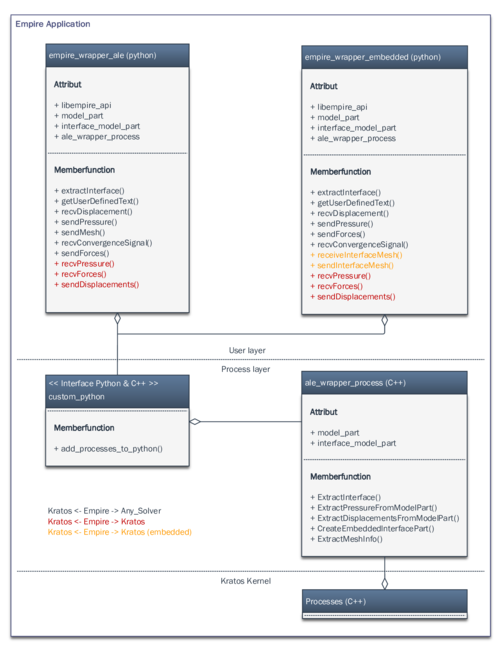Figure 39: Class structure of the Kratos-EMPIRE-interface - The figure shows the class structure of the interface application which wraps EMPIRE functions in Kratos. In terms of a co-simulation, this allows both a connection between several solvers including Kratos (blue functions) and a connection exclusively between several Kratos applications through EMPIRE (red functions). Additional functions (orange) allow moreover a Empire controlled partitioned analysis with embedded meshes.

In a second step the EMPIRE libraries had to be linked to the Kratos environment such that its functions can be invoked in that. EMPIRE is relying on its own libraries. Within one of them, all the functions of the EMPIRE_API, they are already described in 3, are defined. This library had to be linked to Kratos. In favor of compatibility a pre-compiled version of it was used. This eventually enables to link it dynamically in Kratos' Python scripts as needed in single simulations. Within the interface, the dynamically linked EMPIRE libraries resemble in a single object called libempire_api" (see figure 39).

Besides of the compilation also the different programming languages had to be considered. Since the EMPIRE_API is accessible via C-functions but Kratos in contrast uses Python for the setup and C++ for the run of a simulation, a further implementation requirement was a common format to exchange data from either programming language. In this case C-Arrays were used since they are principally processable by all these languages.

Due to the fact that both Python and C++ was used in Kratos, the advantages of both languages were to be exploited. That is why all iterative and computationally expensive routines were written in C++ while all the other serial tasks like input and output operations are directly accessible and modifiable within Python and hence do not need any recompilation. The outsourcing of the different routines required a careful conversion of the different data formats but eventually allowed an optimized performance.

After having created the general environment for the interface, the EMPIRE_API's functions according to table 3 had to be wrapped into that. The actual interface was thereby designed such that, depending on which framework one is choosing (ALE or embedded), the user has access to different features which are each based on the aforementioned API functions but customized to the specific problem. As a consequence two main Python classes appear in the application: "empire_wrapper_ale" and empire_wrapper_embedded. In both cases the interface can be integrated in a Kratos simulation by just creating a single object of either of these classes at run-time. The object then wraps the functions of the EMPIRE_API in the correct format and is consequently referred to as a wrapper in the following.

As already indicated before, some processes of the wrapper are outsourced to C++ as can be seen from figure 39.This obviously implies a connection of the two separate programming environments via an established Python-C++ exchange format. Through this connection the outsourced functions are contained in the C++ class "ale_wrapper_process" which hence covers all program parts that require an iterative or computationally demanding algorithmic such as reading or extracting information from the entire coupled system12. It is also used to extract the common physical interface for an ALE-approach or the embedded structure boundary in an embedded approach. In both cases information about the interface are stored as an extra attribute, which here is called interface_model_part. This facilitates the handling of the wrapper in Kratos significantly.

The C++ functions are just used by the wrapper objects of the two higher lever Python classes and hence do not have to be invoked in any position of the simulation. The latter wrapper objects are the one entities which are needed to set up the communication with EMPIRE. Therein included functions are posing the actual interface and will thus directly be invoked by the Kratos user who intends to set up an FSI-simulation for example. Typical functions here are the sending and receiving of field-information such as displacements or pressures. Most of the functions are simple customized versions of the already explained EMPIRE_API functions from table 3. Note, however, that additional features were included in order to enable:

1. a co-simulation using several Kratos clients at once or even solely Kratos throughout the entire analysis with EMPIRE (highlighted in red).
2. an exchange of mesh information which is is necessary when using an embedded approach(highlighted in orange).

By using all the these functions Kratos can be used together with EMPIRE to run n-coupling co-simulations without any great effort and for both an ALE and embedded analysis. Before, this was not possible. In consequence Kratos can be combined with further analysis approaches beyond the finite element method such as e.g. approaches from control theory. This not only follows the Kratos' philosophy regarding multiphysics but primarily offers various new applications.

There are, however, still limitations. Despite the implemented interface allowing already a co-simulation with both solution procedures, embedded and ALE, there is still a principal limitation, which is imposed by EMPIRE itself. The limitation here arises due to the fact that EMPIRE is not yet designed for embedded approaches and therefore indeed receives meshes from the client, through the function call “sendMesh”, but in turn cannot send mesh information to the single clients, which would require a function call like “receiveMesh” on client side. In an embedded approach, however, the fluid solver has to know the configuration of the current structure mesh in order to be able to identify the actual interface. Principally this can be achieved by receiving the structural mesh either in each time step or by receiving it once in the very beginning of the simulation and subsequently update it with the known displacement field at each instance.

Both of these options are however not yet possible with EMPIRE and still a matter of future project work. Nevertheless, in order to be able to carry out first partitioned analysis in an embedded framework using the features of EMPIRE, the interface contains a preliminary “receiveInterfaceMesh”- and a corresponding “sendInterfaceMesh”-function. These functions allow to exchange mesh information, i.e. node IDs, node coordinates, element IDs and connectivities, separately in the beginning of the co-simulation using the in EMPIRE given options of sending and receiving signals. When doing so, the wrapper automatically assembles the single information to a mesh of the fluid-structure interface in Kratos by using the function “CreateEmbeddedInterfacePart” and stores it as one of its attributes34. Even though this procedure was used within the scope of this work, in order to be able to investigate the capabilities of the embedded method in a partitioned approach, it has to be prospectively exchanged by a respective functionality in EMPIRE.

Having now all features at hand to carry out a partitioned FSI-analysis using Kratos via EMPIRE, two generic processes can be identified for the case of an ALE and an embedded procedure. In this context we are concentrating on the configuration where exclusively two Kratos solvers, one for the CSM and one for the CFD, are coupled. Each process flow and corresponding communication pattern can hence be outlined as done in figure 40 and 41. Partitioned analyses in the remainder of this monograph all follow these processes.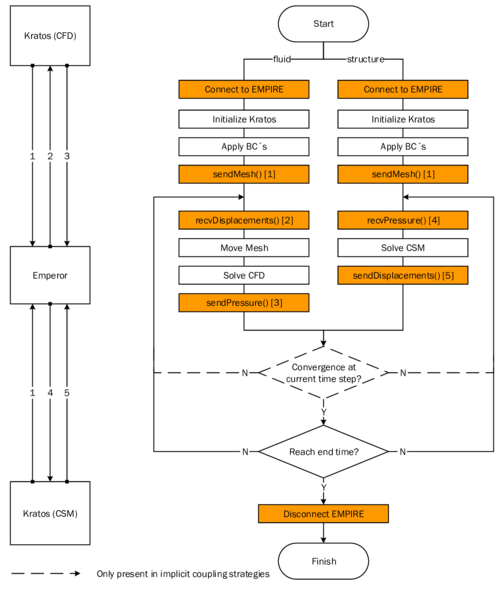Figure 40: Co-Simulation of an FSI problem in an ALE framework using Kratos and EMPIRE - The right chart shows the general process flow as an extension of the generic one from figure 3 by the above described newly implemented features of the EMPIRE interface (orange). The communication between the two different solvers is hence managed by EMPIRE (left).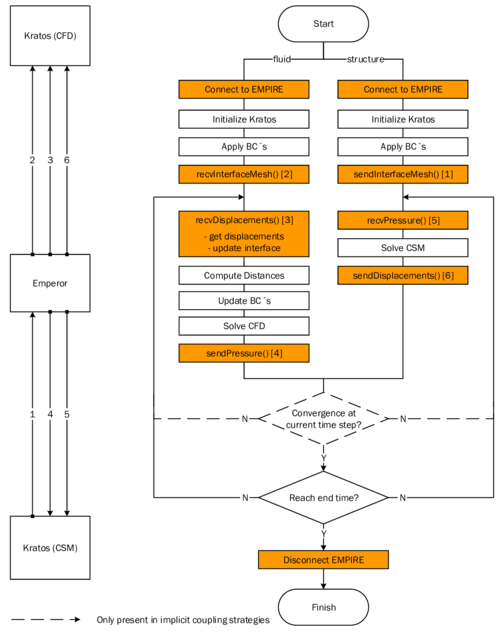Figure 41: Co-Simulation of an FSI problem in an embedded framework using Kratos and EMPIRE - The right chart shows the general process flow as an extension of the generic one from 4 by the above described newly implemented features of the EMPIRE interface (orange). The communication between the two different solvers is again managed by EMPIRE (left). Based on the latter, field data is mapped at the interface according to the embedded approach.

From figure 40 and 41 it can be seen that a partitioned FSI-simulation using Kratos and EMPIRE only requires to invoke a few additional functions from the main Python classes in 39 either before the time evolution starts, during or after it. So the general structure of a Kratos simulation is preserved which facilitates is application significantly. Steps beyond the direct communication such as the mapping between to possibly non-matching grids are handled by EMPIRE internally and do not have to be specifically defined in Kratos.

Note that, as already mentioned in chapter 5.2, EMPIRE does not allow domain partitioning and hence a respective global parallel solution approach. This means that each single branch in the figures 40 and 41 indeed can use internally parallelization techniques, but the global solution process needs to be kept sequentially.

Note also that the wrapper offers the option to send and receive both pressure values and forces. Typically pressure values are sent since they are independent of the discretization unlike reaction forces that vary with changing degree of refinement as indicated in figure 42.The latter fact leads to difficulties when mapping between non-matching meshes and might result in a violation of equilibrium conditions. EMPIRE can, however, map consistently in both situations using the Mortar method.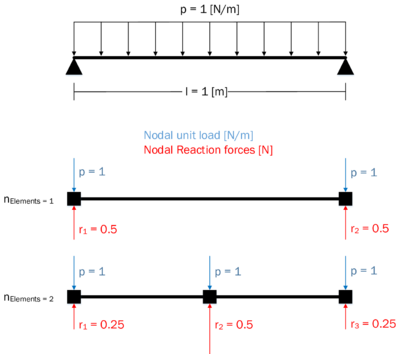Figure 42: Load quantities in dependency of the FE-discretization - The picture shows how the discretization influences the nodal reaction forces, they vary, and the nodal unit loads, they are independent. This is why usually pressure values are mapped between non-matching interface meshes in an FSI-simulation.

At his point it can be summarized: The implemented interface covers all features to connect Kratos to EMPIRE while sticking to the specifications given by the EMPIRE_API. It manages both the communication between the different software packages and hence the different programming languages as well as the internal data management in Kratos. The structure of the wrapper is thereby chosen such that it allows an easy and flexible connection of one or even more instances of Kratos to EMPIRE. This enables various new scenarios of Co-Simulations in which Kratos can be used. Furthermore, preliminary functions allow a partitioned FSI-anlysis using Kratos together with EMPIRE in not just an ALE but also an embedded solution approach. Figure 40 and figure 41 outlined in this context the general process flows where exclusively Kratos-clients are present. This generic processes can be adjusted to realize different coupling strategies5. Furthermore, due to the fact that the clients are independent, it is just a simple step to replace the structural solver of Kratos with the one from Carat. That is that Carat and Kratos can now also be used together in a Co-Simulation environment. What remains to be done is a verification of the interface´s functionality. This is going to be topic of the following chapter.

(1) From an implementation point of view these program parts are included in Kratos as independent processes, which is why the respective class is derived from the parent class “Process” of the Kratos kernel

(2) Remember: All model information are contained in the Kratos object “model part”. That is why the model part appears as an attribute in both classes.

(3) The respective functions are highlighted in orange within the above depicted class structure.

(4) Note that this procedure does not require both clients to be Kratos, also Carat can be used assuming that it sends the same information in the same sequence. In this case, the customized “sendMesh” function would have to be adopted in Carat which is a straightforward task.

(5) By adjusting the input XML of EMPIRE

## 6.2 Verification

Having implemented the interface between Kratos and EMPIRE we can think about models on the basis of which it is possible to verify that the implemented interface conforms to the defined specifications and works correctly. This chapter is dedicated to proof this using a few simple examples. Here we are concentrating on the most important features of the interface. This is enough, though, to guarantee an error-less communication between Kratos clients and EMPIRE.

The testing will be organized as follows:

1. The connection and data transfer is to be tested and verified by using a simple dummy FSI-configuration where a structural movement is manually imposed and transmitted to the fluid domain.
2. Assuming a correct data transfer a correct mapping will then be tested by means of a beam placed in a channel flow.
3. Having tested the essential sequential steps in the communication, a correct coupling of them within the full implicit FSI-analysis of the example from 2. will be tested. A subsequent physical interpretation of the results shall moreover proof a correct overall communication.
4. Finally it is to be shown that for the case of an embedded approach, mesh information can be exchanged via EMPIRE as required.

Case 1-4 will be done using the ALE formulation and hence the respective ALE wrapper-functions. Without proofing it explicitly the results will also be valid for the wrapper-functions in terms of the embedded method.

### 6.2.1 Verification of a correct data transfer

As indicated above, first the connection of Kratos to EMPIRE and the correct data transfer is to be tested. Therefore the model shown in figure 43 was used since it provides a simple geometric setup allowing an easy tracking of the quantities of interest. The model is a two-dimensional rigid square plate placed at the bottom of a cube-shaped fluid domain. The idea is now to manually impose a movement of the plate in positive y-direction, send this movement via EMPIRE to the fluid solver and see what the fluid receives and how it reacts to this. The simulation is thereby such that with every time increment of ${\textstyle \Delta t=0.002s}$ the plate is moved by a displacement increment of ${\textstyle \Delta y=0.02}$:

 $y(t_{n+1})=y(t_{n})+\Delta y,$
(6.1)

where

 ${\begin{array}{ll}\Delta y=0.002&~\forall t_{n},\\\\y(t_{0})=0.\\\end{array}}$
(6.2)

The plate starts with the the movement at the very bottom of the fluid cube and has an identical cross-section such that the fluid is not able to flow across the edges of the plate. Due to incompressibility this requires a zero pressure at the opposite face enabling here a mass flux out of the cube. Obviously one can expect in total a “squeezing” of the fluid domain which finally can be measured. Even though this test scenario is clearly not an FSI-analysis, since it is only a unilateral coupling, it already includes the communication pattern of a such which is why the results of this test also hold for the fully coupled pendant. The communication pattern and the process flow of this test are depicted in figure 44. Note that here an explicit coupling scheme was chosen in which no relaxation filter is present. This was done since we are only interested in the pure data transfer. A manipulation due to a relaxation factor was to be excluded.Figure 43: Verification of the data transfer between EMPIRE and Kratos - Rigid plate in a cubic fluid domain and with imposed movement. The data transfer is tested by comparing the displacement field of the fluid as a reaction to the imposed movement. They have to coincide.

To investigate the movement of the fluid cube the displacements of an arbitrary node at the bottom of the cube was recorded along the simulation process. This has to correspond to the imposed movement of the structure if the communication is done correctly. In fact as shown in table 5 this can be observed. Figure 45 shows the respective results for the fluid cube after a simulation time of ${\textstyle t=0.01s}$. Since the results coincide the data transfer can be considered to be verified.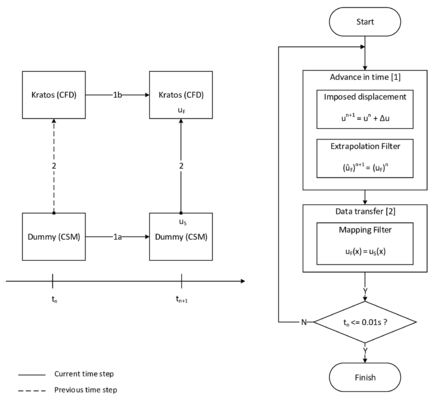Figure 44: Process flow for testing the EMPIRE-Kratos data transfer - The left picture shows how the communication is organized in EMPIRE. As depicted, there is a data exchange between a Kratos CFD- and a Kratos CSM-solver. The latter, however, only sends prescribed displacements (Dummy). The right picture shows the corresponding iterative process flow.

 ${\textstyle Time[s]}$ 0 0.002 0.004 0.006 0.008 0.01 ${\textstyle \mathrm {y_{plate}} }$ 0 0.02 0.04 0.06 0.08 0.1 ${\textstyle \mathrm {y_{node\_A}} }$ 0 0.02 0.04 0.06 0.08 0.1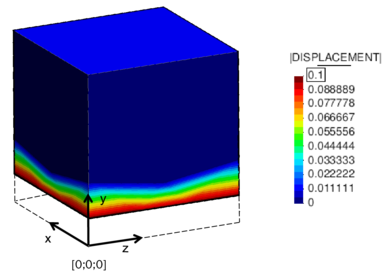Figure 45: Fluid domain with imposed plate movement after a co-simulation with EMPIRE - The picture shows the results of the partitioned simulation for a simulation time of $0.01s$. A significant movement of the fluid domain due to the imposed movement of the bottom plate can be observed. In fact the final displacement corresponds to the one that was expected after $1s$ according to equation (6.2).

### 6.2.2 Verification of a correct data mapping

As seen in the previous chapter, already a simple data transfer requires a data mapping as soon as non-matching grids are present. In particular the displacement and pressure field has to be mapped along the interface of the two domains in an FSI scenario. Using an embedded approach there is obviously no common mesh interface and the mapping is already included in the algorithmic of the method. In a partitioned ALE-formulation, however, a correct mapping has to be taken into account as a separate step. In this context Empire is using a Mortar Method to map between non-matching grids. The latter step in a setup where two Kratos solvers are connected to EMPIRE is to be verified qualitatively in the following.

Testing scenario is going to be a cantilever beam placed inside a channel flow. At the wet interface fluid and structure are each discretized differently in order to have a non-matching grid. This will require a corresponding data mapping between the domains. The respective model details can be found in figure 46. Furthermore the different interface meshes each of the fluid and the structure are shown in figure 47. From a physical point of view the complete setup is posing a flow-induced deflection problem, i.e. a classical FSI problem. For this we know the physical behavior, as will be detailed in the subsequent section, which is why it was taken also for verification purposes in the remainder of this chapter.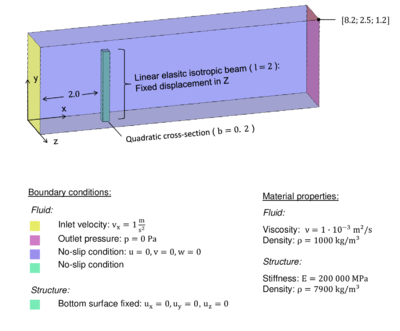Figure 46: Classical FSI problem: flow-induced deflection of a beam - For this scenario the physical behavior is known. The parametrization in conjunction with the fixed Z-displacement of the beam will lead to an X-deflection at the tip of the beam without introducing any flutter or similar dynamic phenomena.Figure 47: Non-matching interface meshes - The picture shows the different meshes for the wet interface in scenario 46

Even though this problem is principally a fully coupled problem, we are in this section only interested in how the data is mapped at the interface. To this end we were running a unilateral coupled analysis of this model, which is similar to the one described in figure 44. Here we let the fluid flow fully develop and afterwards map at each time step the given pressure field at the interface on fluid side to the structure which in turn simulates the resulting deformation. Since we are only interested in the mapping there is no coupling of the resulting displacements back to the fluid which means that we are not resolving the entire fluid-structure interaction. The latter is going to be investigated in the following chapter.

Having simulated this test case one can compare the pressure field at the interface at each time step on structure side and on fluid side. A correct mapping requires them to differ only according to the limitations of the underlying mapping algorithm, i.e. for this case where we are using a Mortar mapping method with rather fine interface meshes, the pressure field on both sides should almost coincide. In fact as can be qualitatively seen from figure 48 they coincide. Since this is a generic process step for all FSI-simulations in this context, one can consider the mapping in connection with the above described data transfer using the Kratos-EMPIRE interface to be verified.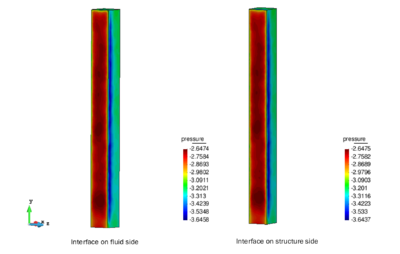Figure 48: Verification of the pressure mapping with EMPIRE - The picture on the left presents the pressure distribution of the fully developed flow field at the fluid´s interface to the structure for a given time step. The right picture shows the received pressure field at the structure in the same time step after the data was transferred and mapped using EMPIRE. The similarity is obvious.

### 6.2.3 Verification of a correct coupling in a complete FSI

Having investigated the data transfer and mapping it is now to be tested and verified whether the complete coupling between the different Kratos solvers via EMPIRE works correctly. To this end we are using the scenario from figure 46. As opposed to the previous section, however, we will now apply a complete bilateral coupling of fluid and structure, i.e. we are simulating the complete FSI. Figure 49 details the corresponding process.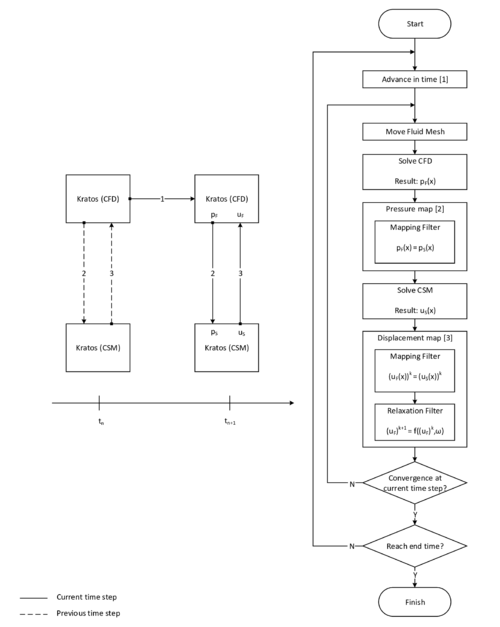Figure 49: Partitioned analysis of the flow-induced deflection of a beam in a channel flow - The right picture shows the process flow.The left figure indicates the corresponding communication pattern.

The aforementioned example is particularly useful for the verification of the coupling since we know the physics of this problem which is a flow-induced deflection (bending) of the cantilever beam. If the coupling of the different domains using Kratos and EMPIRE in a process as depicted in figure 49 works correctly, this physical behavior has to be recovered by the analysis. In the following this is going to be tested in two steps:

1. check convergence in each time step,
2. compare final results and double check physical significance.

Checking convergence is straightforward. Here we are looking at the step where the displacement field is transferred from the structure to the fluid (step 3 in figure 49). Since we are using an implicit scheme with Aitken method, we can test if the coupling formally is successful by checking in each time step whether the received displacement values on fluid side iteratively converge to the sent ones on structure side. To this end the displacement value for a specific node on the structure was compared to the value of the corresponding node of the fluid. Both nodes are highlighted in figure 47.

Table 6 summarizes the results from the analysis for the time step ${\textstyle n=30}$. As can be seen, convergence1 is achieved after ${\textstyle k=4}$ steps. From this one can conclude to a working coupling between the two domains when using the Kratos-EMPIRE interface.

With the interface functions in terms of coupling, mapping and data transfer being verified it remains to test how all the single steps resemble to an overall FSI-analysis. To this end the beam was simulated in the complete FSI context. Intentionally the corresponding model parameters and boundary conditions from figure 46 were chosen to be such that a simple deflection of the beam without any flutter phenomena has to be expected. Note in this context the relevant Reynolds number of

 $Re={\frac {v\cdot b}{\nu }}={\frac {1\cdot 0.2}{1\cdot 10^{-3}}}=200$
(6.3)

 Time step n = 30 Structure (Node S) Fluid (Node F) Aitken factor $u_{send}^{k+1}$ $u_{recv}^{k+1}$ 0,013929187 0,013459557 - 0,013929706 0,013464254 0.01 0,013928829 0,013987717 1,12454 0,013928464 0,013928965 0,998332 0,013928250 0,013928499 1,00624

Despite the comparatively low Reynolds number we neglect the viscous influence of the fluid onto the structure and just use the pressure field as driving force for the structural movement. That is we simply need to transfer the scalar pressure field between the domains instead of the more difficult directional traction forces.

To investigate the deflection of the beam we again use the node indicated in figure 47 and measure its X-displacements as time evolves. The results are recorded in diagram 50. As can be seen qualitatively, the analysis gives exactly what we physically expected to get, i.e. a deflection of the beam in flow direction. Furthermore note that after a while the tip deflection gets non-linear in time which is also what we expect from the bending beam if the deformation becomes large. Figure 51 illustrates the results at the whole setup.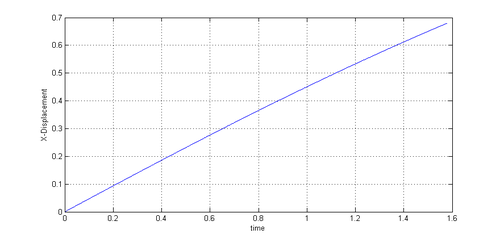Figure 50: X-deflection of a beam in a channel flow - The diagram shows clearly the expectable deflection in X-direction. Note the slight non-linearity as time evolves.

Even though we are not evaluating the results quantitatively, we nevertheless observe that the physical behavior of the model is captured. From a qualitative perspective this clearly indicates a robust and working partitioned analysis. Since in this case the latter was carried out using the newly implemented Kratos-EMPIRE interface we can and have to consider its functionality as verified.Figure 51: Pressure distribution in the channel flow with elastic beam - The figure shows the results for the flow-induced bending of the beam at $t=1s$

(1) Taking into account a tolerance of $1e-6$

### 6.2.4 Verification of a correct exchange of mesh information

As explained in section 6.1, a partitioned analysis using Kratos in connection with EMPIRE requires an exchange of mesh information at the wet interface of the structure. That includes the extraction and sending of the mesh on structure side as well as the receiving of the corresponding mesh data on fluid side. The newly implemented Kratos-EMPIRE interface allows this exchange of mesh information at an arbitrary instance of the simulation with the two specific wrapper functions, “sendMesh”- and “recvMesh”.

Their functionality can simply be verified by applying them to the problem of the beam above. To this end we are omitting the computation of the entire FSI but just concentrate on the exchange of the respective mesh information. That is, we extract1 and send the mesh of the wet part of the structure to EMPIRE using the customized “sendMesh”-function and ask EMPIRE subsequently for it by means of the new “recvMesh”-function. As a result the fluid solver is expected to own a copy of this mesh of the wet interface which eventually allows to apply the embedded algorithmic to it in order to identify the embedded boundary within the fluid domain.

Looking at the results that we get when actually applying these functions to the beam, a correct exchange can in fact be observed. The results are depicted in figure 52, where on the left the original solid beam mesh is shown and on the right the extracted and sent interface mesh. As expected the "sendMesh"-function extracted the wet interface of the solid beam, sent it to EMPIRE and the latter passed it to the fluid solver who asked for it via the “recvMesh”-function. It is obvious that it was sent correctly. With this also the exchange of the mesh-information between different Kratos solvers connected through EMPIRE is verified, i.e. possible.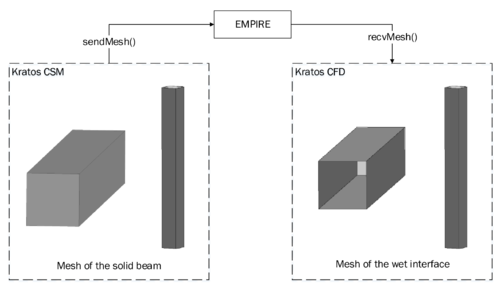Figure 52: Sending and receiving mesh data using the Kratos-EMPIRE interface - The picture shows the results of the exchange of the beam's wet interface among the two different Kratos solvers. Note that even though the graphic shows two meshes, a tetrahedra-mesh on the left and a triangle-mesh on the right, the edges are not represented in order to avoid clutter.

(1) Assuming the interface is tagged as a such in some way, e.g. via a global Variable IS_INTERFACE"

# 7 Interface treatment in FSI problems

Every embedded method requires a proper tracking of the embedded domain and in particular the corresponding interface. Within the present chapter we will first discuss a collection of strategies which allows to represent and track arbitrary structures within an embedded domain. Then we will test its approximation error and influence on the overall solution with a few small- and large-scale examples. The corresponding tests and investigations are carried out at either generic or single-field CFD problems. The results are basis for the FSI simulations in the follow-up chapters.

## 7.1 Geometric description of the FSI interface

Within an FSI simulation based on an embedded approach it is required to provide a representation of the shape of the structure to the fluid solver. An efficient approach to handle this is the level set method based on distance functions which was already discussed in 2.2.4.1. Therefore a set of distance values for each element has to be computed as indicated in figure 6. Due to the fact that this approach is by definition just capable of describing one plane in each element, structures with many geometrical details can not be represented properly. In the following we will step-wisely develop an algorithm to treat structural surfaces of arbitrary complexity which can be categorized according to table 7. For each listed group representing a structural characteristic, the main challenges with regard to the interface treatment are emphasized.

 Surface complexity Visualization Challenges Planar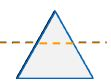As a planar surface has a constant orientation throughout its domain, a globally constant plane is defined in each fluid element resulting in an exact representation of such surfaces. Slight curvature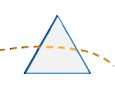The orientation of the surface within a single element is slightly changing, such that the surface can not be described exactly by a plane. However, the approximation error which is made is still comparably small. Sharp edge (geometrical discontinuity)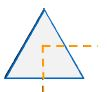A sharp edge located within an element can not be described at all based on a single plane. Essential geometrical information of the structure get lost by the approximation. Local agglomeration of two or more surfaces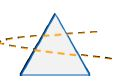If two of more surfaces traverse one element, they have to be reduced to one single plane. Thus the structural information is locally completely lost.

### 7.1.1 Geometric approximation of plane structure surfaces

In the course of this chapter all the ingredients for an appropriate representation of a planar structure will be explained. Its starts with a mathematical description of the implemented algorithmic. After having a basic set of functions at hand, simple test cases will be discussed. As a representative test case we choose a rectangular plate. Furthermore a cube, which consists of six plates enclosing a volume, is tested in order to extend the validation to the general 3D case. The plate and the cube are significant benchmarks which are used for many validation examples in the later course of this monograph. The aim of this chapter is to describe the planar surfaces of these test cases properly by means of the discrete distance function.

In the framework of this monograph, the fluid volume is discretized by a three-dimensional mesh of tetrahedra, whereas the embedded mesh, discretizing the structure, is commonly represented by means of mesh of triangles. On the level of implementation, four basic tasks have to be performed, illustrated in figure 4 in the introduction. After the distances have been calculated they are assigned to the fluid element which in the following is marked as split using the flag SPLIT_ELEMENT.

Because we are generally able to describe with these distance values a plane exactly, also the algorithm for computing the distances, i.e. the distance function, needs to be able to represent a plane exactly. This first chapter explains the implementation of such a distance function. The plane as an infinitely thin structure and the cube as a volumetric structure with plane surfaces serve as examples for verifying the performance of the developed code.

#### 7.1.1.1 Spatial search to identify intersections

As we are interested in the interface between fluid and structure it is necessary to look for all fluid elements which are cut by structure elements along the interface to the fluid. As we do not have any information about the spatial relationship between the fluid elements and the structure elements, a spatial search is necessary to reveal the intersecting structure elements. This procedure is visually explained in figure 53.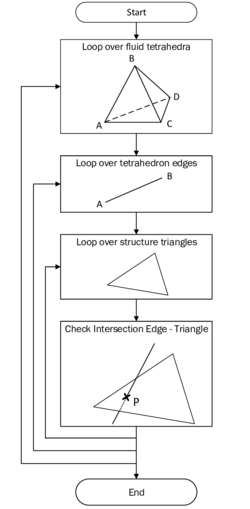Figure 53: Flow chart for spatial search - This flow chart shows the procedure for finding the intersections of fluid and structure elements.

First of all, there is a loop over each fluid element. Due to the fact that there is no general algorithm to identify the intersection pattern of a tetrahedron with a triangle, the algorithm is reduced to an intersection algorithm of an edge of the tetrahedron with the structure triangle. This results in a loop over all six tetrahedron edges and for each edge again in a loop over all structure elements.

Identifying an intersection of an edge with a triangle is a basic procedure. We use therefore the algorithm proposed by Möller . This code is effectively checking the existence of an intersection between a ray and a triangle. Having found such an intersection node, however, it is with this method still not clear whether this point ${\textstyle P}$ is lying on the edge between the points ${\textstyle V_{1}}$ and ${\textstyle V_{2}}$ or somewhere else along the ray (see figure 54).Figure 54: Ray-triangle intersection - An edge with points $V_{1}$ and $V_{2}$ positioned on a ray is intersecting a triangle at the point P.

To this end, the following geometrical consideration needs to be conducted:

 $||{\overline {AP}}||\leq ||{\overline {AB}}||\wedge ||{\overline {BP}}||\leq ||{\overline {AB}}||$
(7.1)

This basically implies that the connecting line of an edge point with the intersection point can not be longer than the edge itself. If this was the case, the intersection point P would not be an element of the edge ${\textstyle {\overline {AB}}}$.

After doing this procedure for all six tetrahedron edges of an element, each intersection node is put into a container which is set up for each element. This allows us to keep track of all intersection nodes. Let ${\textstyle P_{1}}$, ${\textstyle P_{2}}$, ... be the intersection nodes and ${\textstyle I_{Tet}}$ be the set of all intersection nodes, then ${\textstyle I_{Tet}=\{P_{1},P_{2},...\}}$. Then, the cardinality of the set ${\textstyle |I_{Tet}|}$ describes the number of intersection nodes for an element and characterizes the intersection pattern which is utilized in the following.

#### 7.1.1.2 Computation of structure-approximated plane

Obviously, in the case there is no intersection between the fluid and the structure element, i.e. ${\textstyle |I_{Tet}|=\emptyset }$, no distances are computed, such that the fluid element keeps the initially set distance value of ${\textstyle +1}$.

For each fluid element which is cut by the planar structure surface, we can have different intersection patterns comprising up to four intersection nodes. The intersection cases are listed in the following overview:

• ${\textstyle |I_{Tet}|=1}$: Triangle cutting one corner point of the tetrahedron (figure 55a).
• ${\textstyle |I_{Tet}|=2}$: Triangle cutting one tetrahedron edge whereas the two corner points of the tetrahedron are regarded as intersection points (figure 55b).
• ${\textstyle |I_{Tet}|=3}$: Triangle cutting three edges of the tetrahedron (figure 55c).
• ${\textstyle |I_{Tet}|=4}$: Triangle cutting four edges of the tetrahedron (figure 55d).

Due to the fact that we consider a planar surface, all of the found intersection points are lying coplanar within this surface. This will not be possible anymore for surfaces which are slightly curved. As a plane is described by a point lying in the plane and the normal of the plane, we can locally describe the plane for each fluid element by a single intersection node defined by its position vector ${\textstyle {\boldsymbol {p_{1}}}}$ and the normal vector ${\textstyle {\boldsymbol {n}}}$ which is predetermined by the orientation of the structural surface. Then, any arbitrary point ${\textstyle {\boldsymbol {p}}}$ which fulfills the subsequent equation is also lying in that plane:

 ${\boldsymbol {n}}\cdot ({\boldsymbol {p}}-{\boldsymbol {p_{1}}})=0.$
(7.2)

This equation basically expresses that any coplanar vector is perpendicular to the normal vector of the plane. Assuming a intersection pattern with three intersection nodes, this equation is fulfilled also by the coplanar points ${\textstyle P_{2}}$ and ${\textstyle P_{3}}$ as shown in figure 56.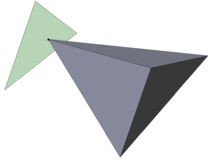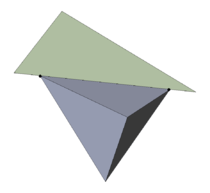(a) 1 intersection node (b) 2 intersection nodes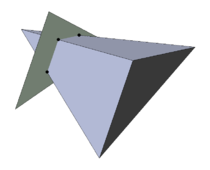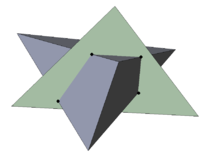(c) 3 intersection nodes (d) 4 intersection nodes Figure 55: Intersection patterns of tetrahedron-triangle-intersection - As a triangle is cutting a tetrahedron, there are principally four possible intersection patterns comprising between one and four intersection nodes.Figure 56: Definition of the structure-approximated plane - The plane to which the distances of the tetrahedron nodes are computed is defined by the surface normal vector and one of the intersection points.

The normal vector is simply the normal of the structural triangle which points into the same direction everywhere on the planar surface. By this we have all information at hand to compute the distance of the fluid nodes to the structure plane.

#### 7.1.1.3 Computation of signed distances to the plane

The shortest distance between any of the four tetrahedron nodes, let us assume e.g. ${\textstyle V_{1}}$ with the position vector ${\textstyle {\boldsymbol {v_{1}}}}$, and the structure-approximated plane can be computed by means of the following equation:

 $d_{1}={\frac {\boldsymbol {n}}{||{\boldsymbol {n}}||}}\cdot ({\boldsymbol {v_{1}}}-{\boldsymbol {p_{1}}}).$
(7.3)

The notations refer to figure 57. The presented equation can be geometrically understood as the length of the projected connection vector ${\textstyle {\overset {\rightarrow }{P_{1}V_{1}}}}$ onto the normalized normal vector. The point ${\textstyle P_{1}}$ is one of the found intersection points, i.e. one element of the set ${\textstyle I_{Tet}}$. The distance is zero if the node ${\textstyle V_{1}}$ is directly located on the surface.

Up to now we did not primarily care about the sign of the distance, which is however crucial for the location of the node relative to the structure. Moreover the distance sign establishes the basis for the recomputation of the structure what needs to be done e.g. in order to incorporate the structure interface into the governing equations of the cut fluid elements. This recomputation is done by a linear interpolation between a positive and a negative distance value in order to compute the zero-distance isosurface which characterizes the approximated structure.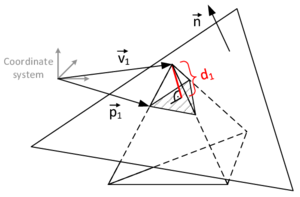Figure 57: Computation of the node distance to the plane - The perpendicular distance $d_{1}$ is the length of the projected vector ${\overset {\rightarrow }{P_{1}V_{1}}}$ onto the normal vector.

A measure for the orientation of the structure is provided by the direction of the normal vector of the structure surface. In the framework of this monograph we will define that the normal vector of the structure surface is pointing outwards. This has to be ensured when the geometry is being created in order to guarantee that the sign of the distances is calculated correctly. In case of infinitely thin structures, which do not allow a distinction between inside and outside, it has to be ensured that the normal vectors of each mesh element are uniquely pointing into the same direction.

The determination of the distance sign is already implicitly contained in equation 7.3. This will be shortly shown in the following. Considering figure 58 the angle ${\textstyle \alpha }$ between the normal vector ${\textstyle {\boldsymbol {n}}}$ and the connection vector